# R S Aggarwal Solutions for Class 11 Maths Chapter 3- Functions

The R S Aggarwal Solutions for the questions given in Chapter 3, Functions, of the Class 11 R S Aggarwal textbooks are available here. In this chapter students learn about the topics related to Functions, including the domain, co-domain, pictorial representation and range of functions. Students are often advised to solve all the questions present in the R S Aggarwal textbook, along with the NCERT textbook, to score full marks in the exams.

The Class 11 R S Aggarwal Solutions for Maths is very easy to comprehend and covers all the exercise questions included in the book. Here, the PDF of the Class 11 Maths Chapter 3 R S Aggarwal Solutions is available, which can be downloaded as well as viewed online. Students can also avail the R S Aggarwal Solutions and download it for free to practice offline as well.

### Access Answers to Maths R S Aggarwal Problems of Class 11 Chapter 3 – Functions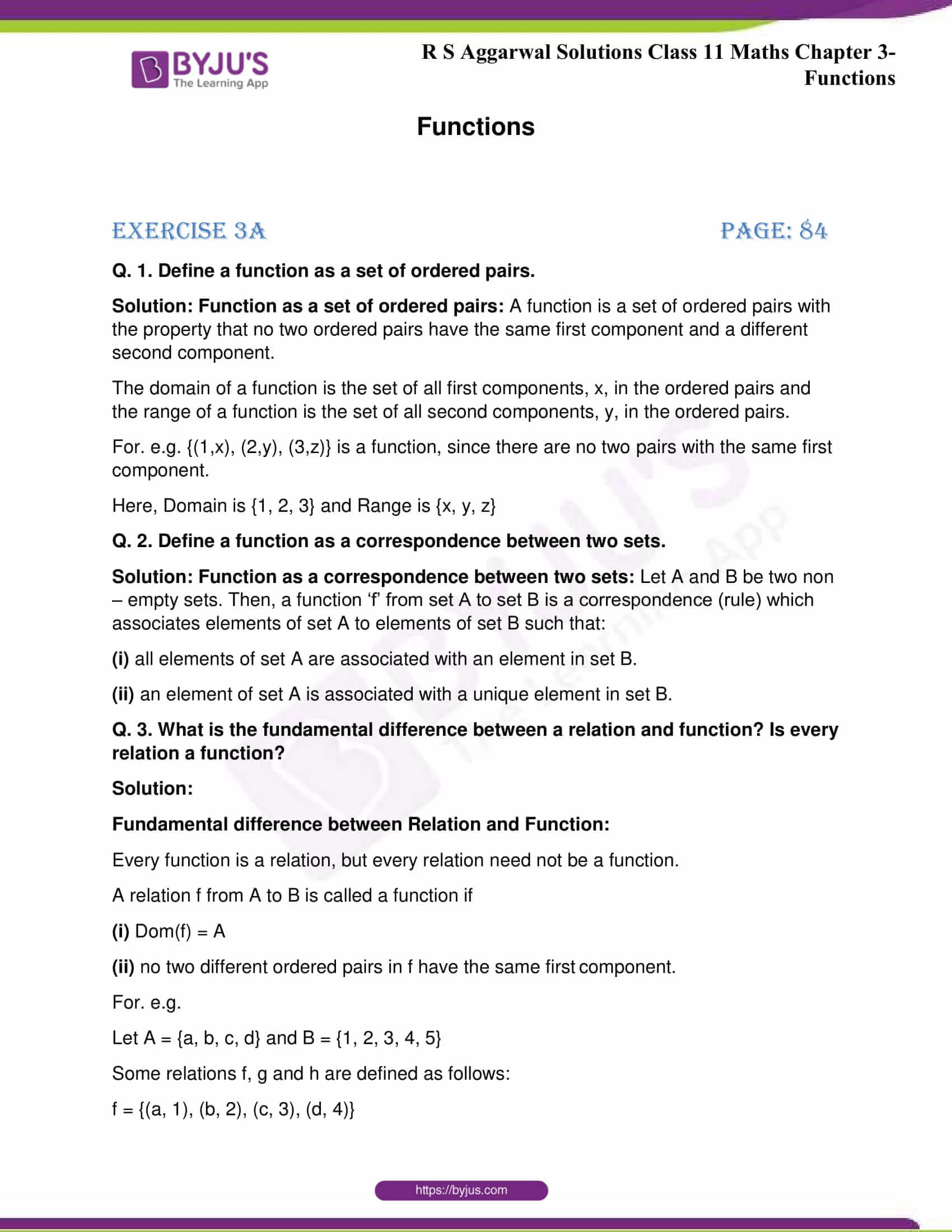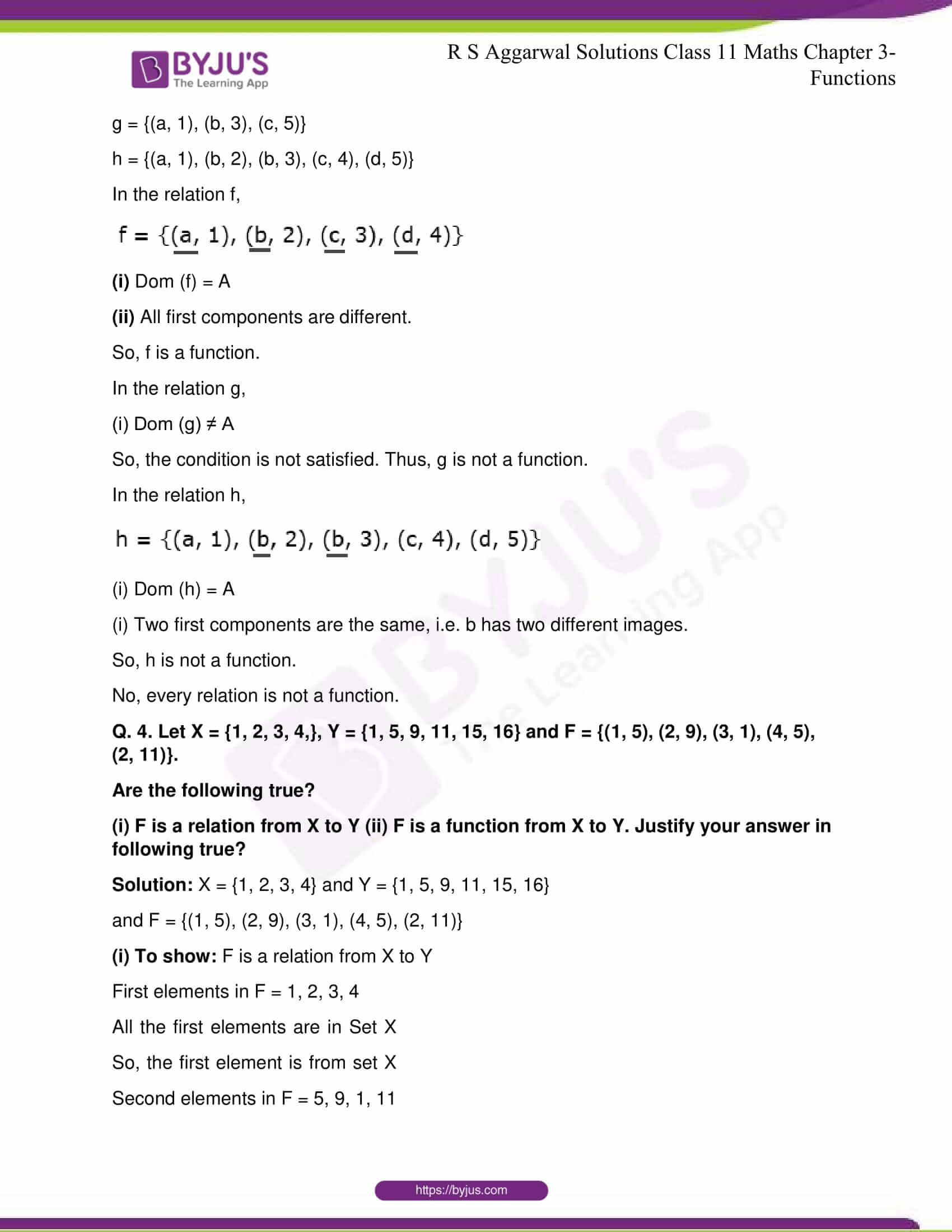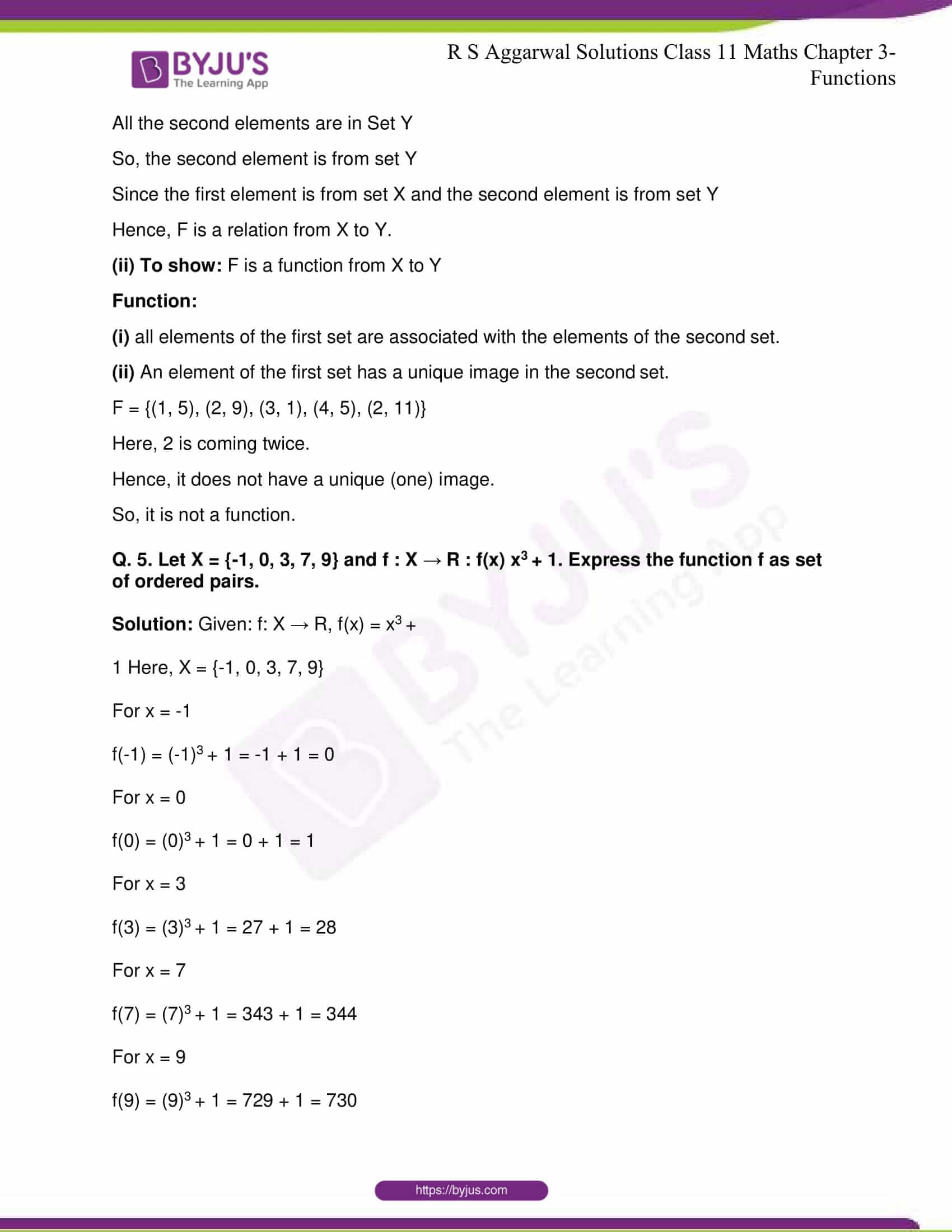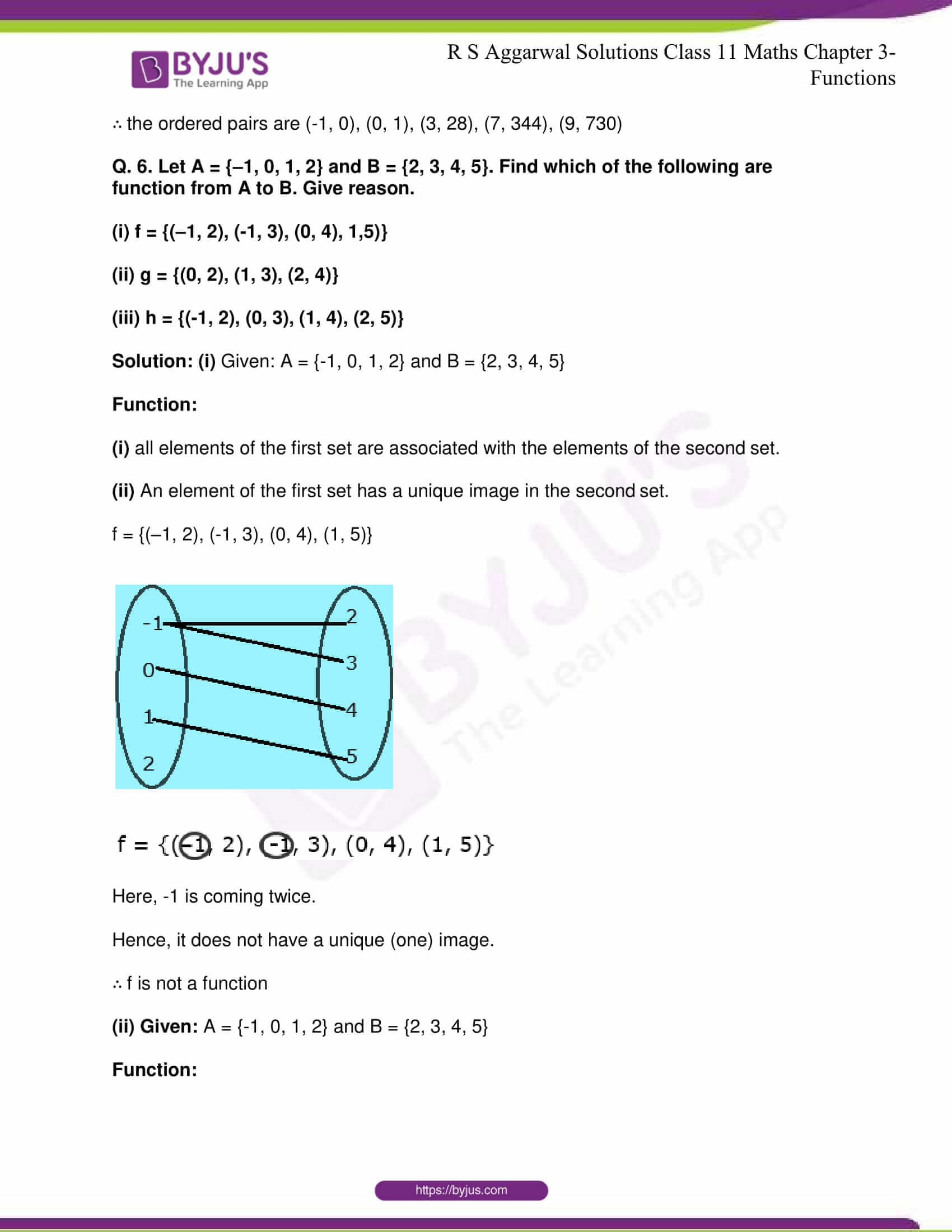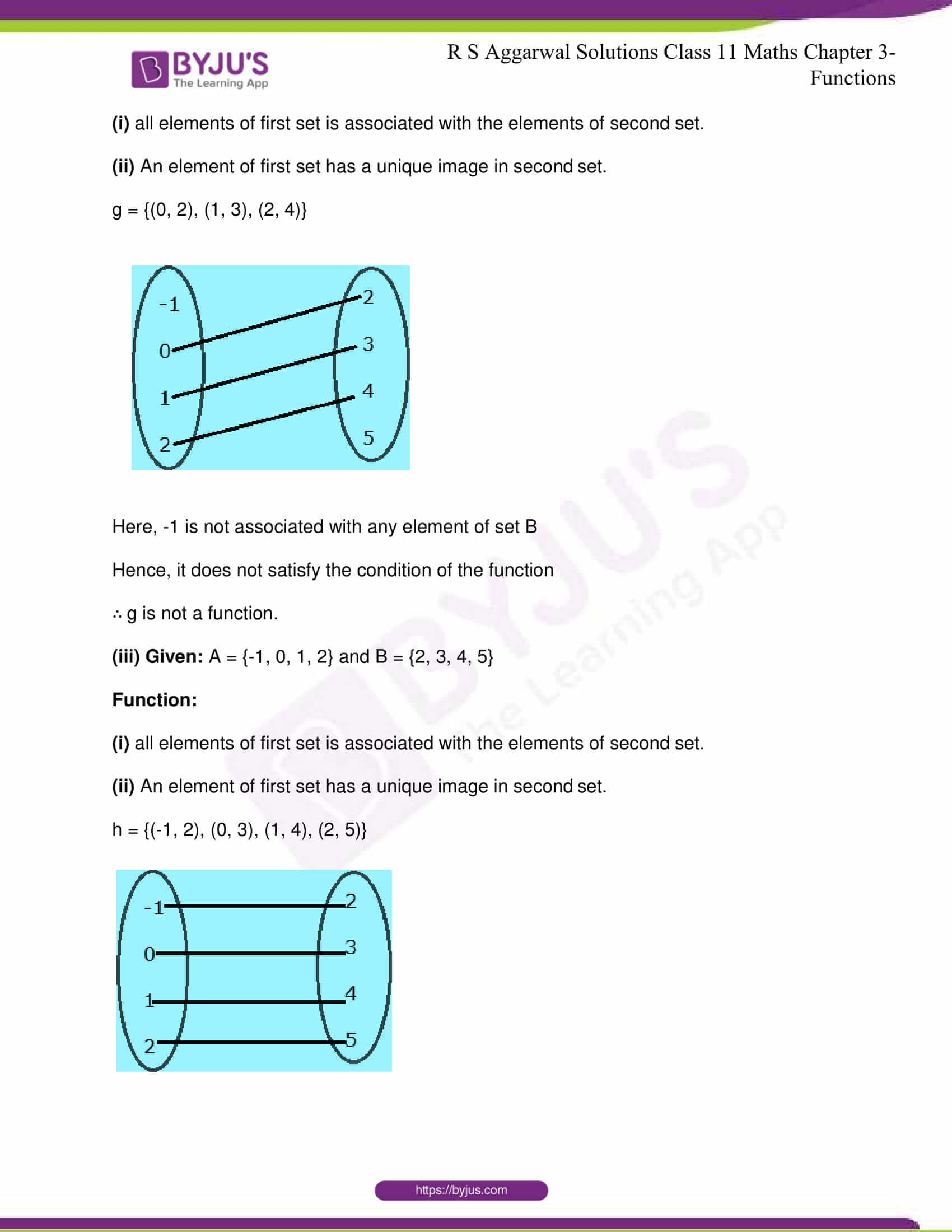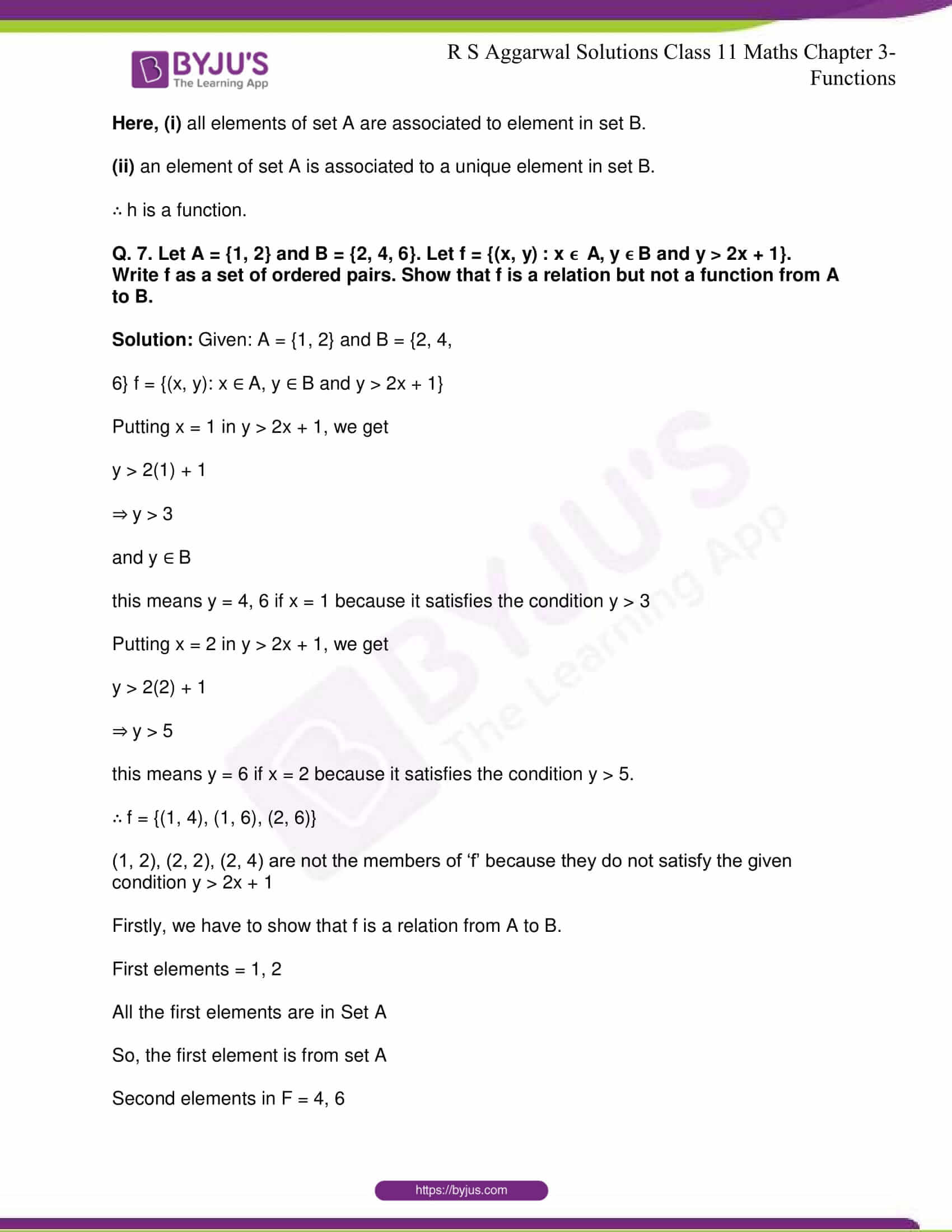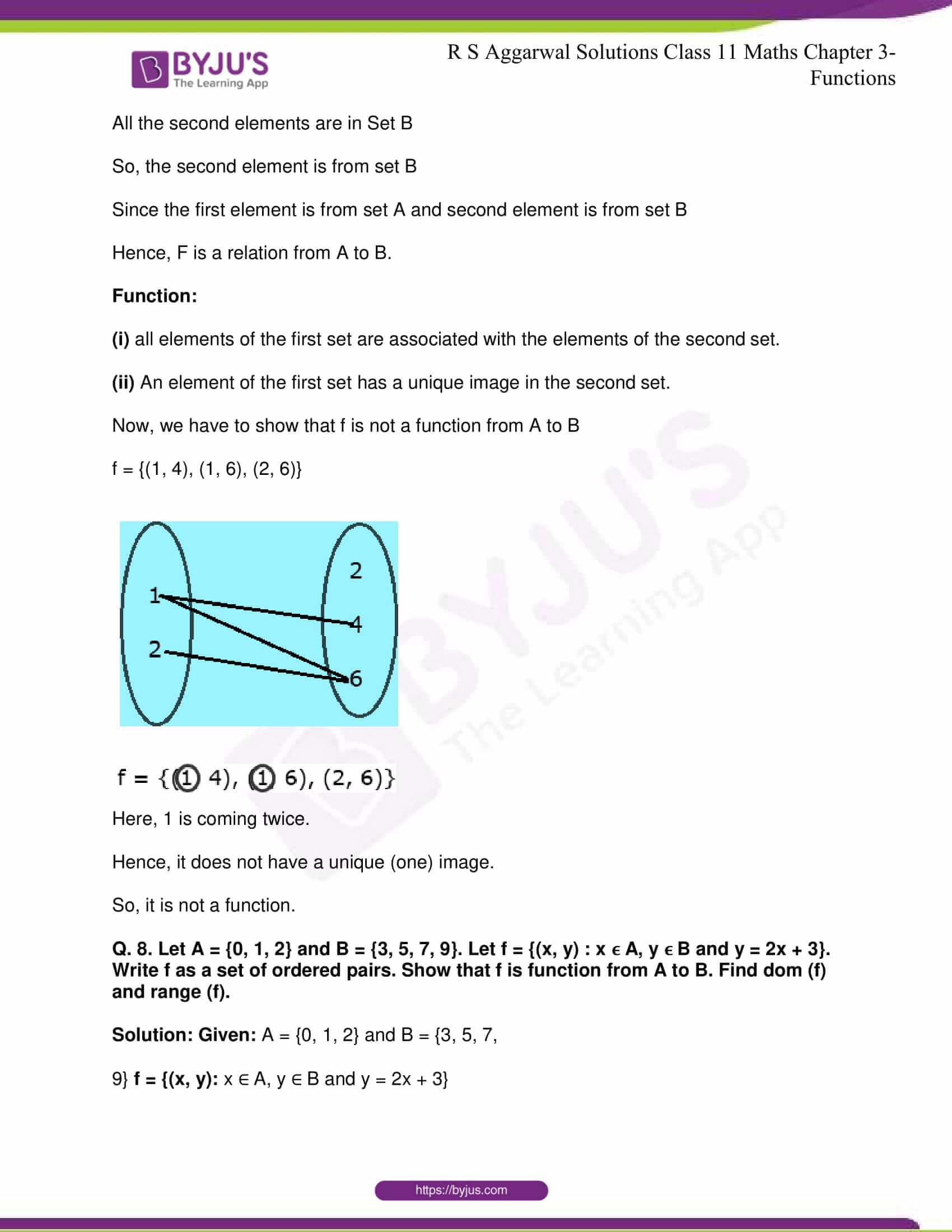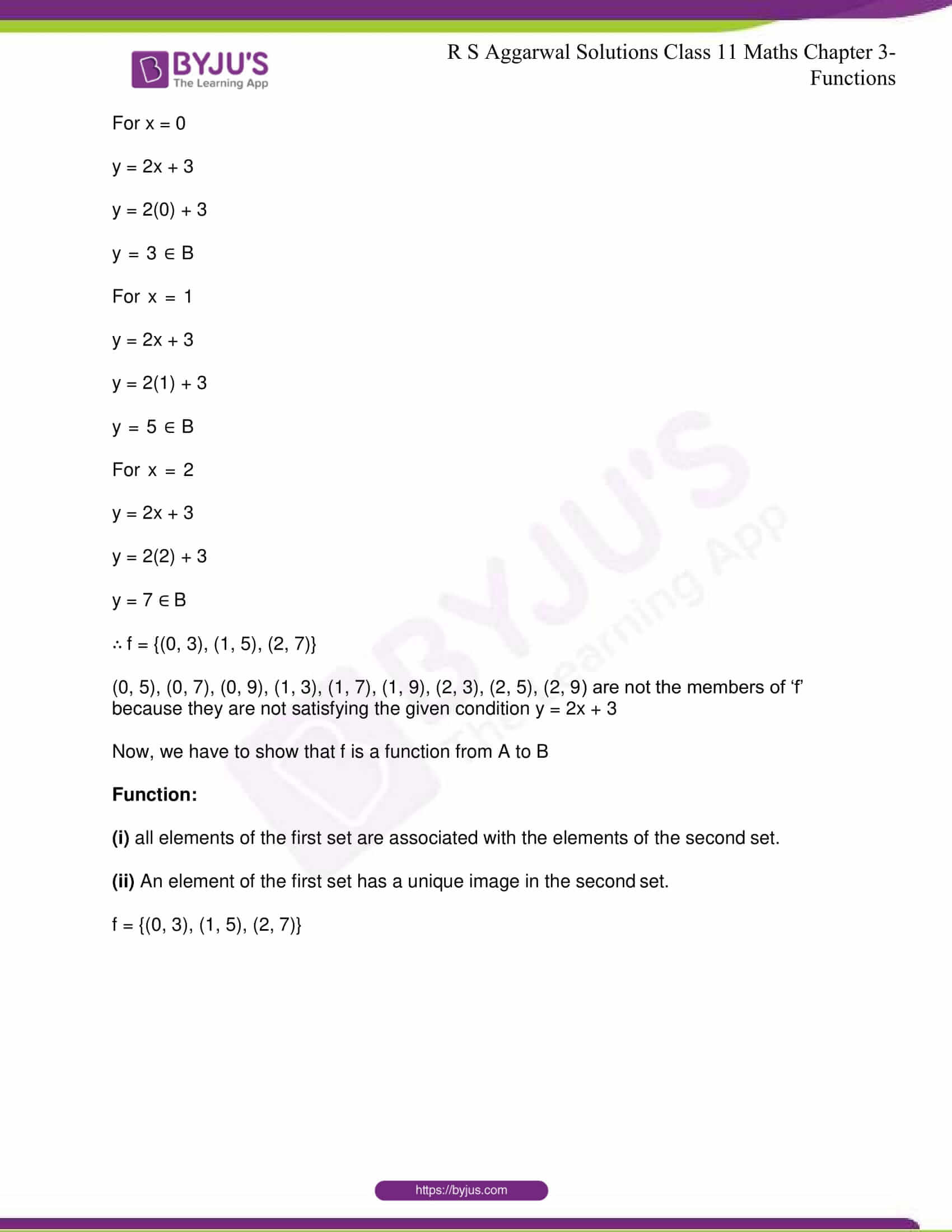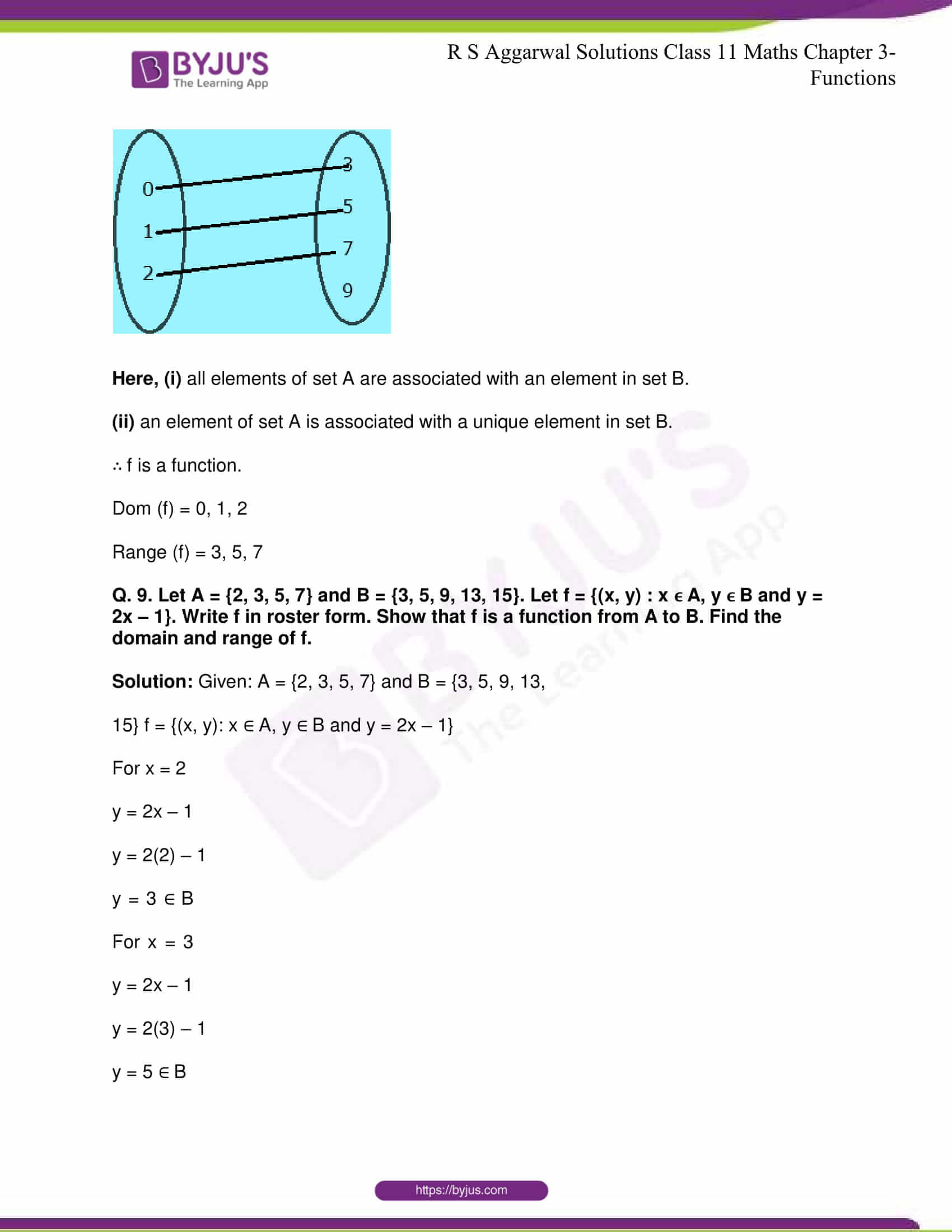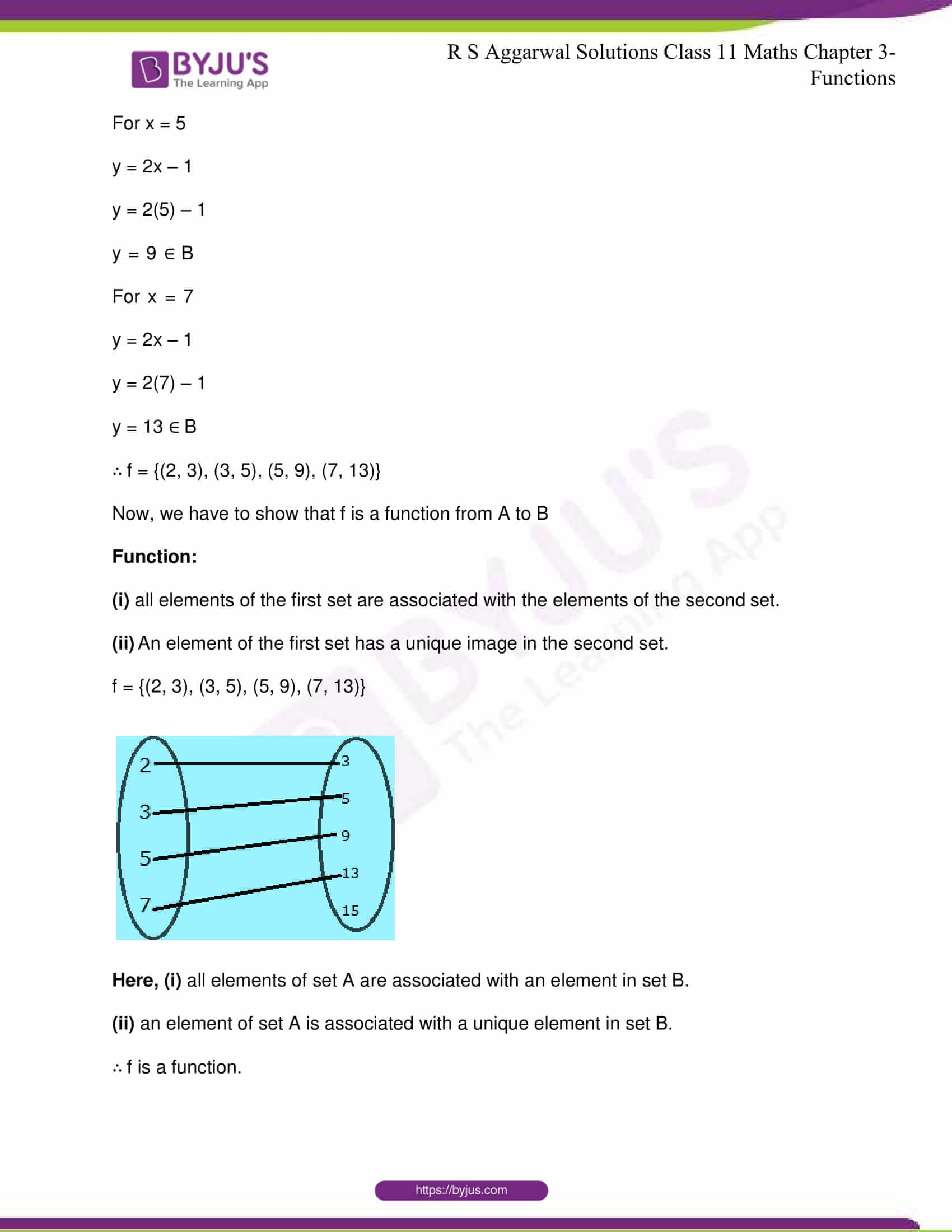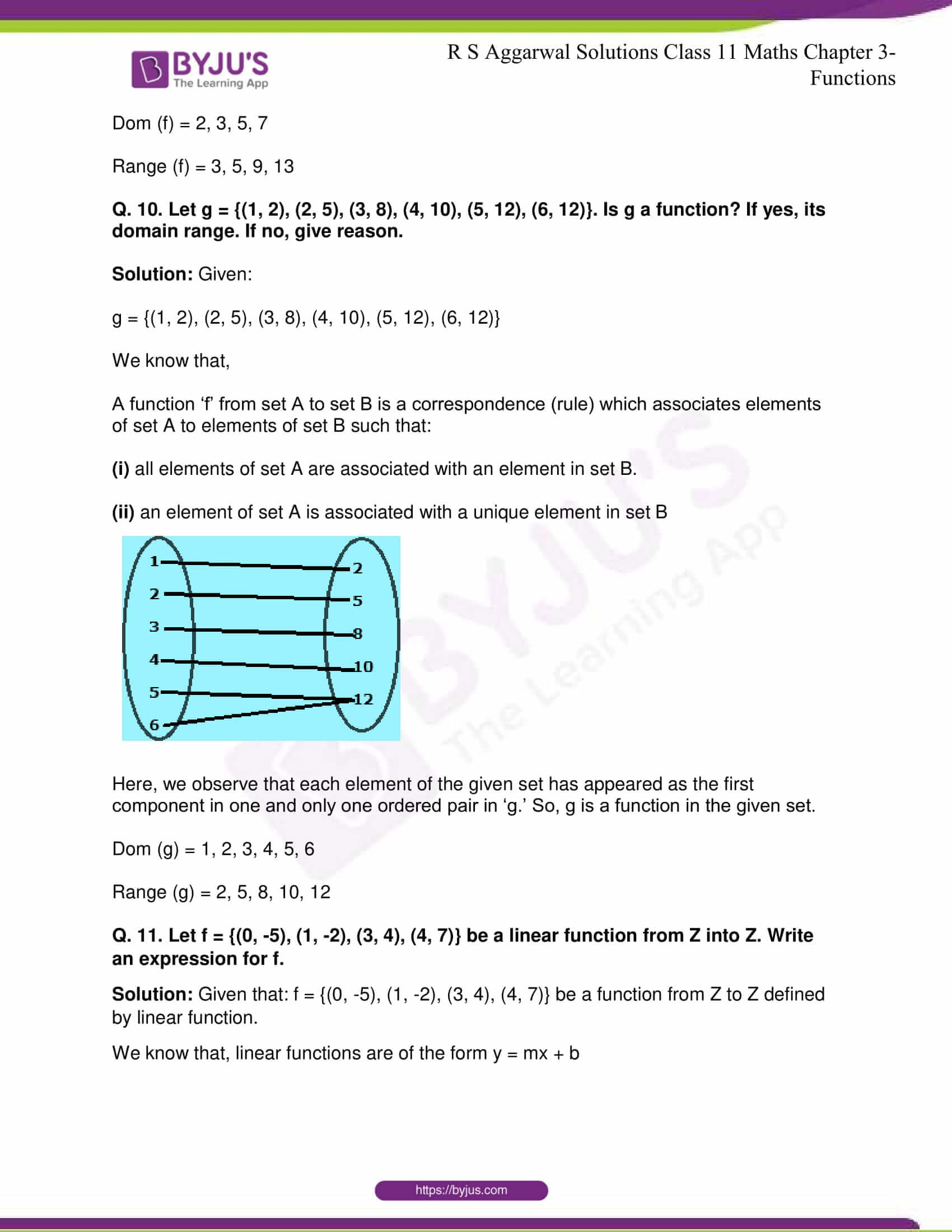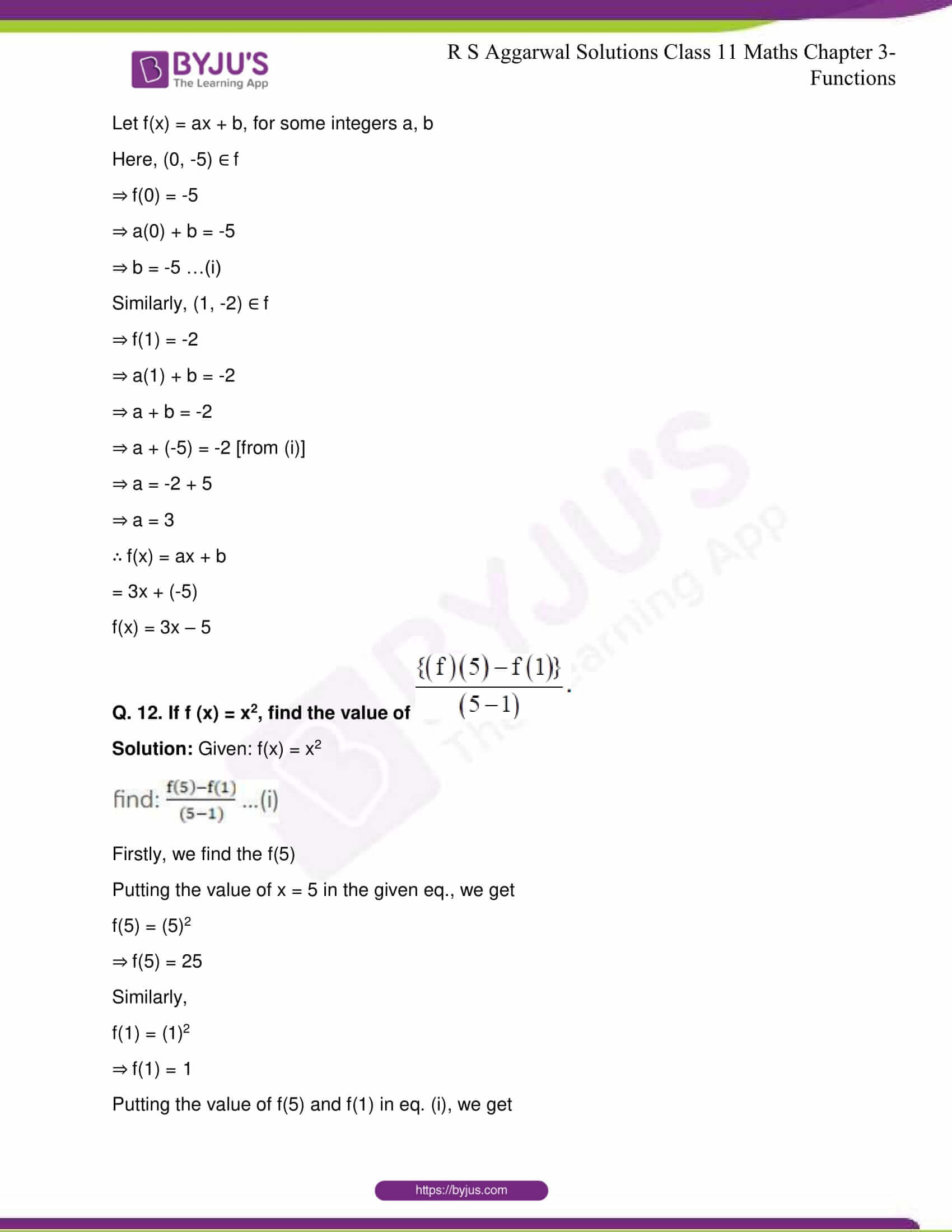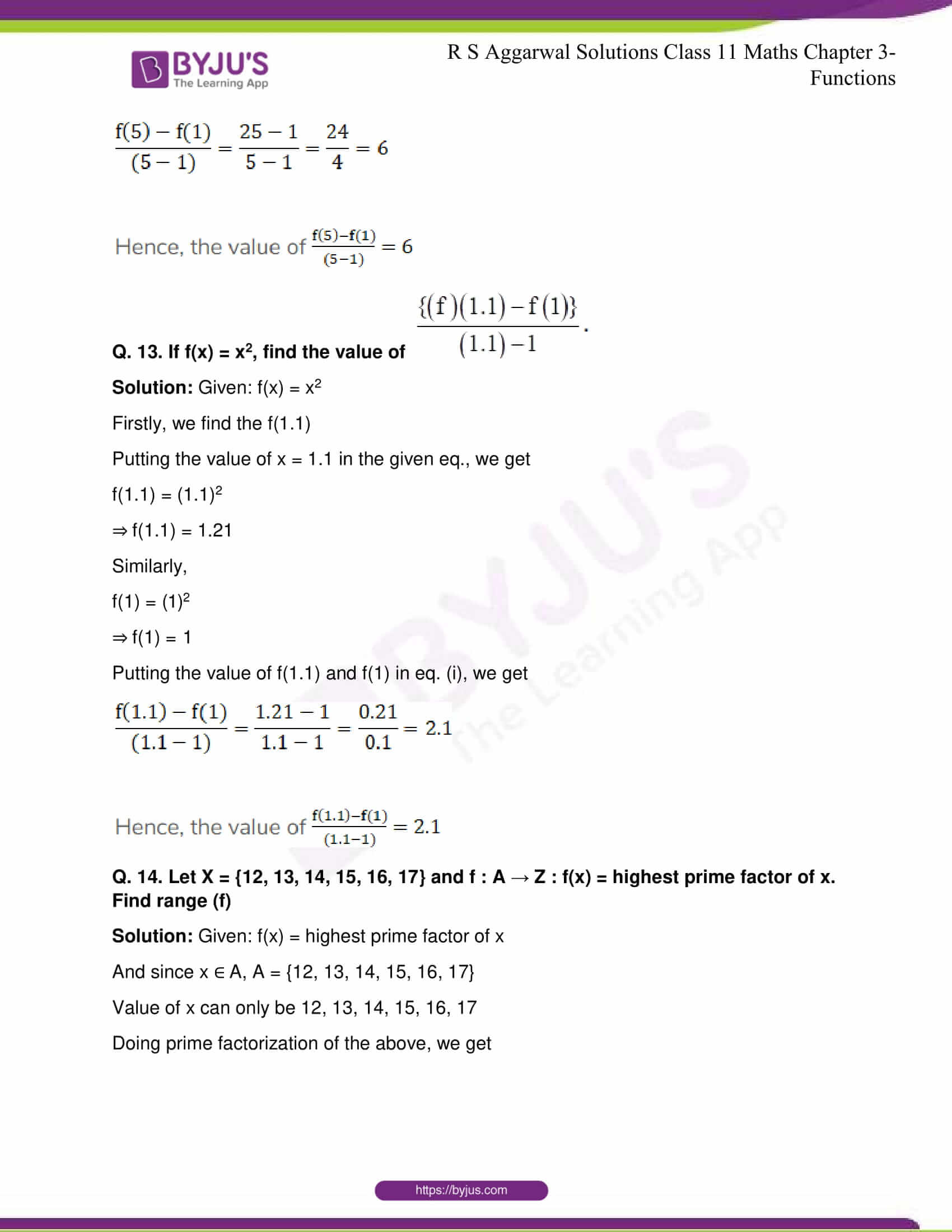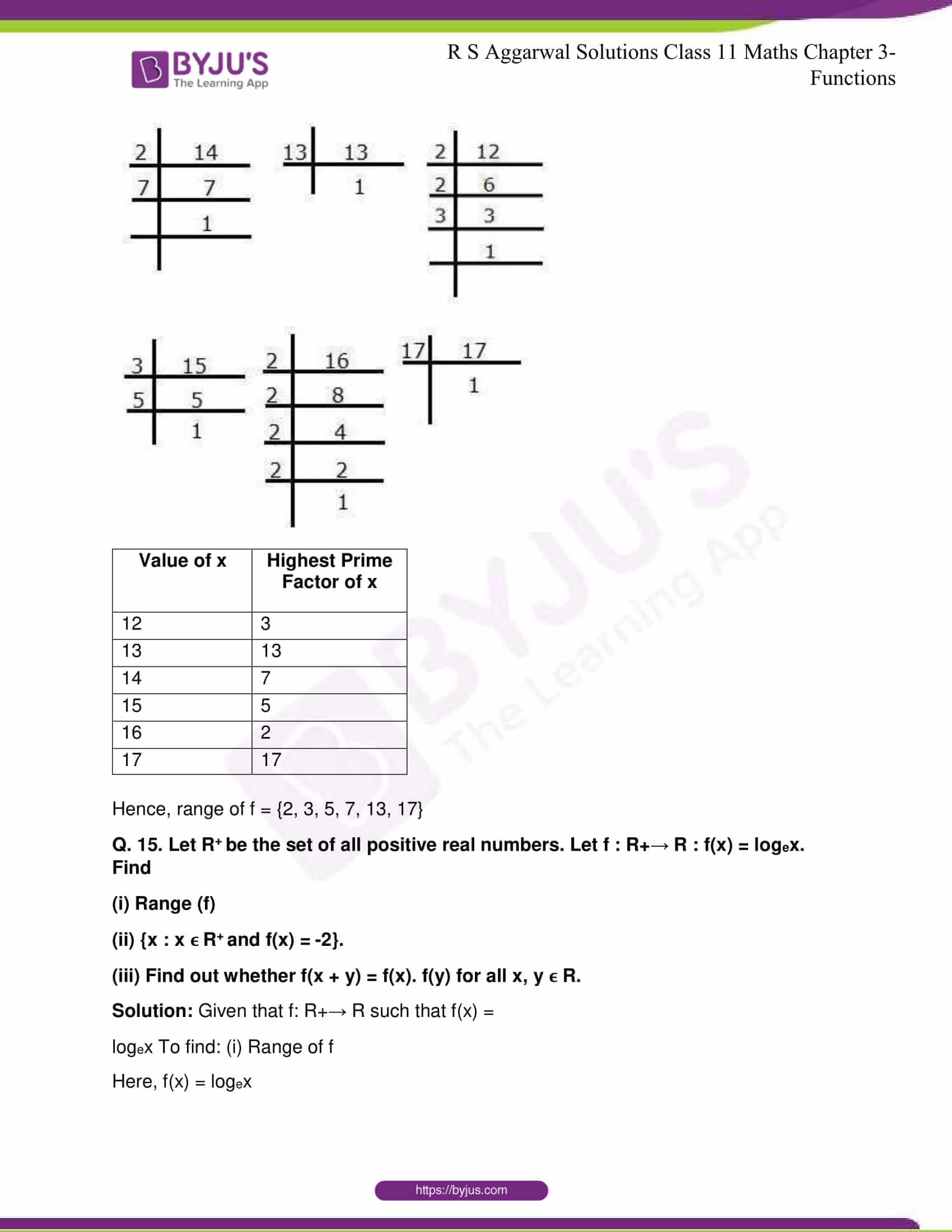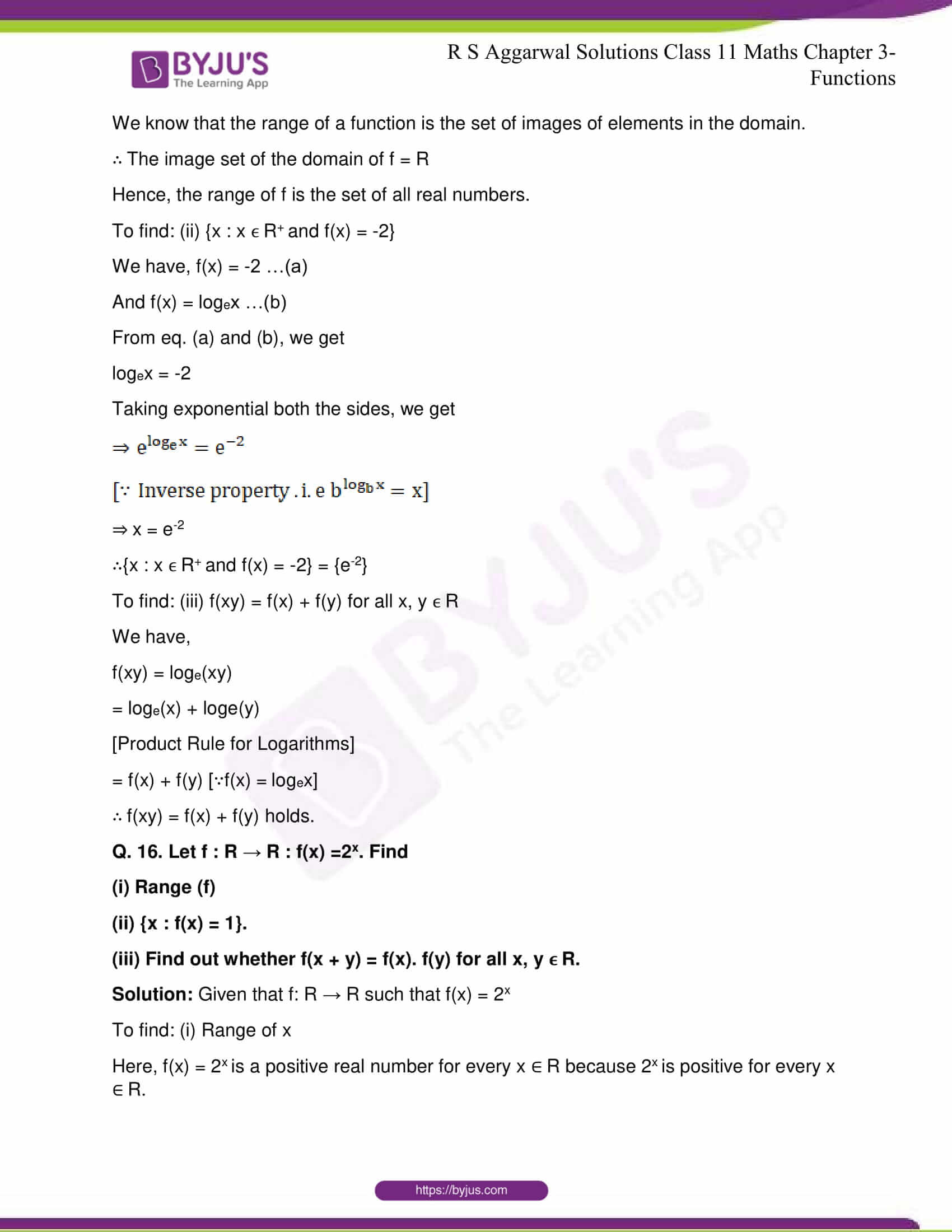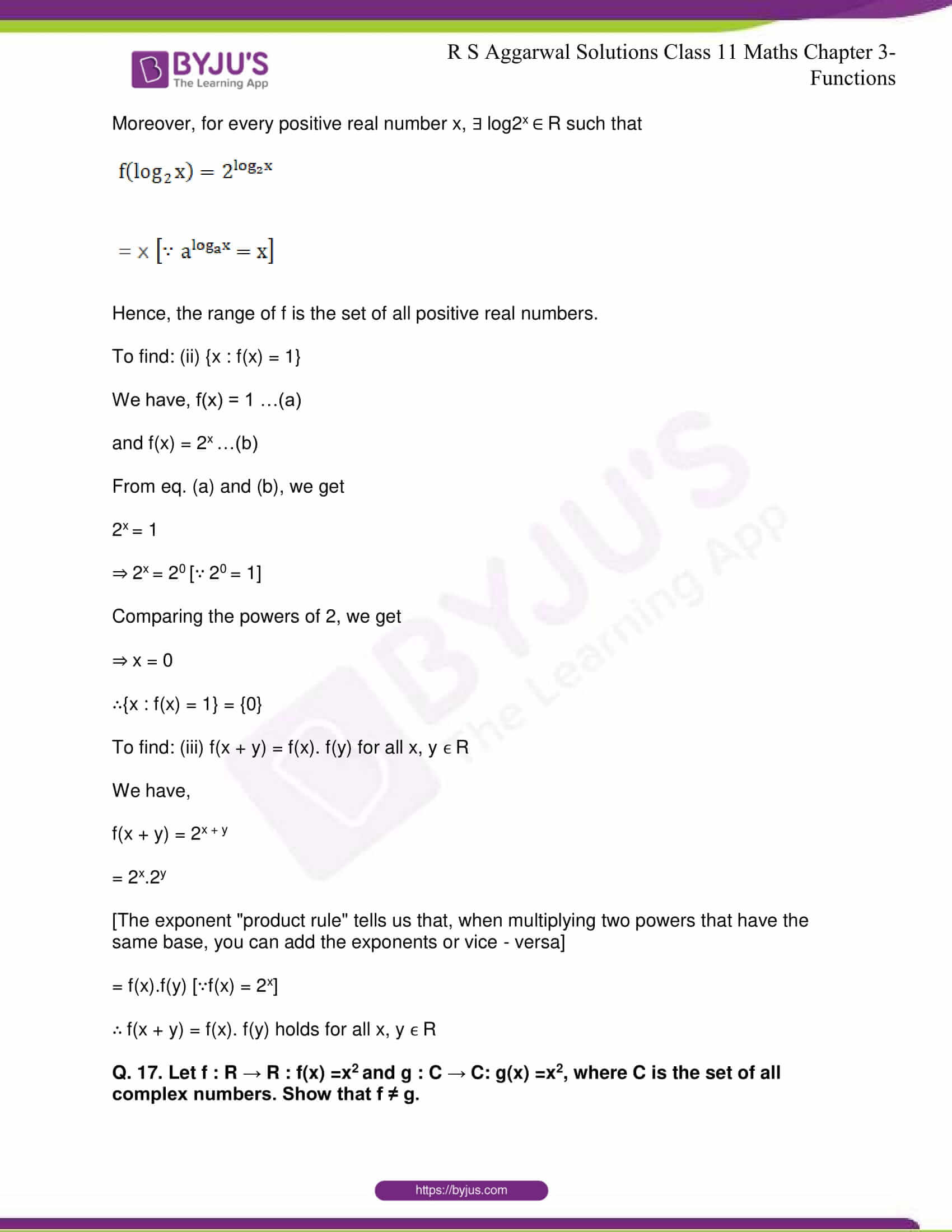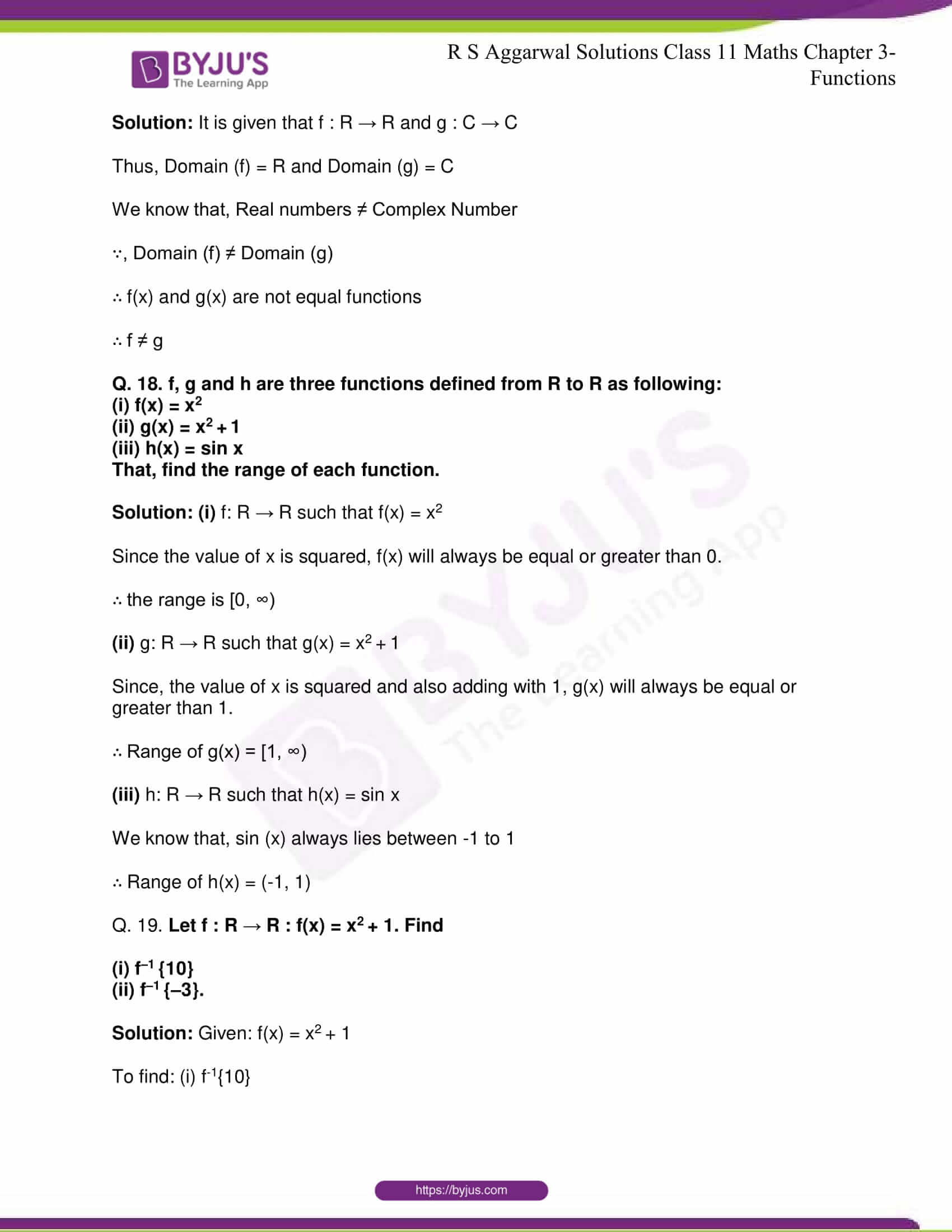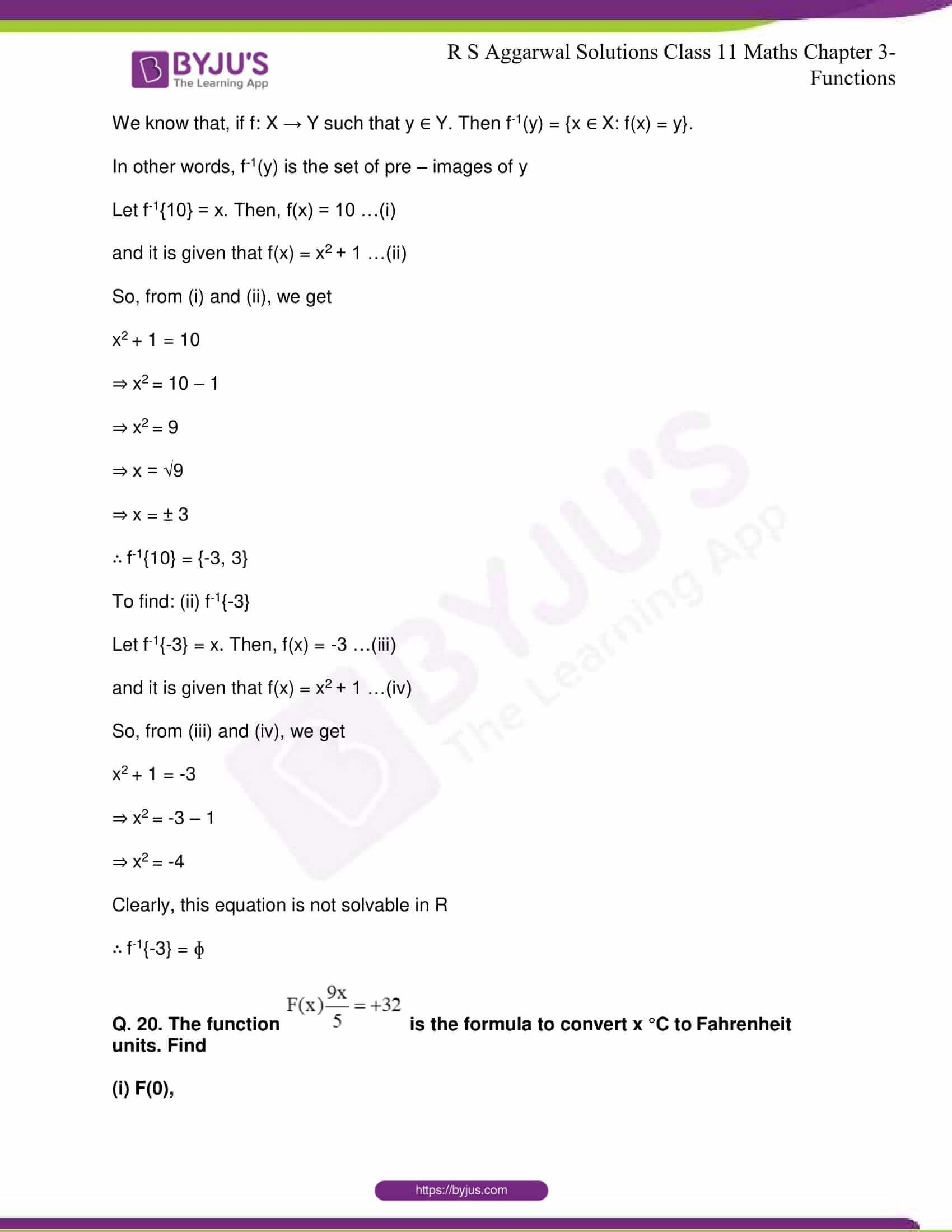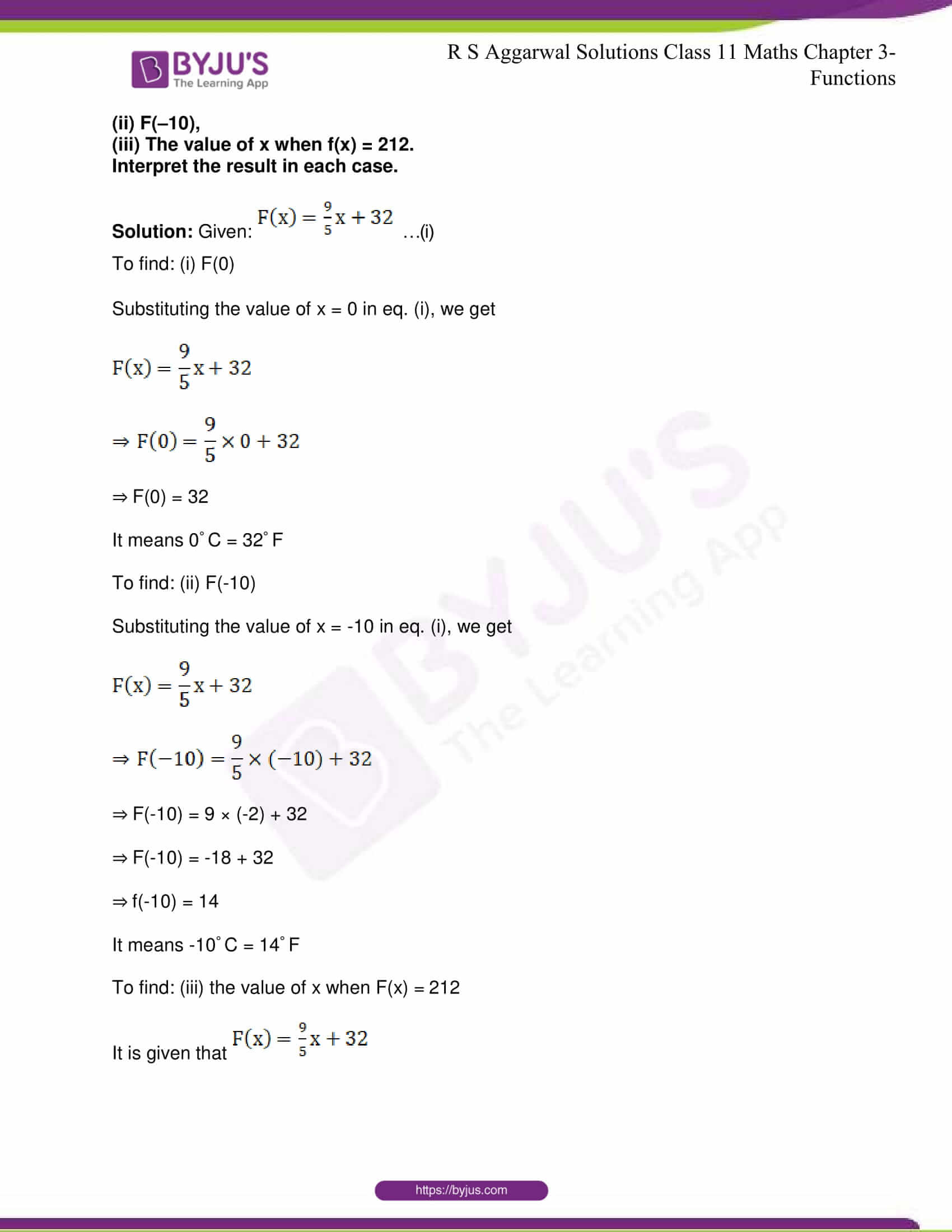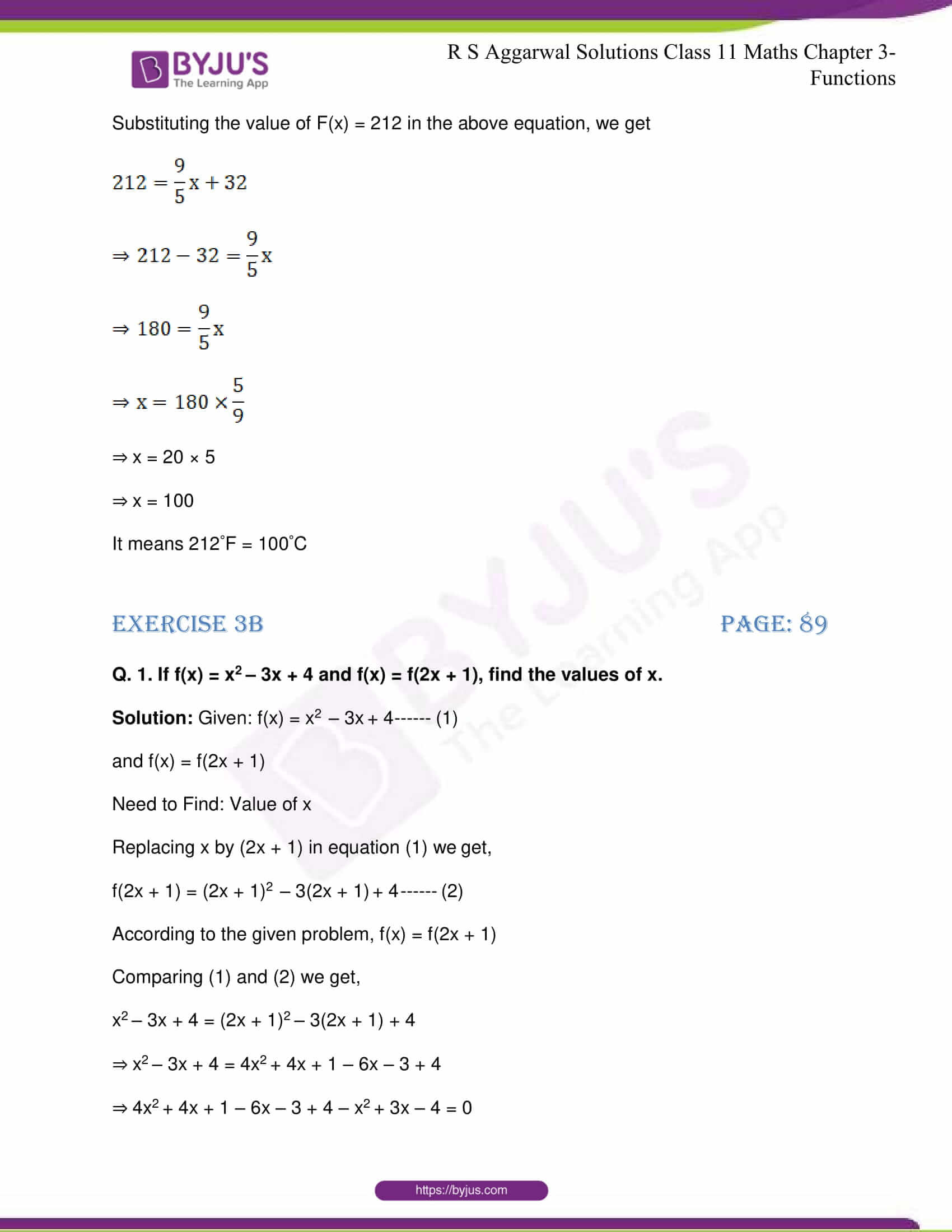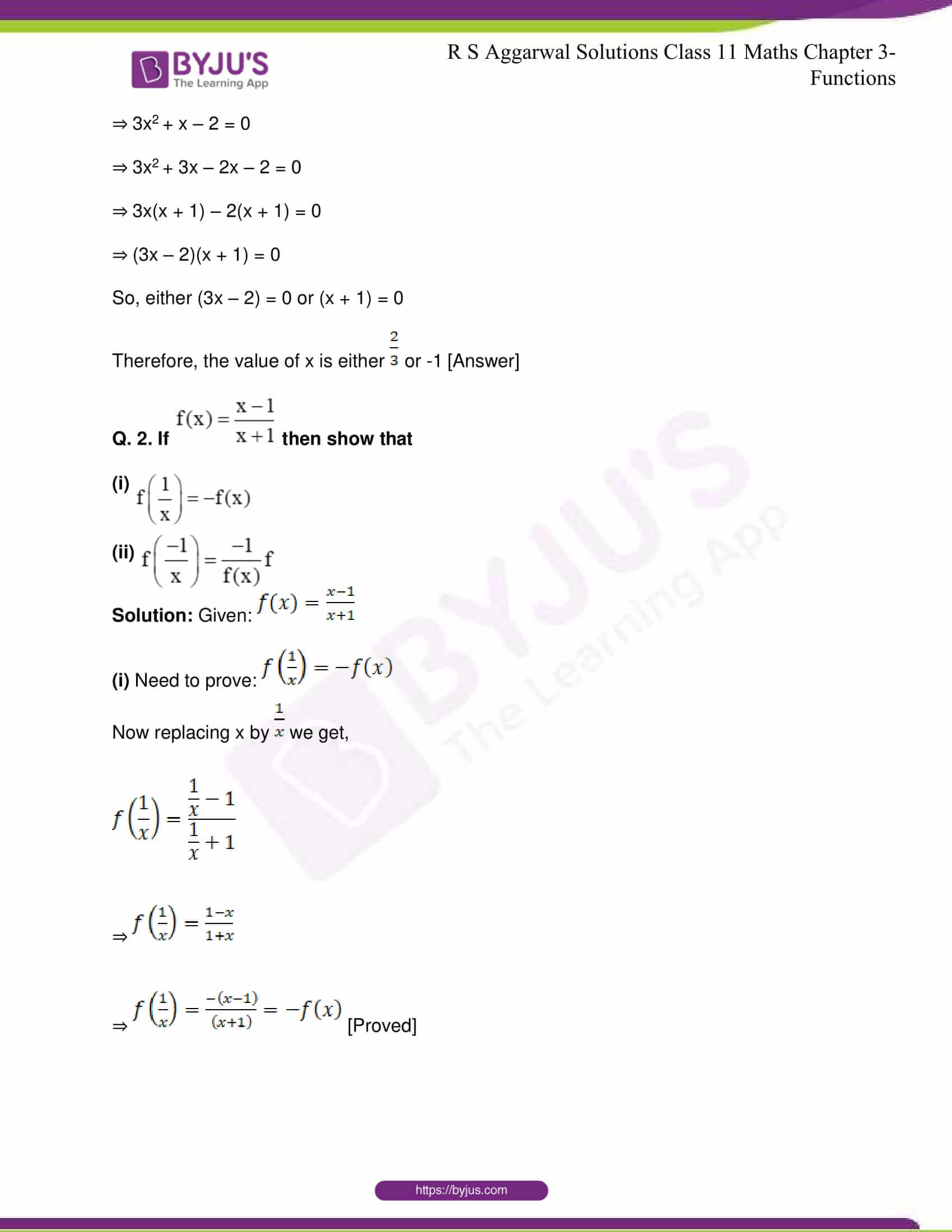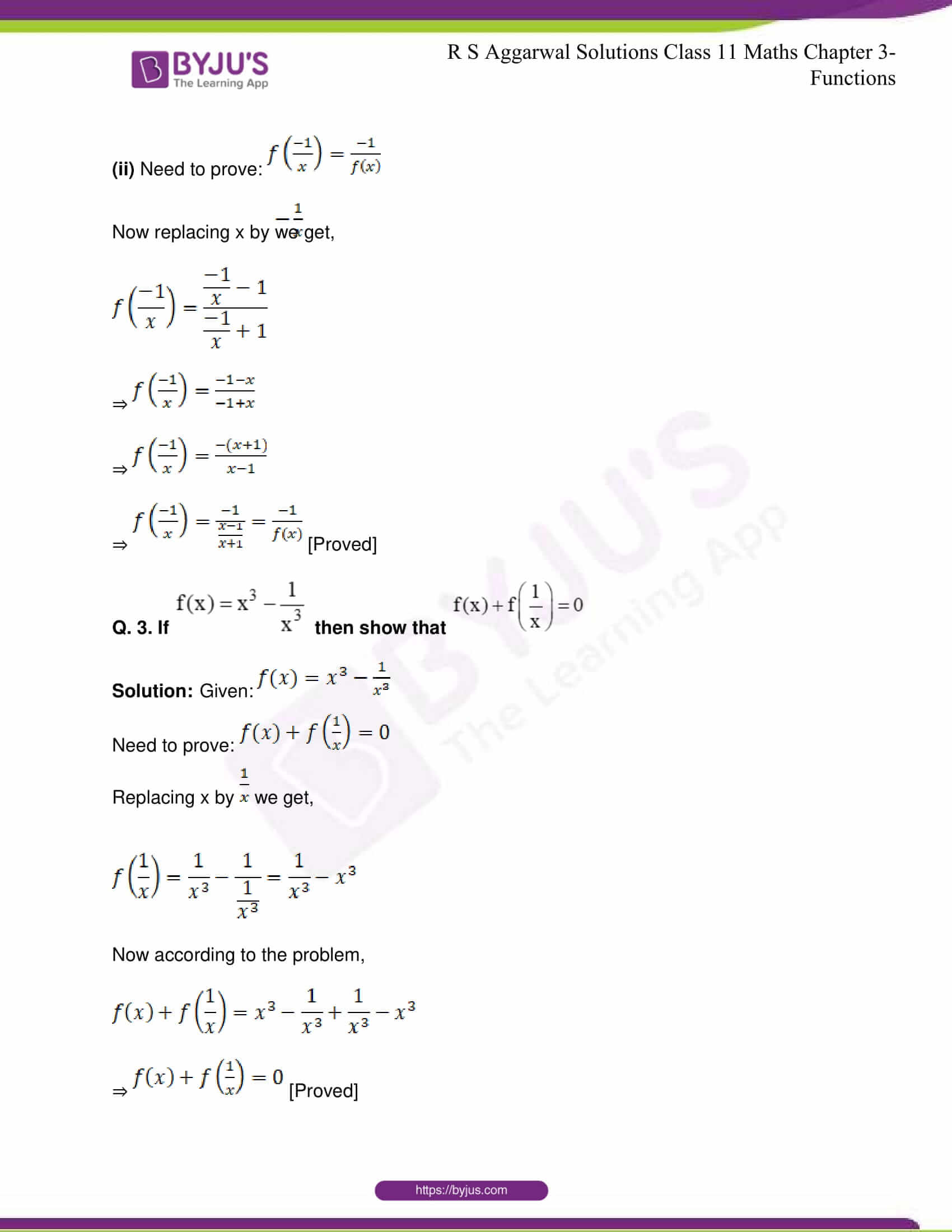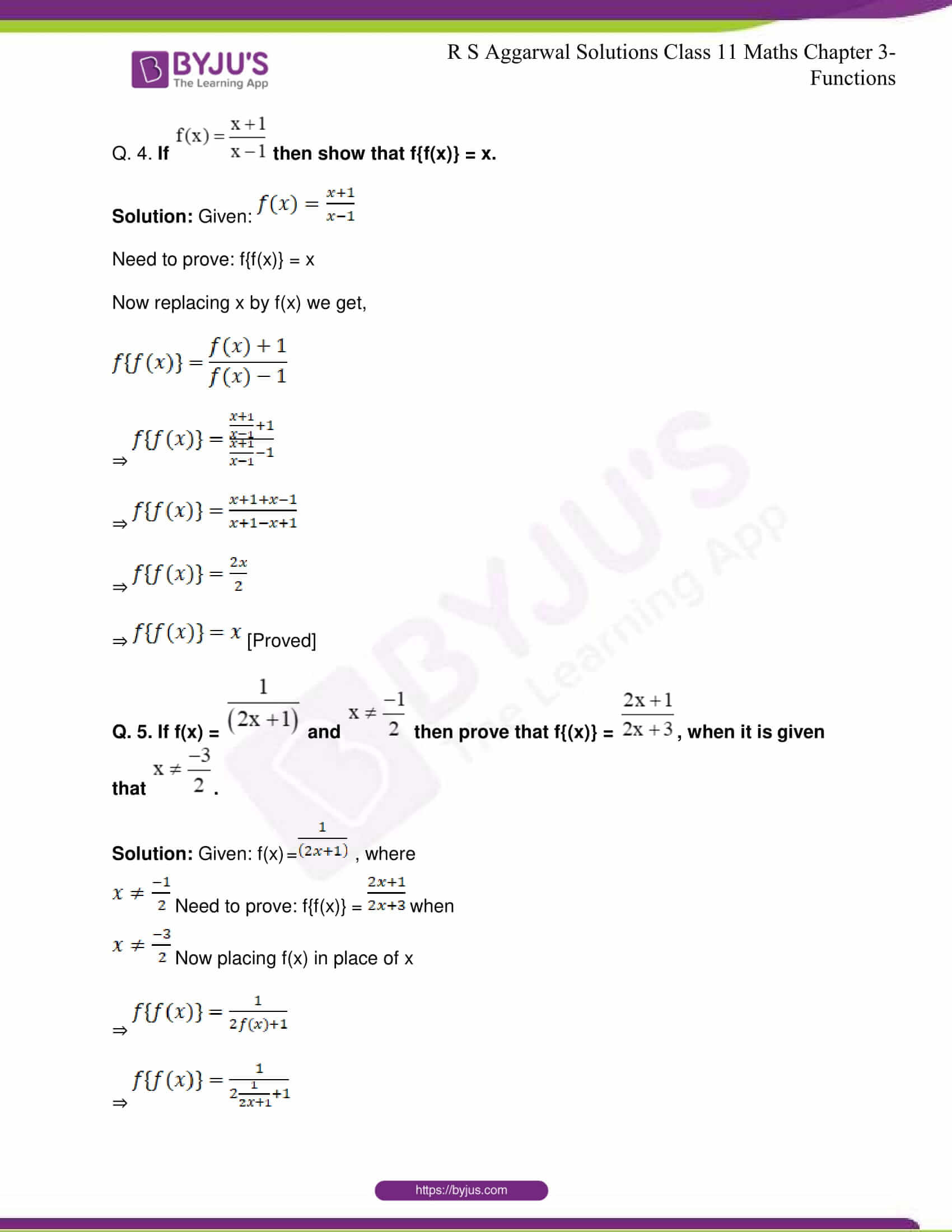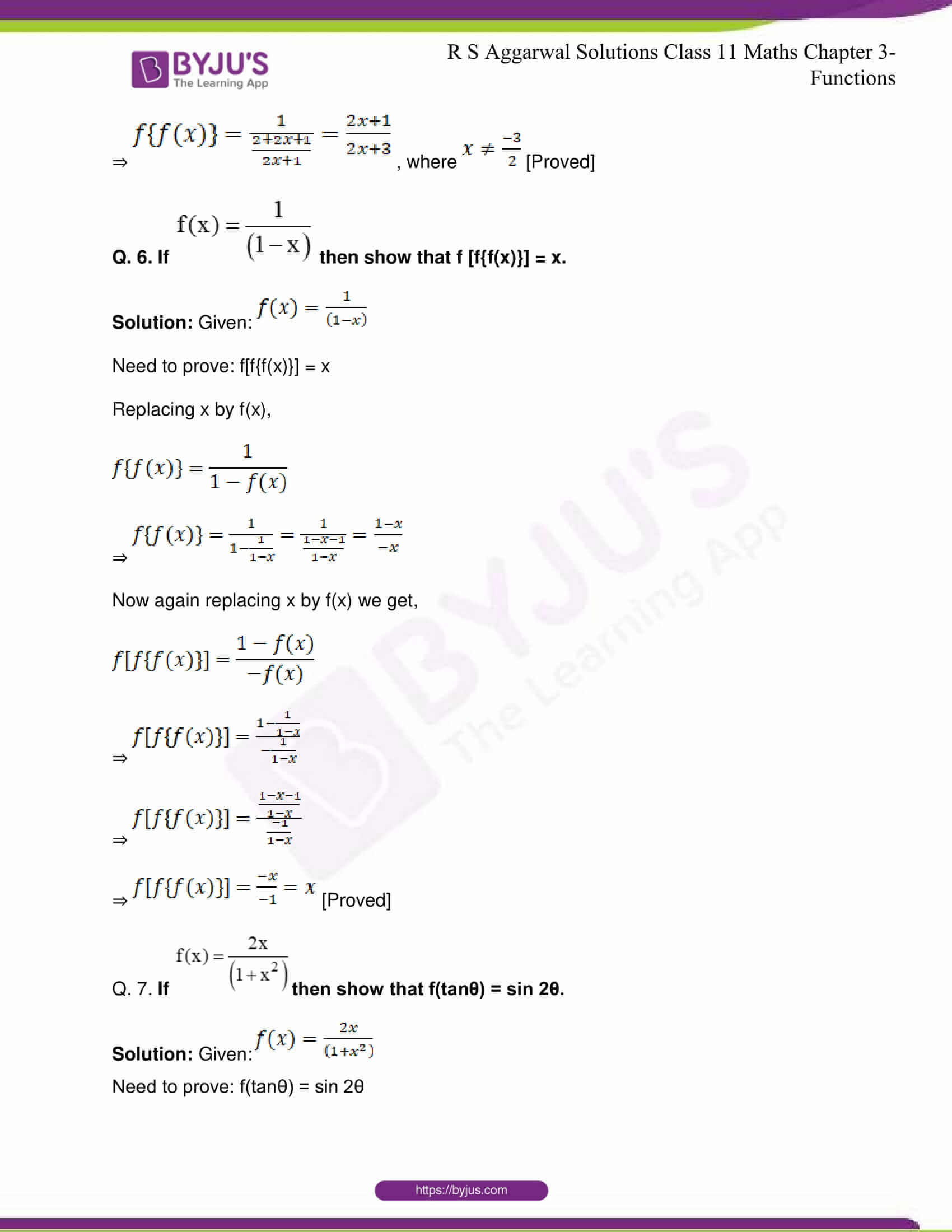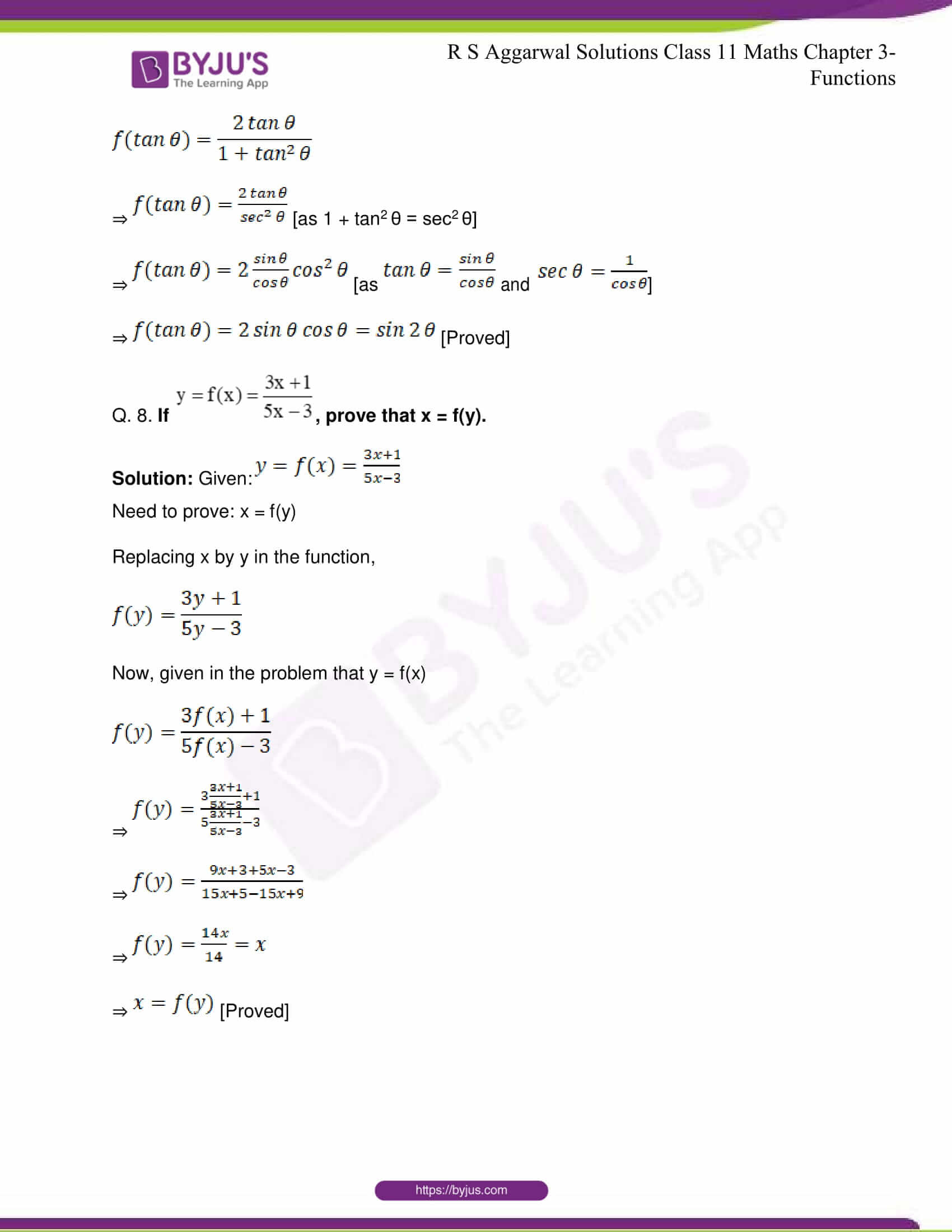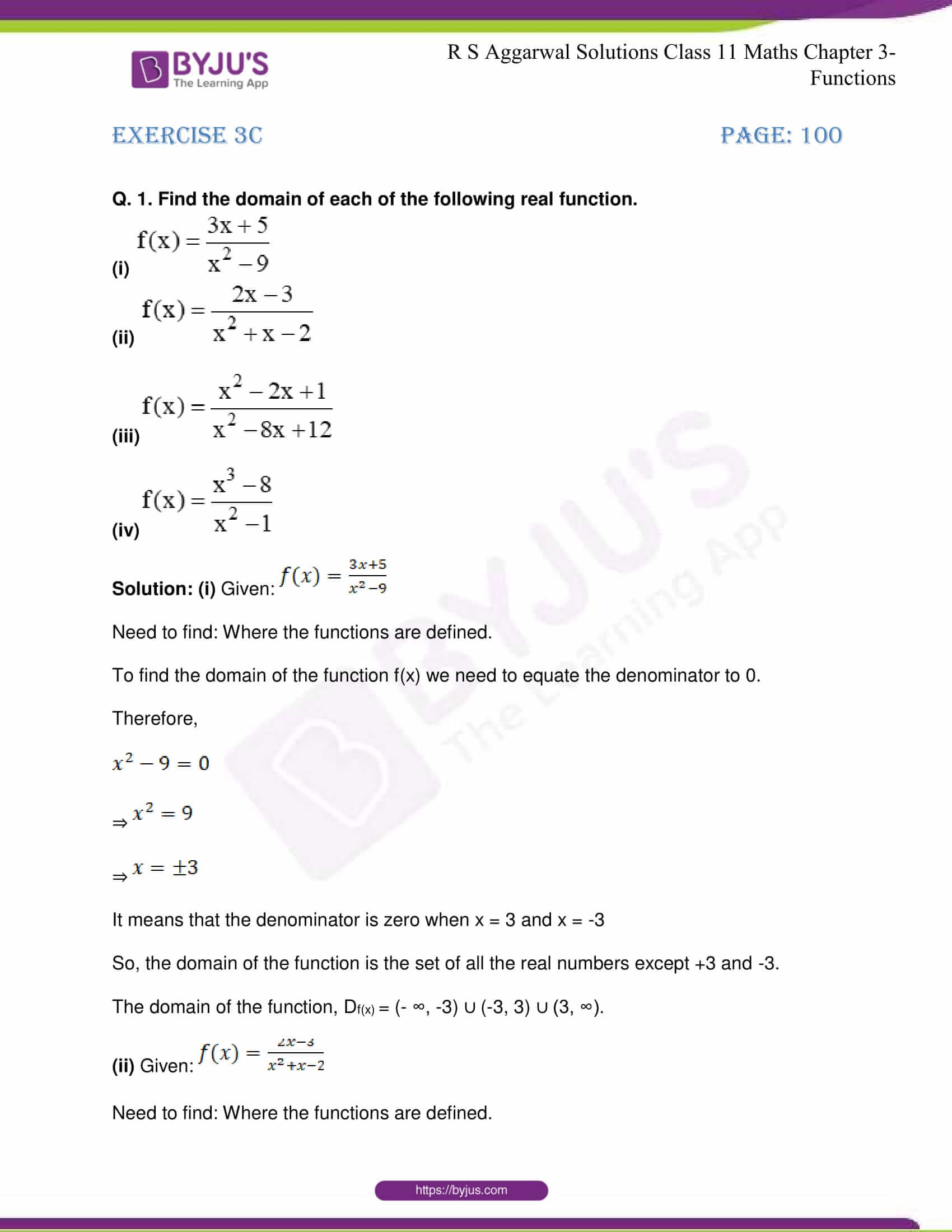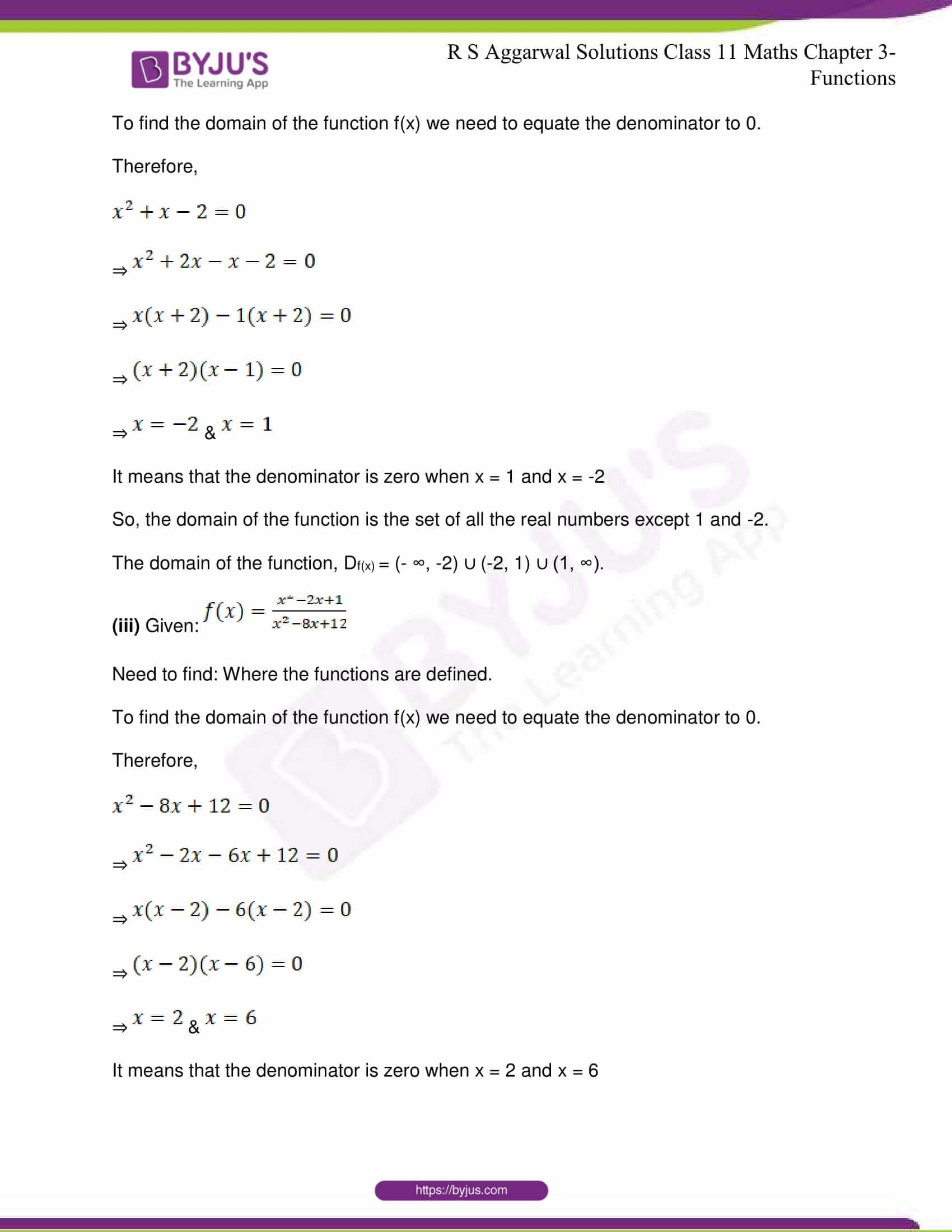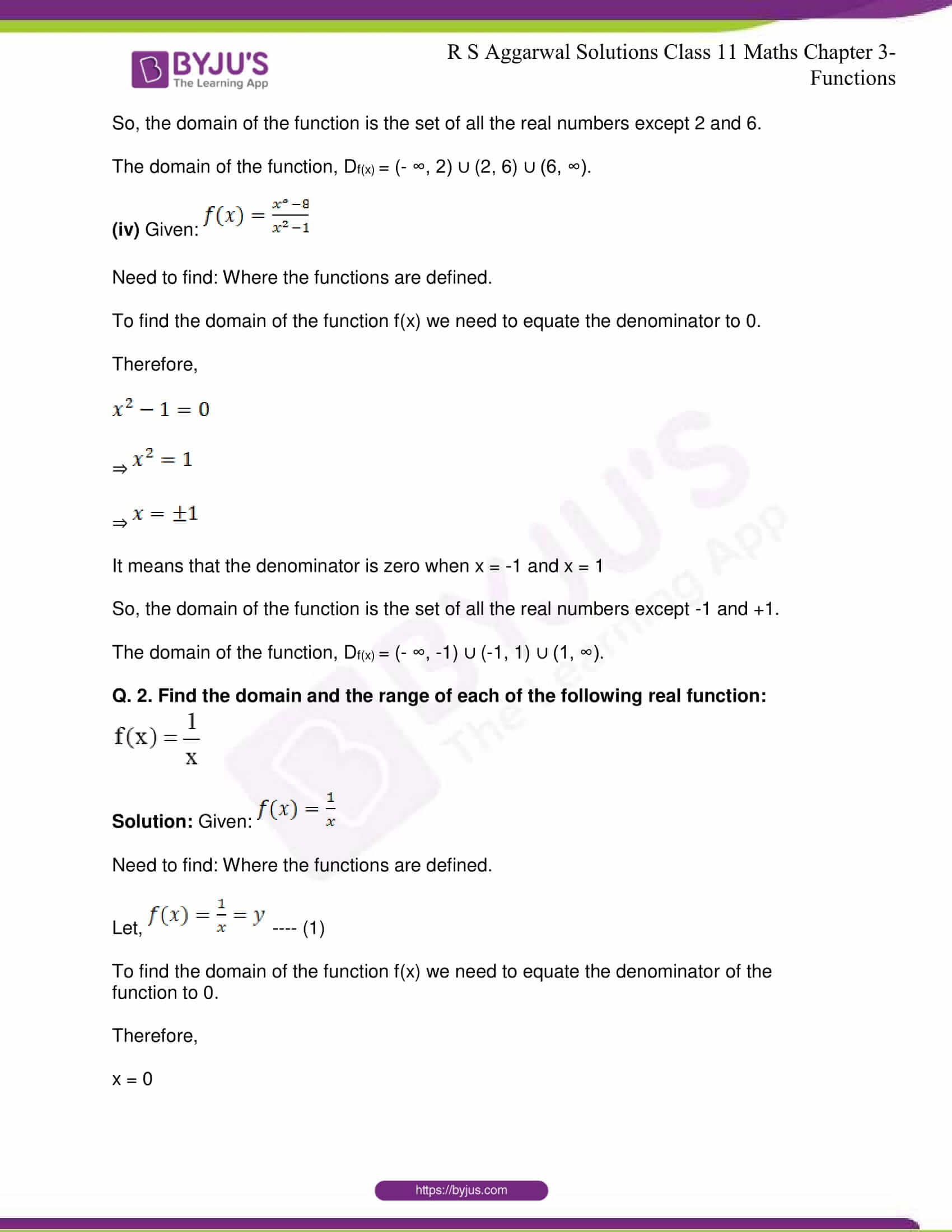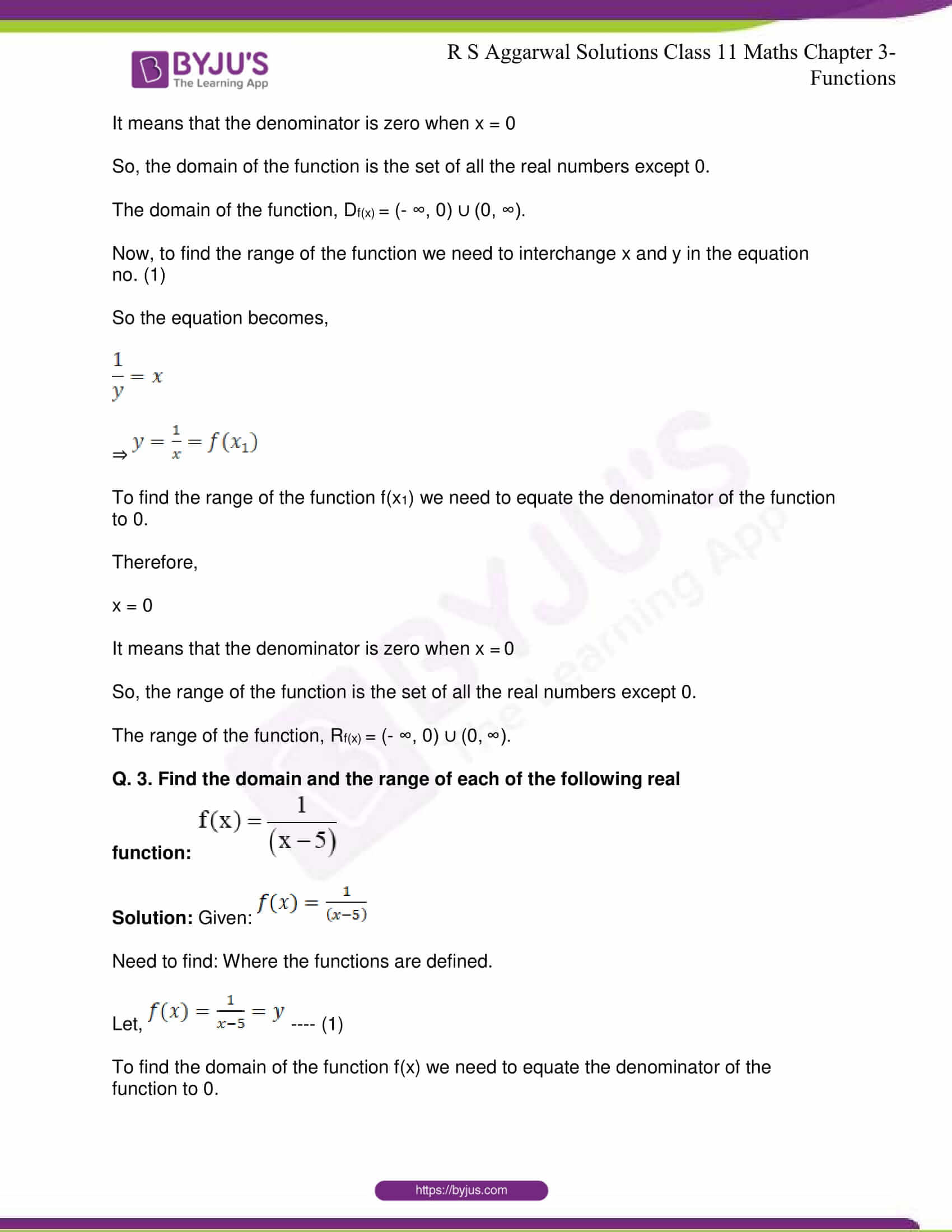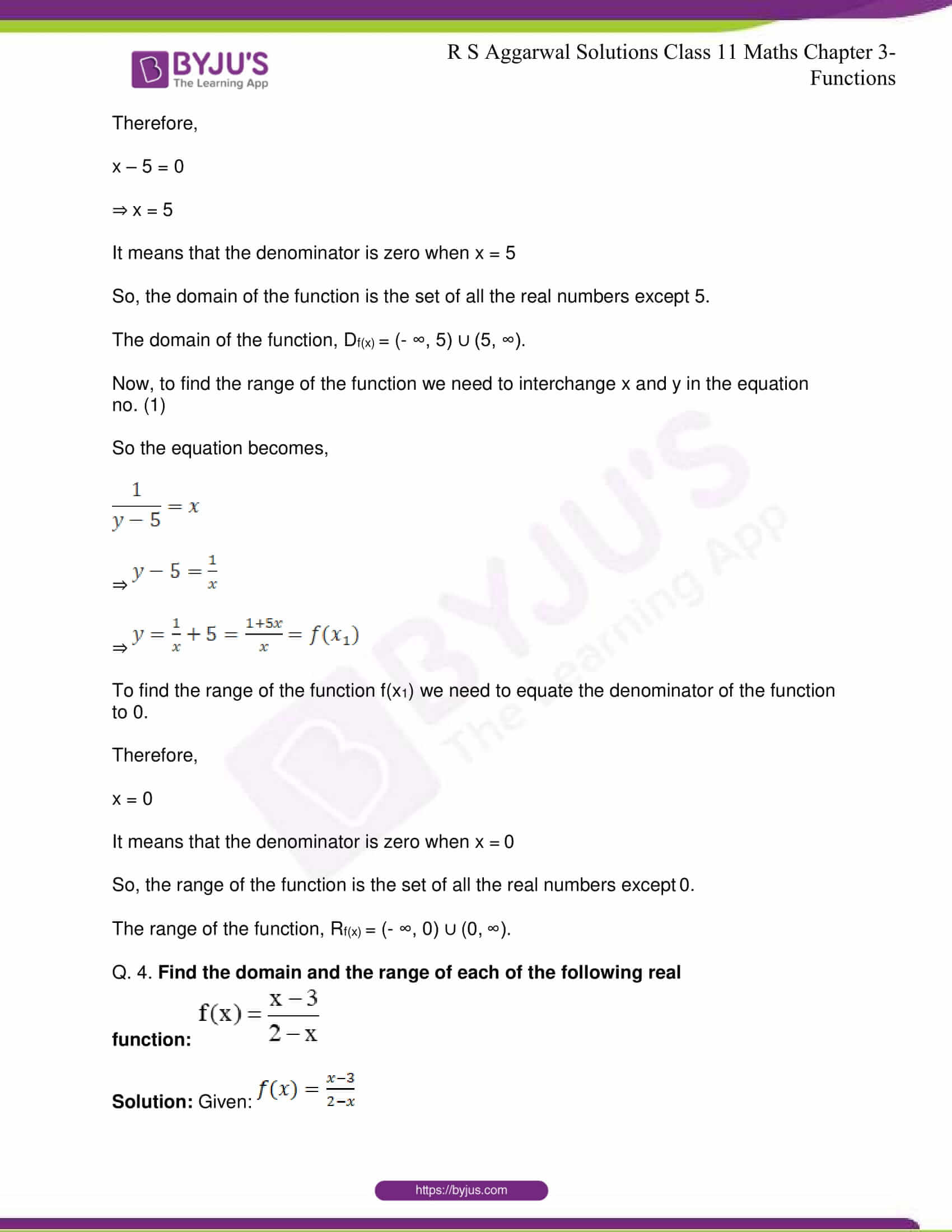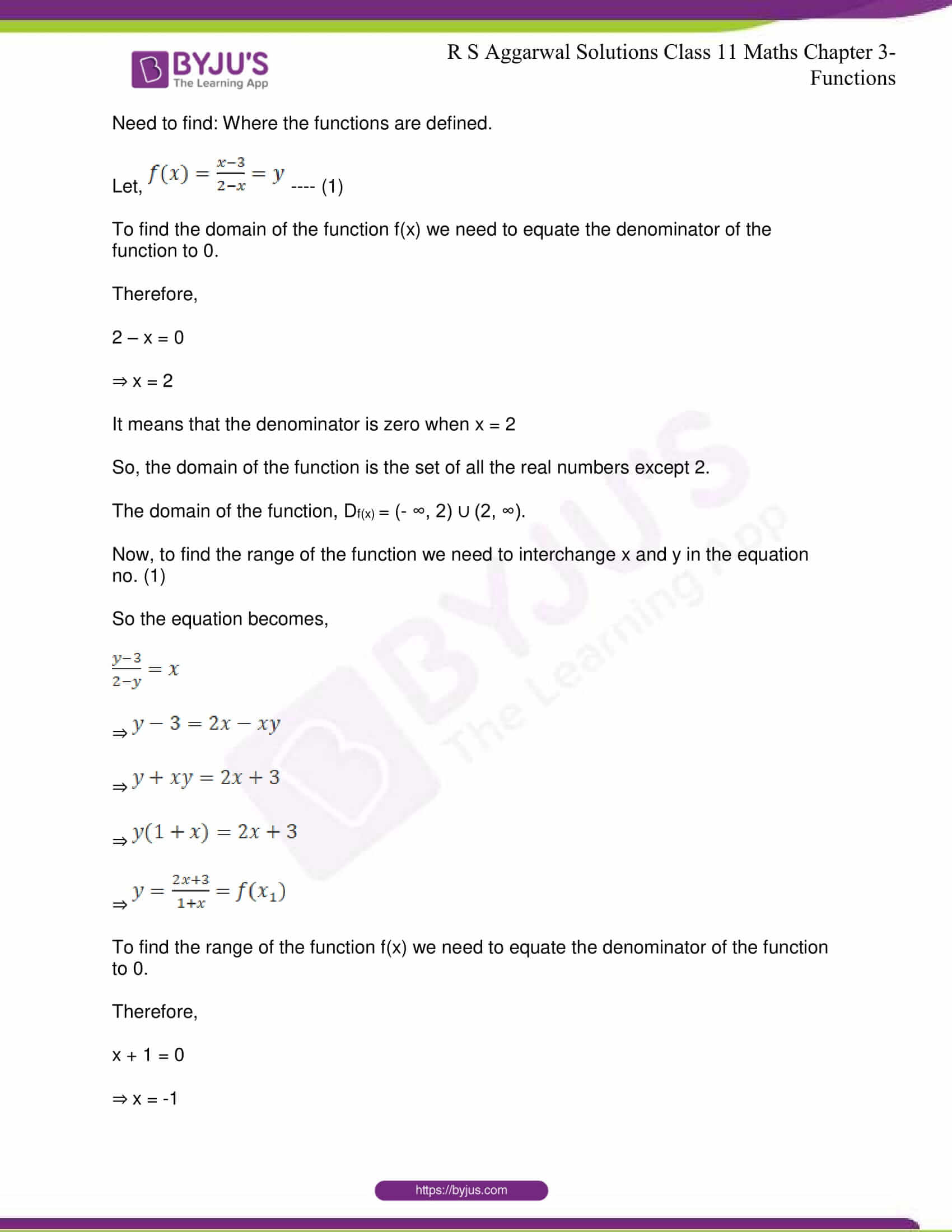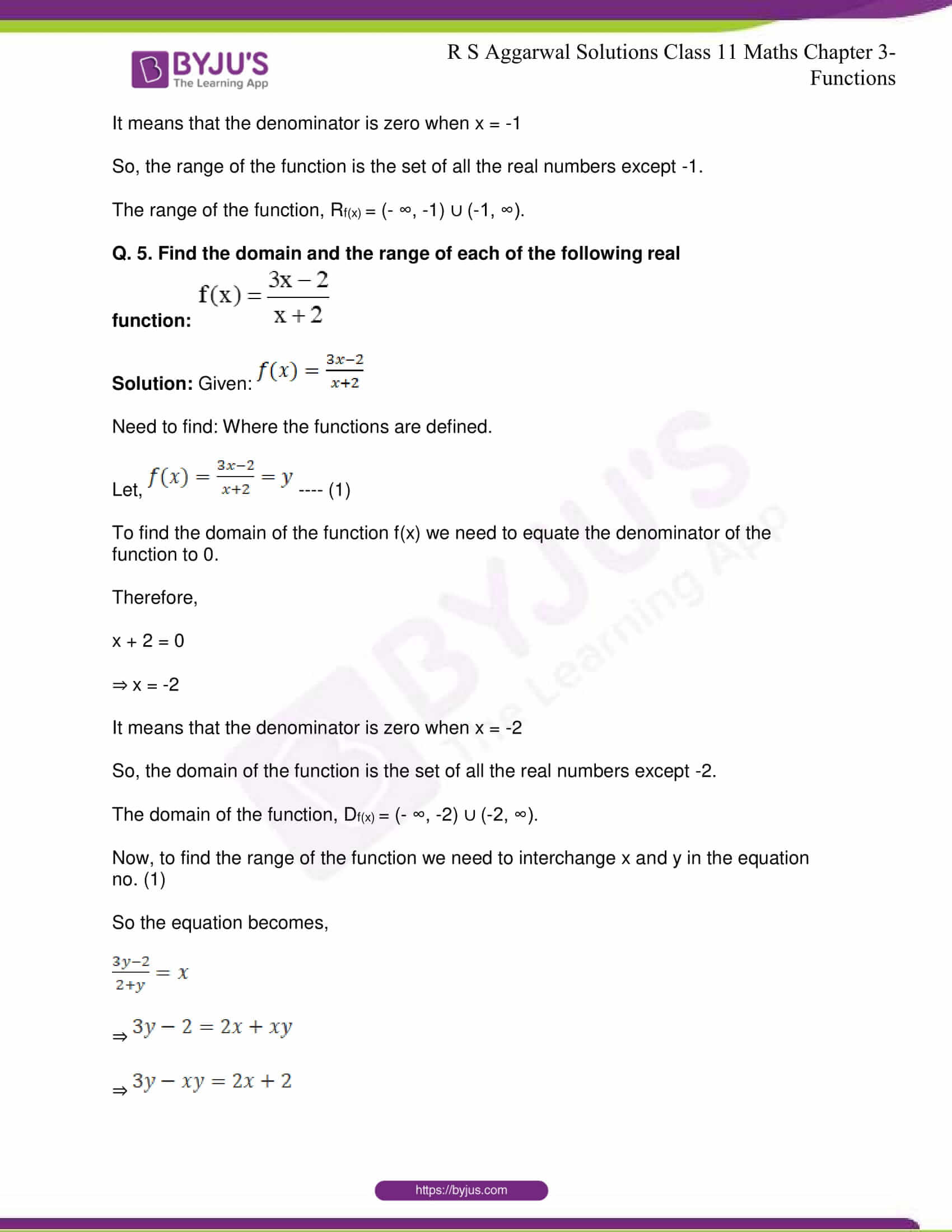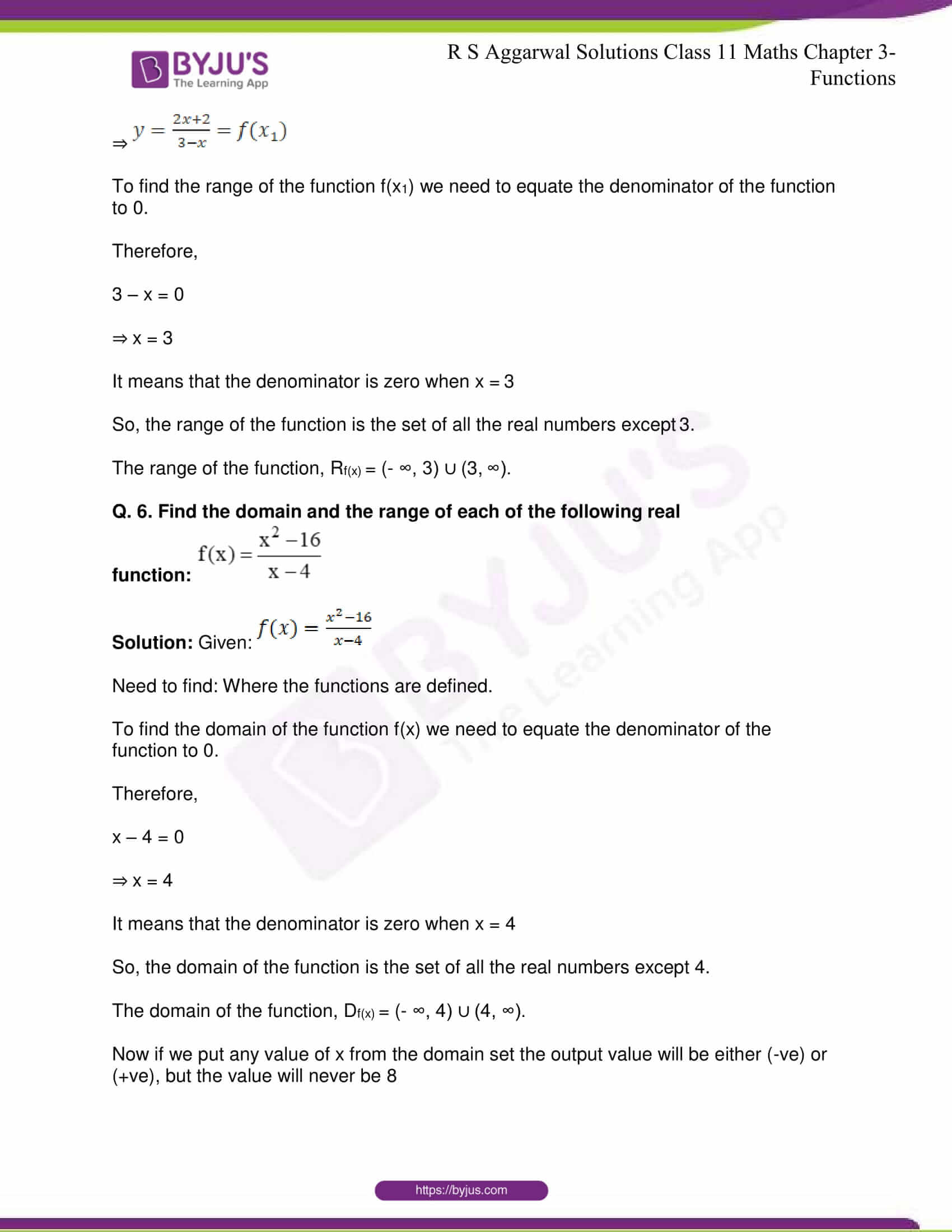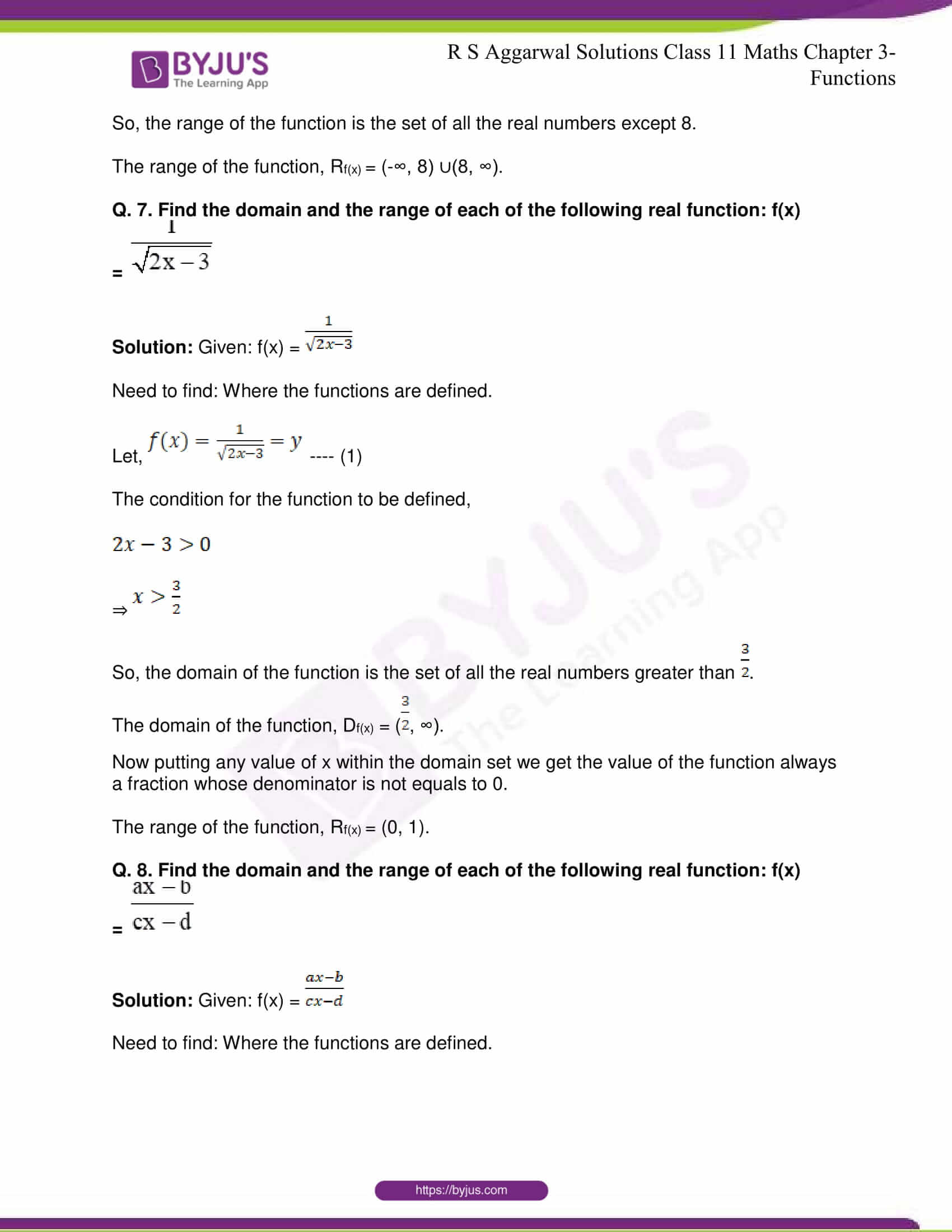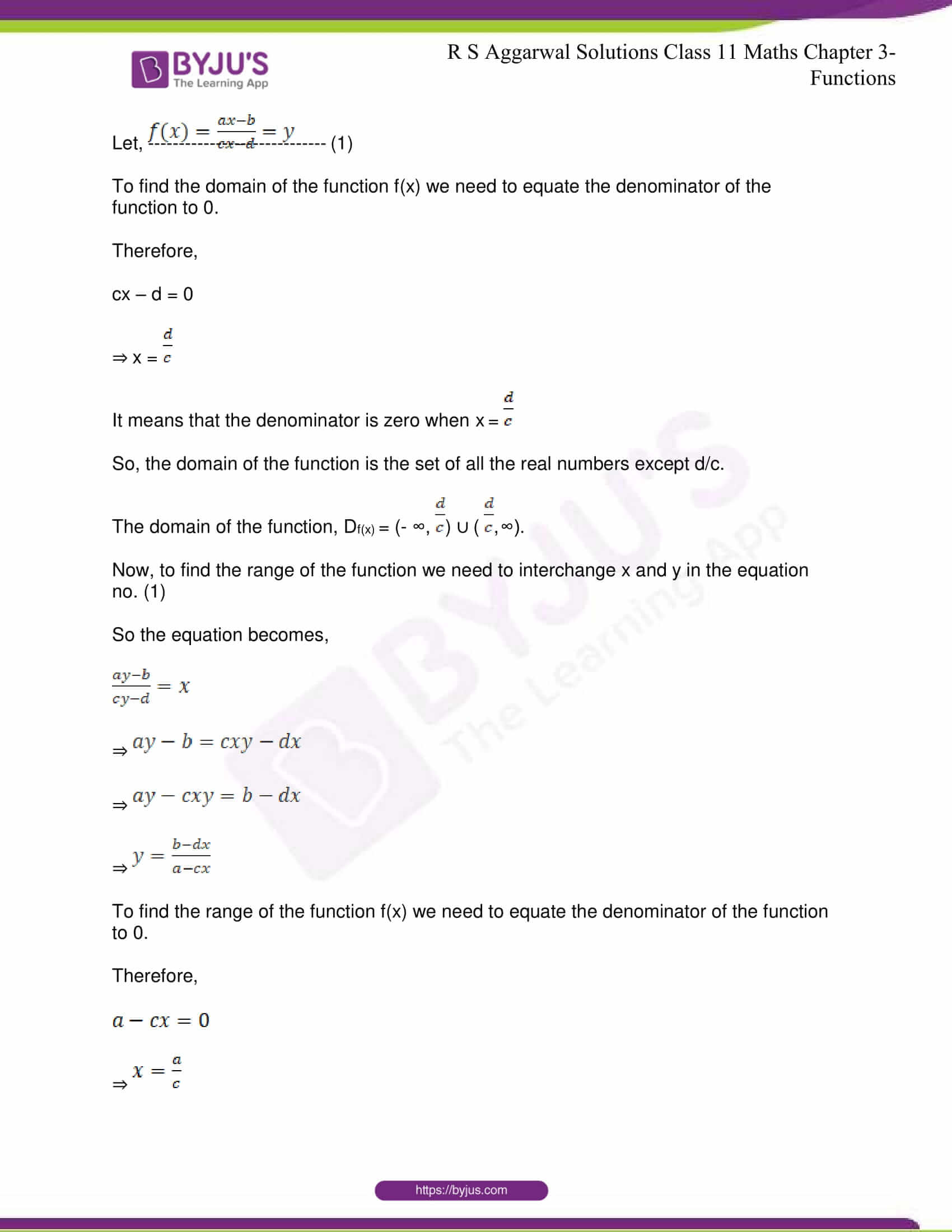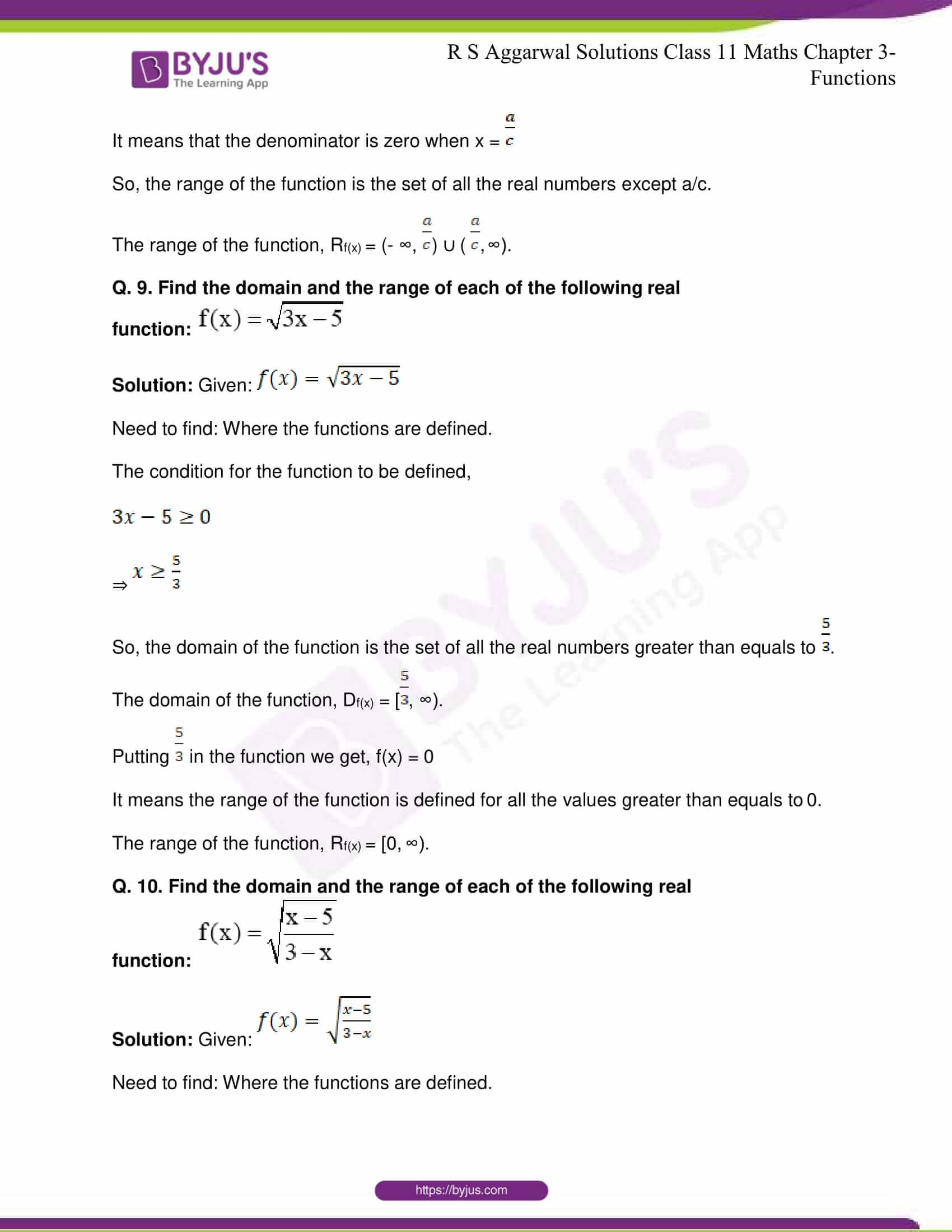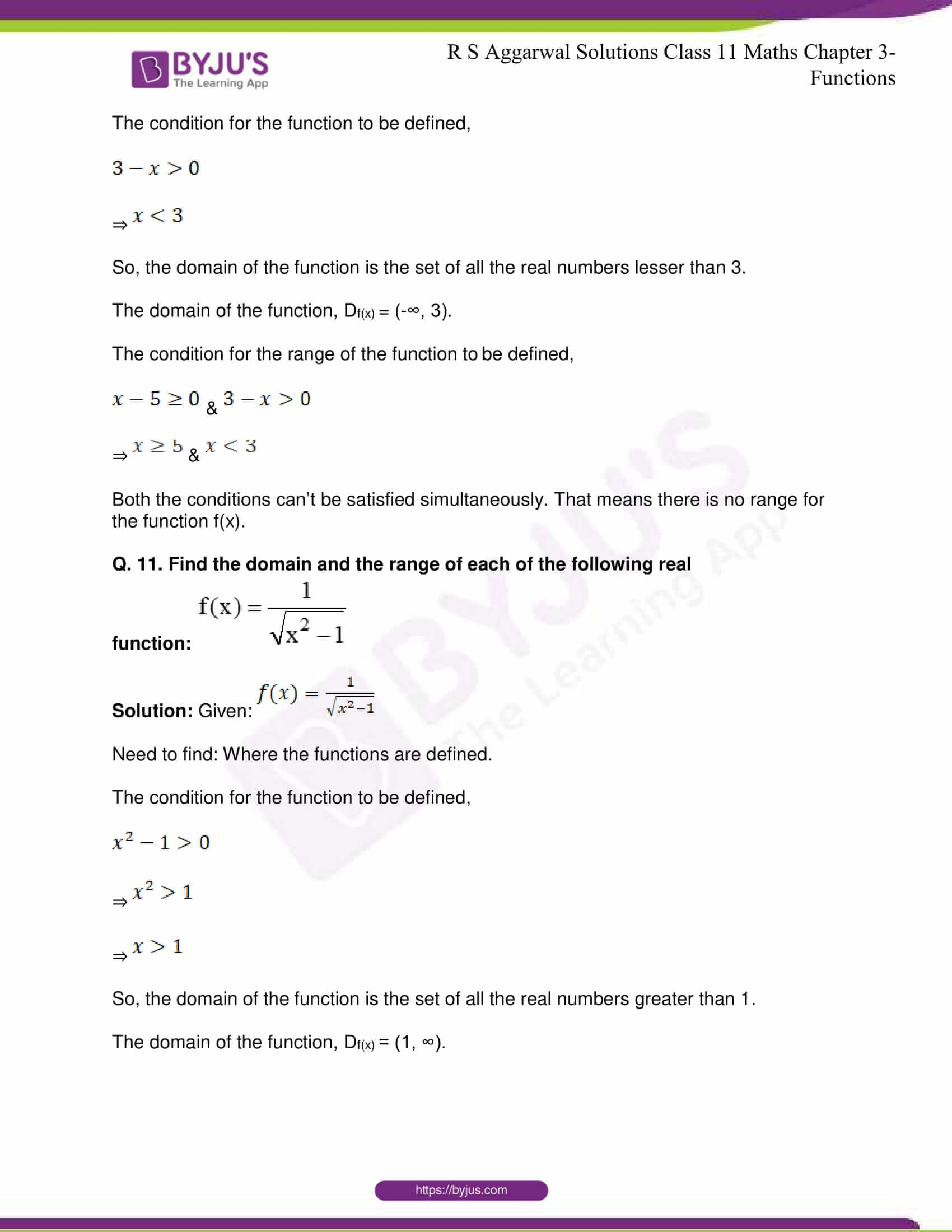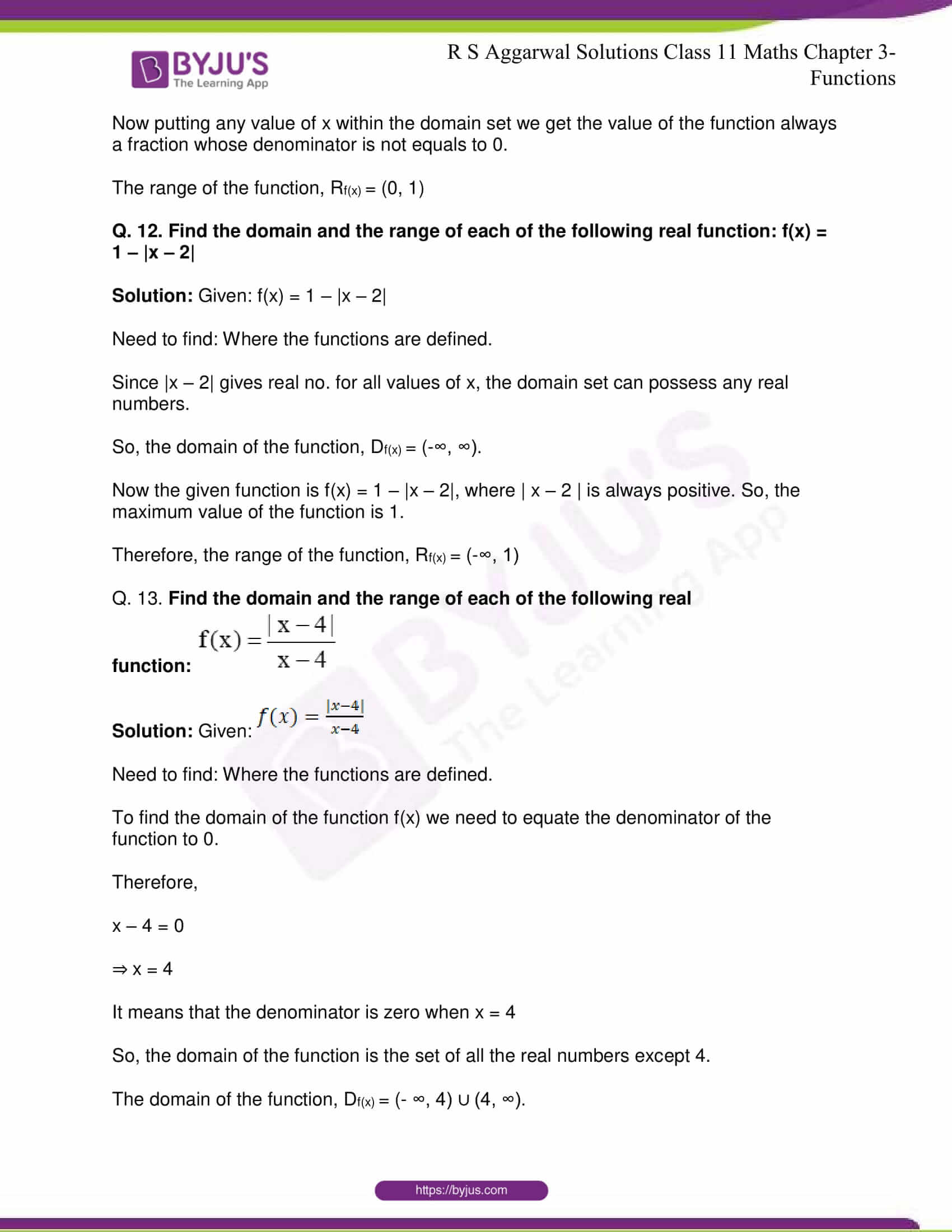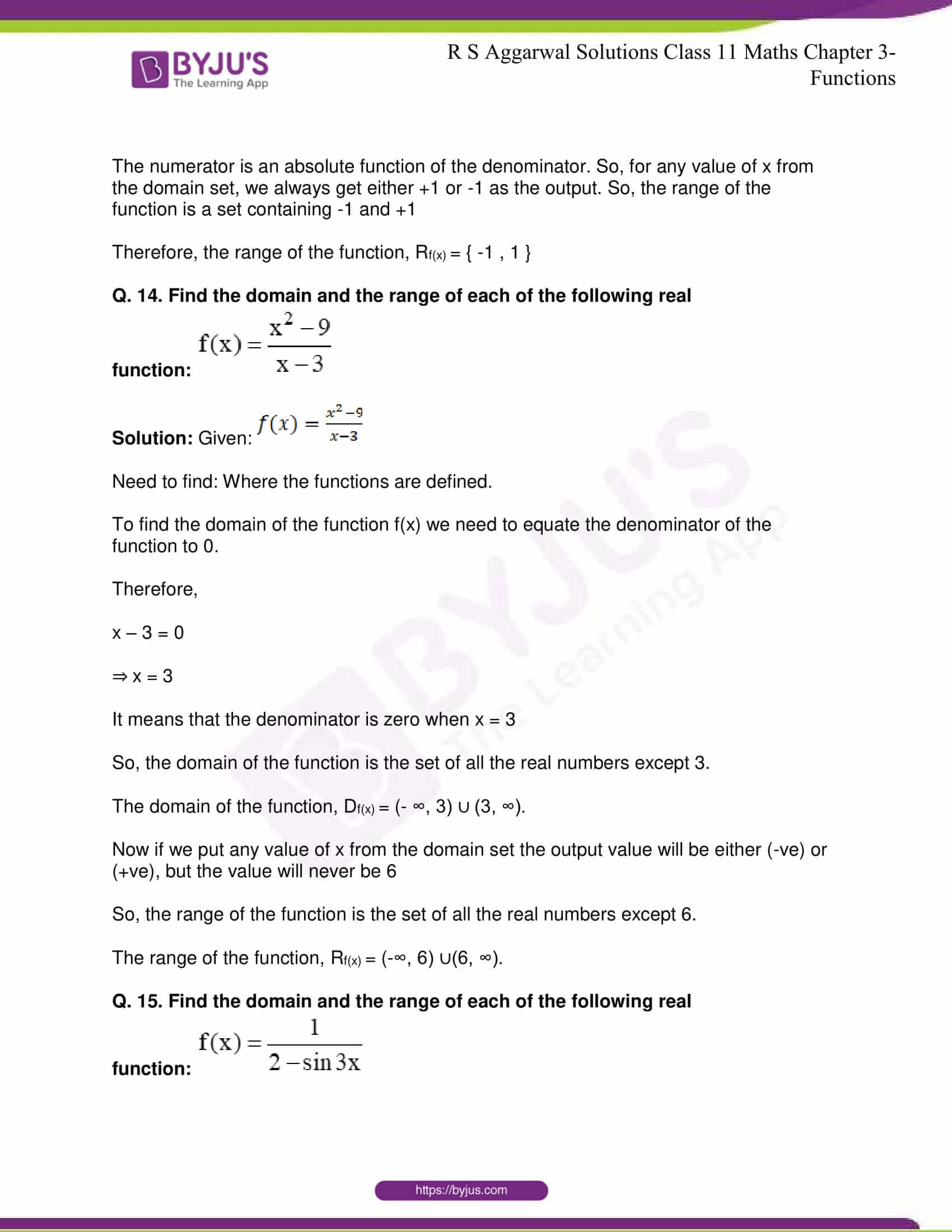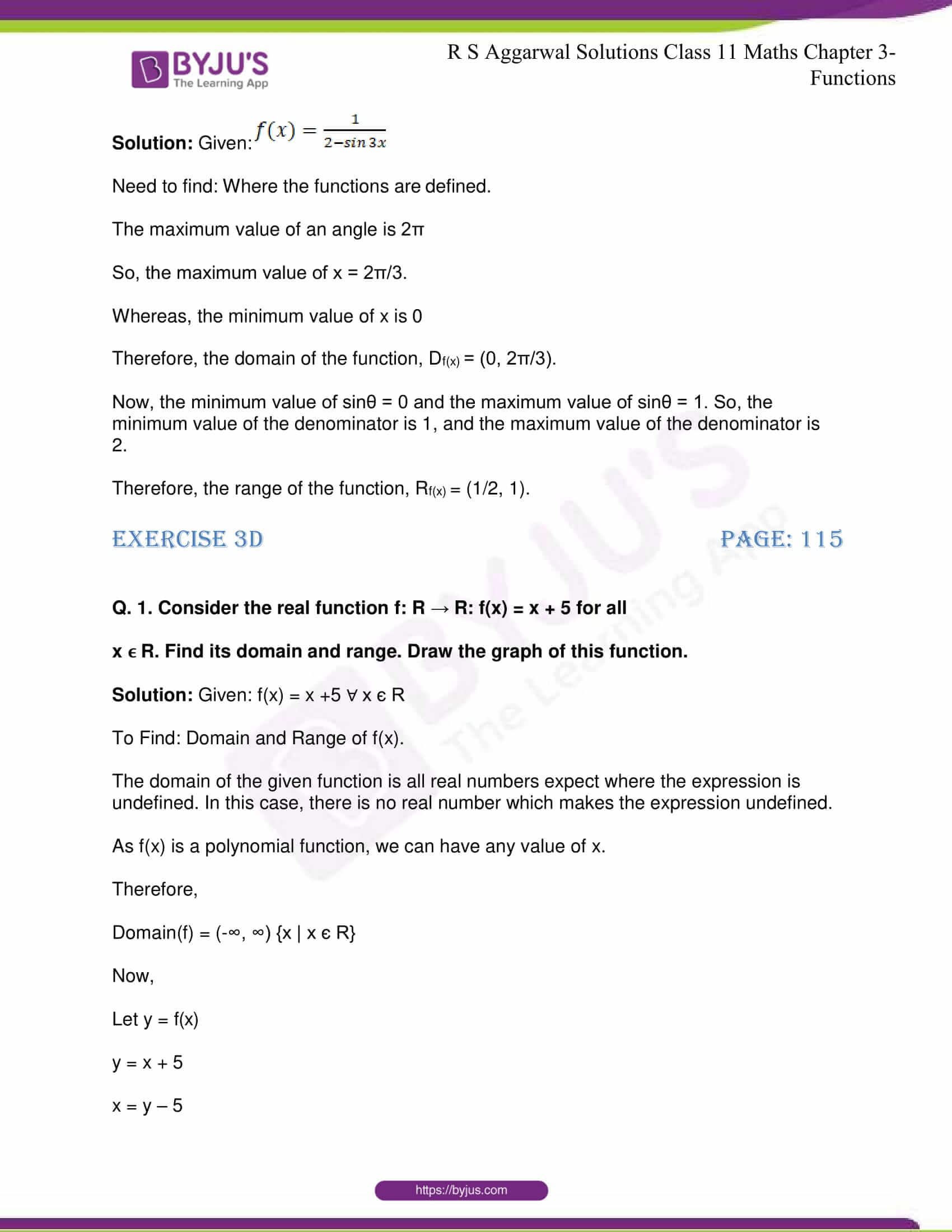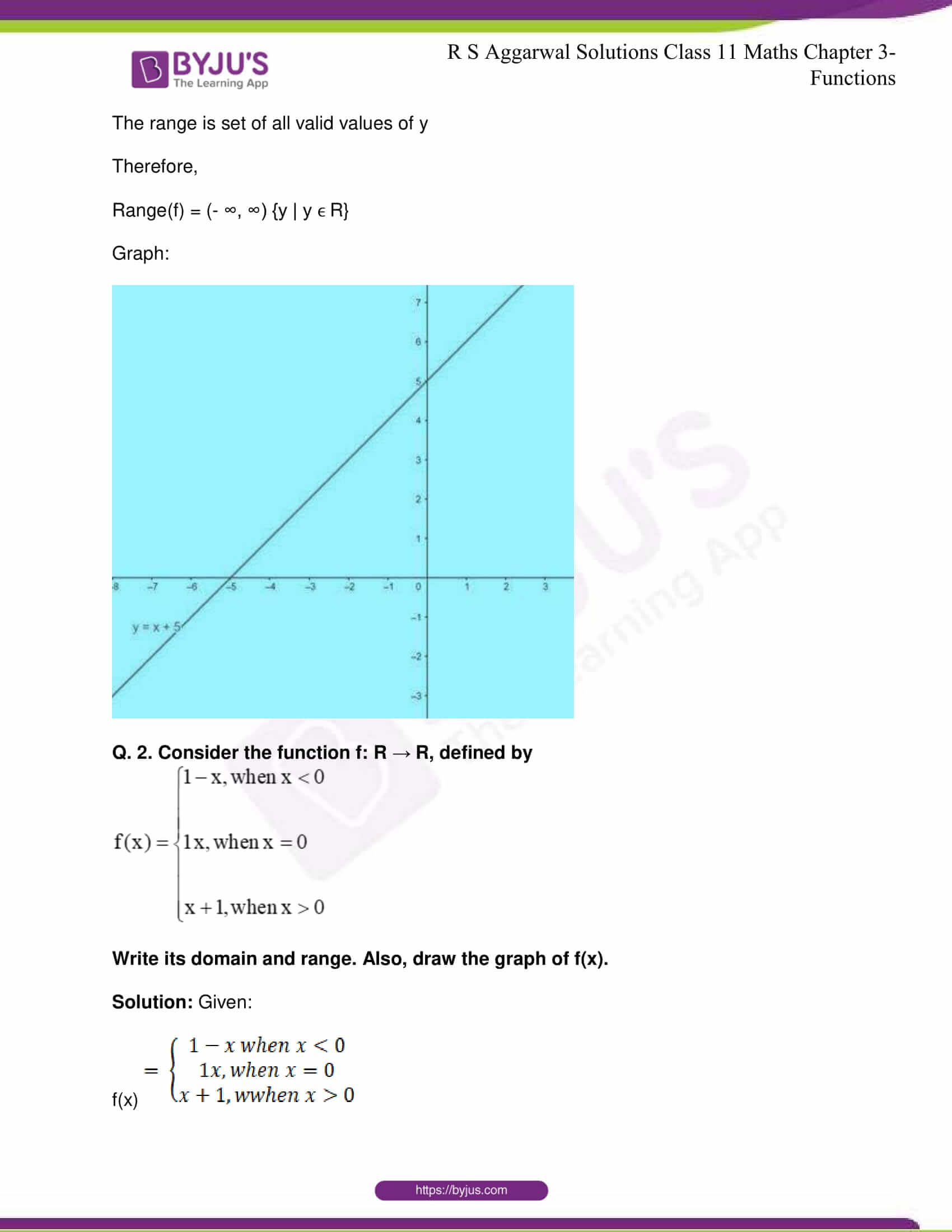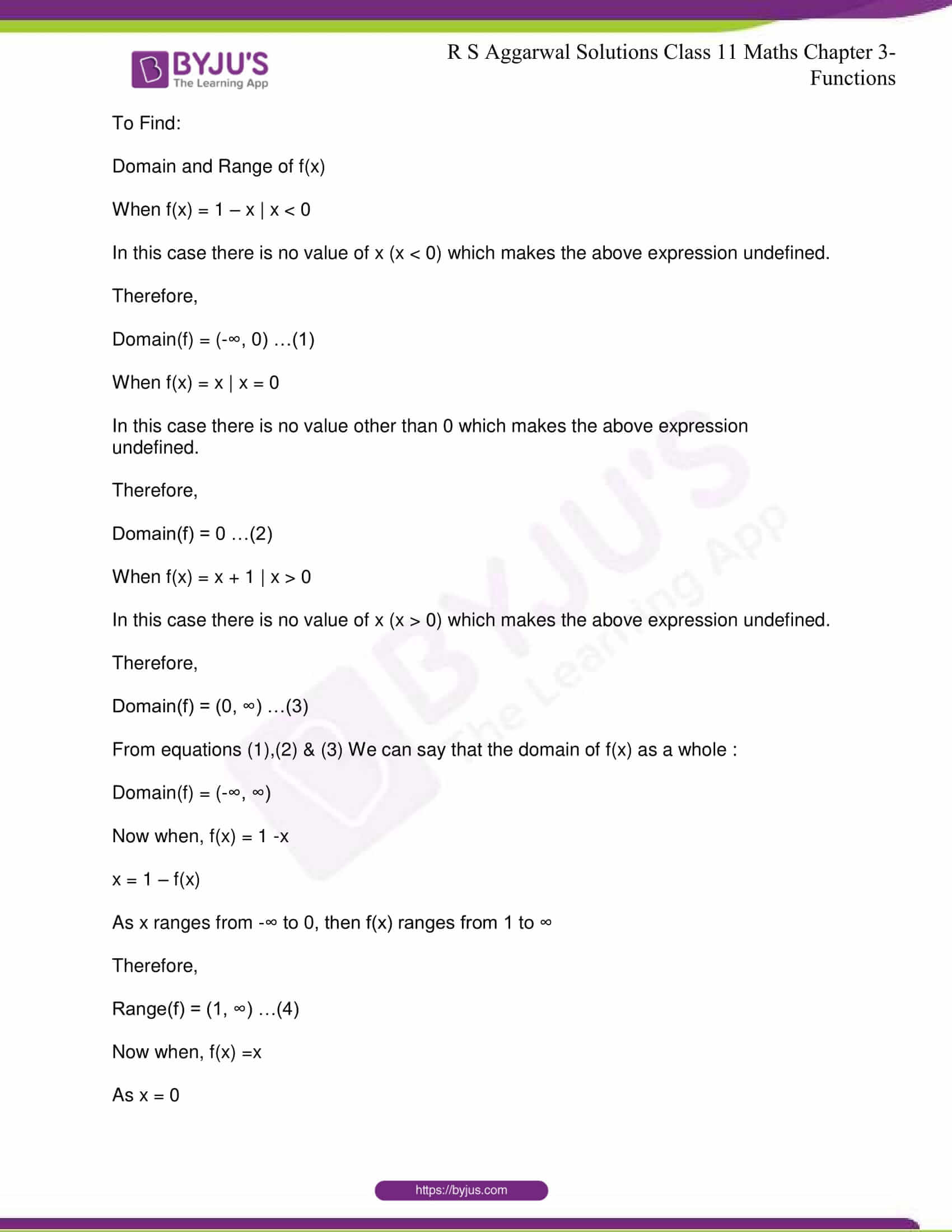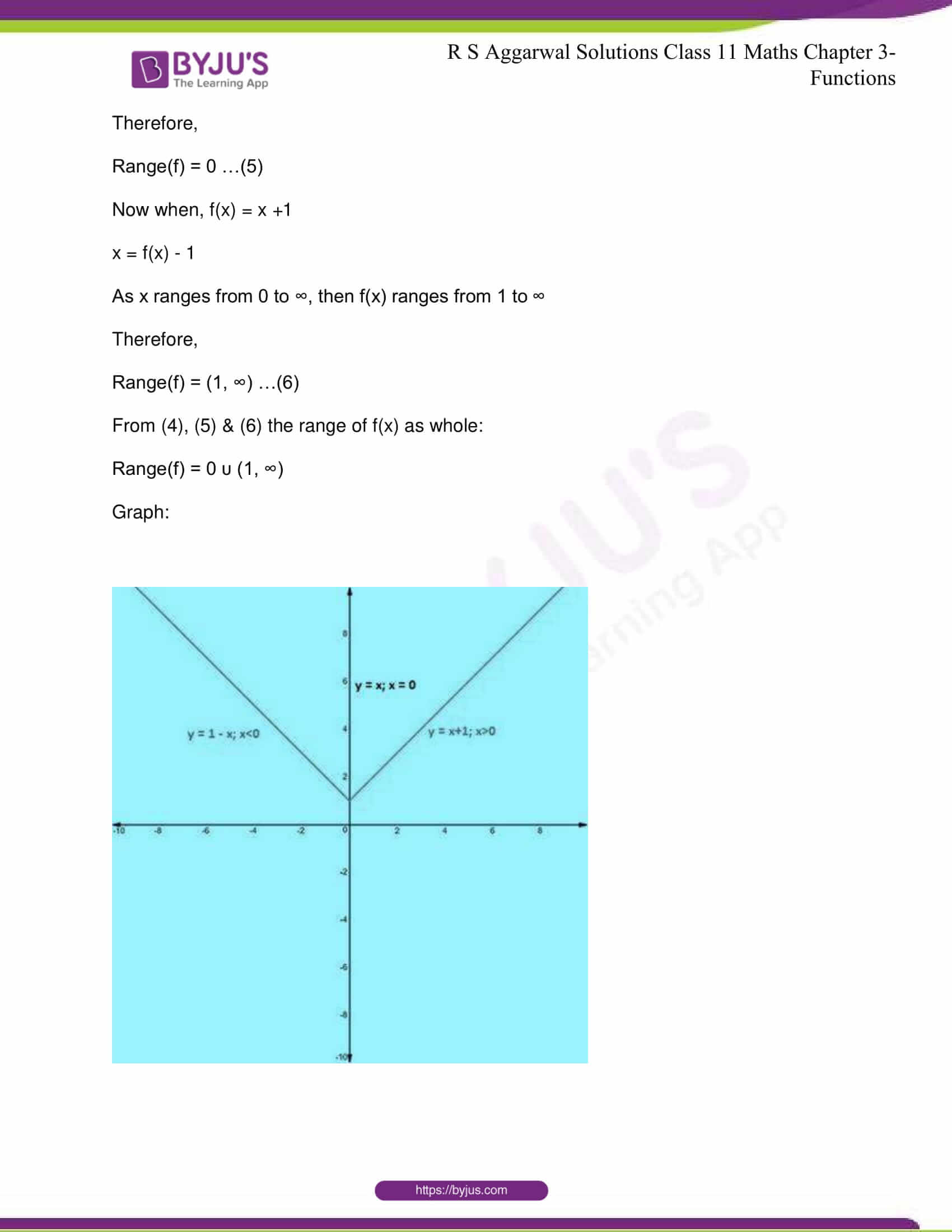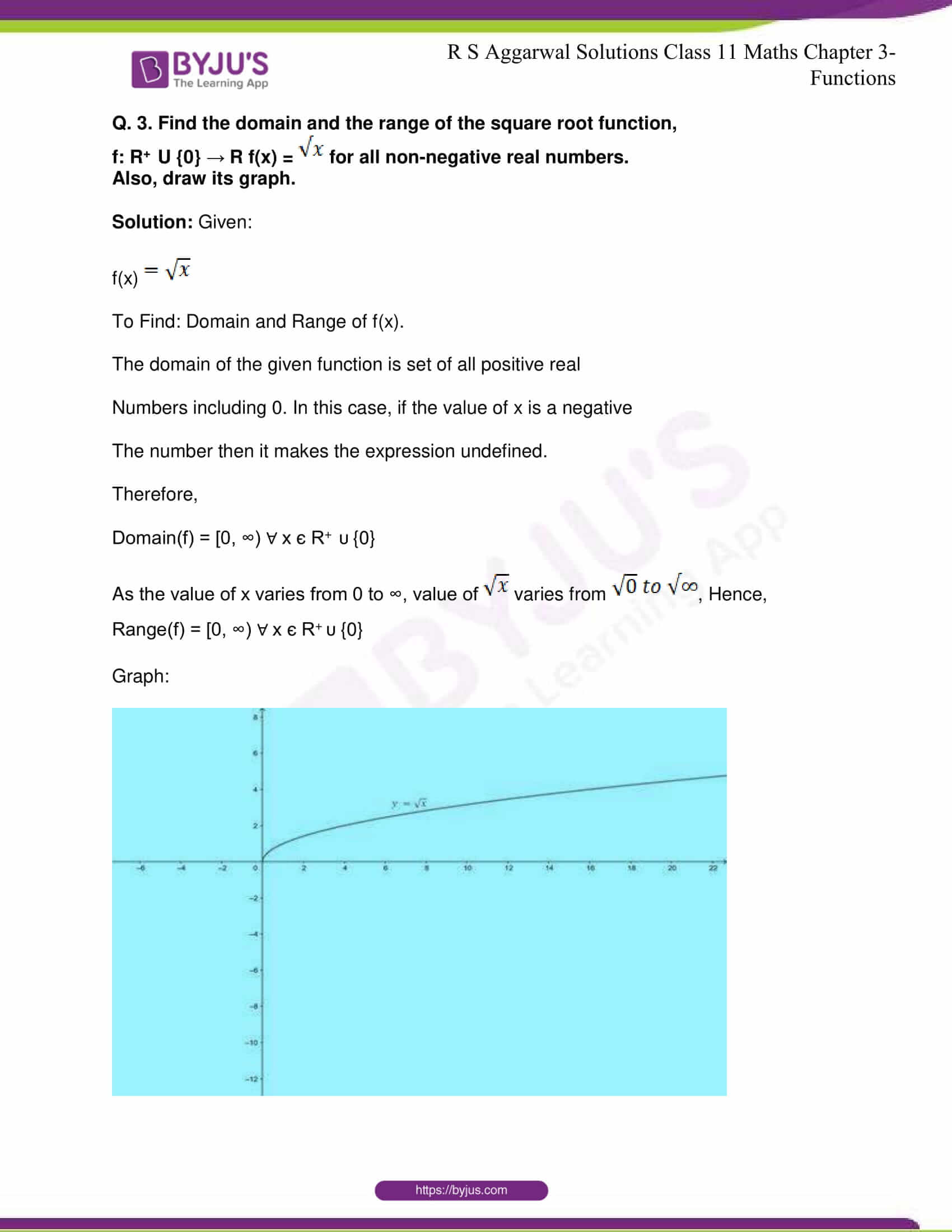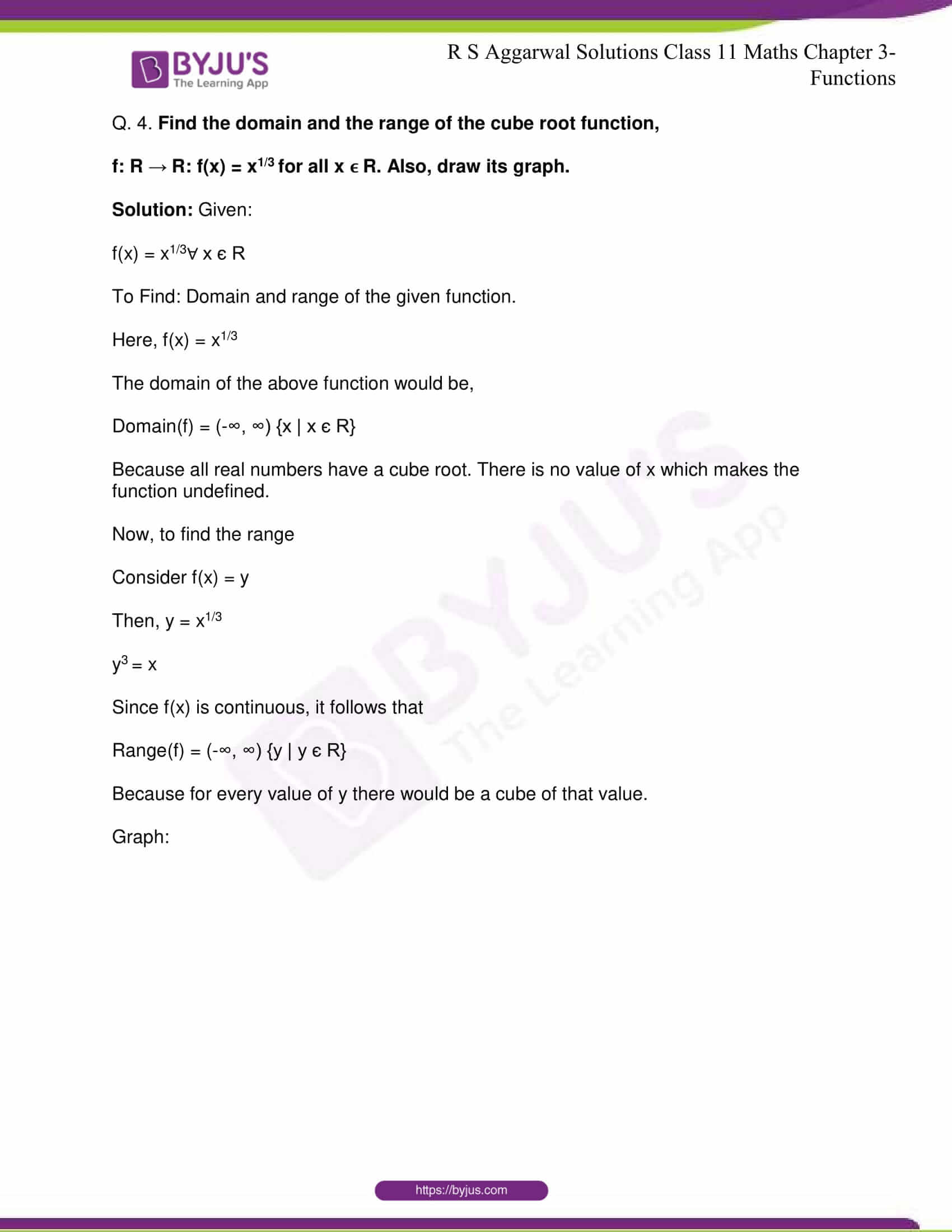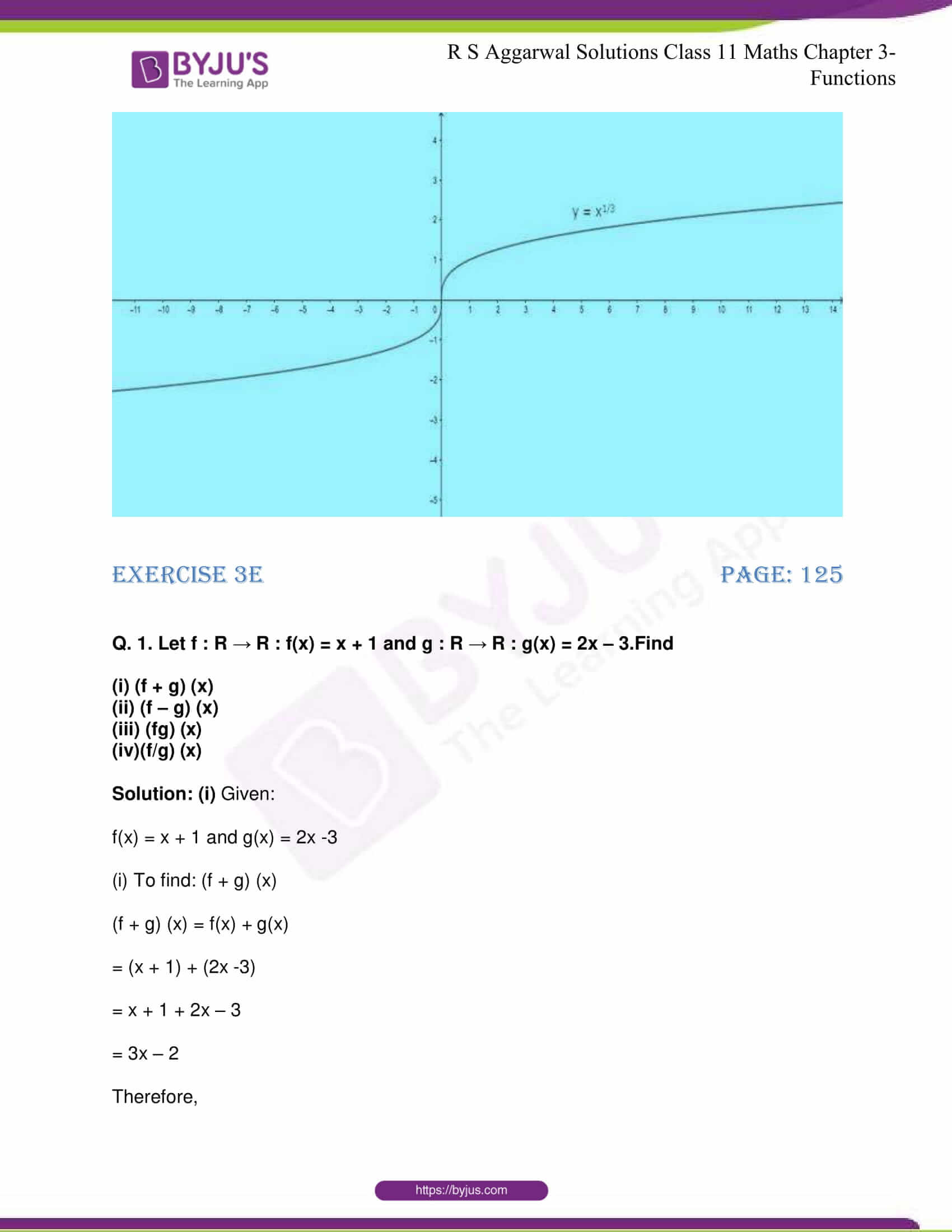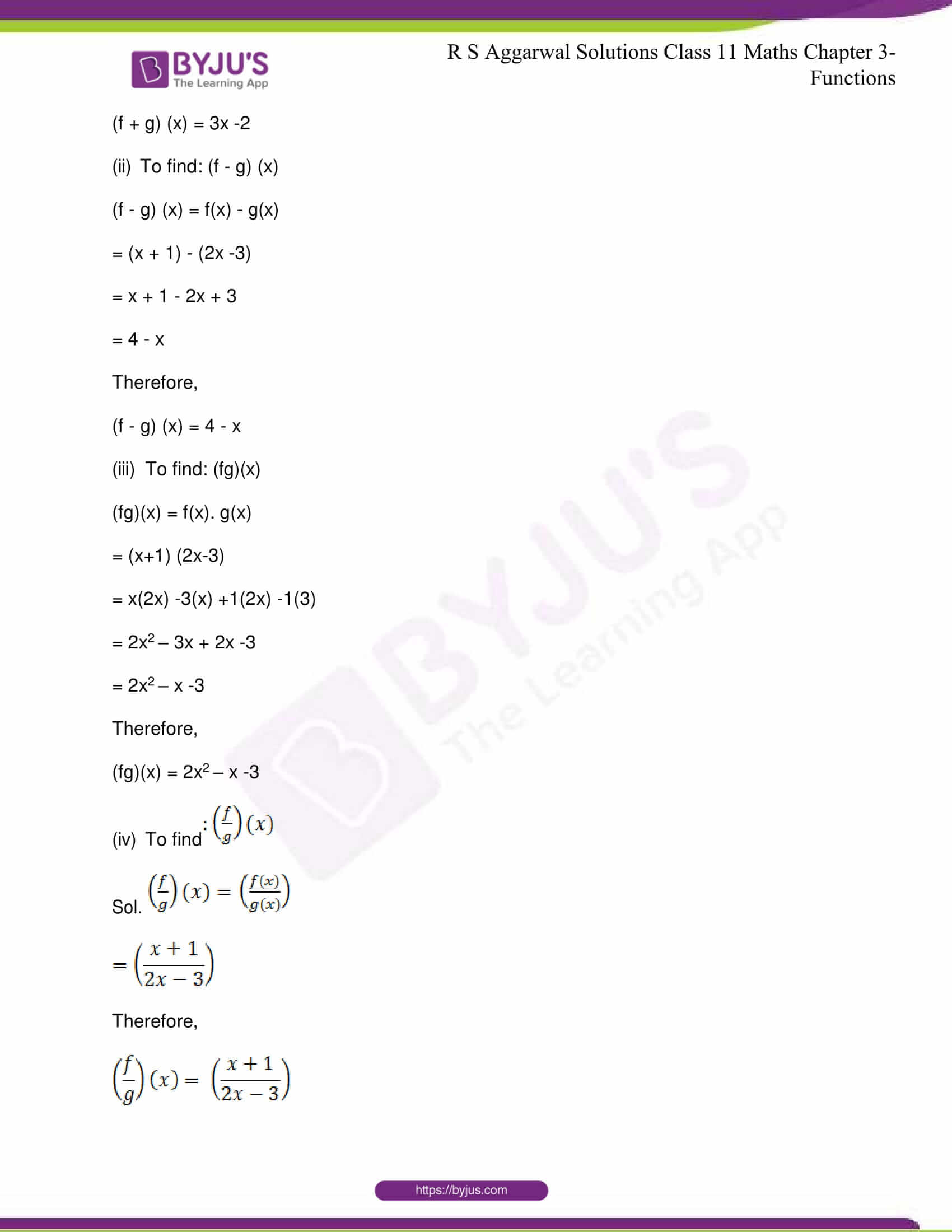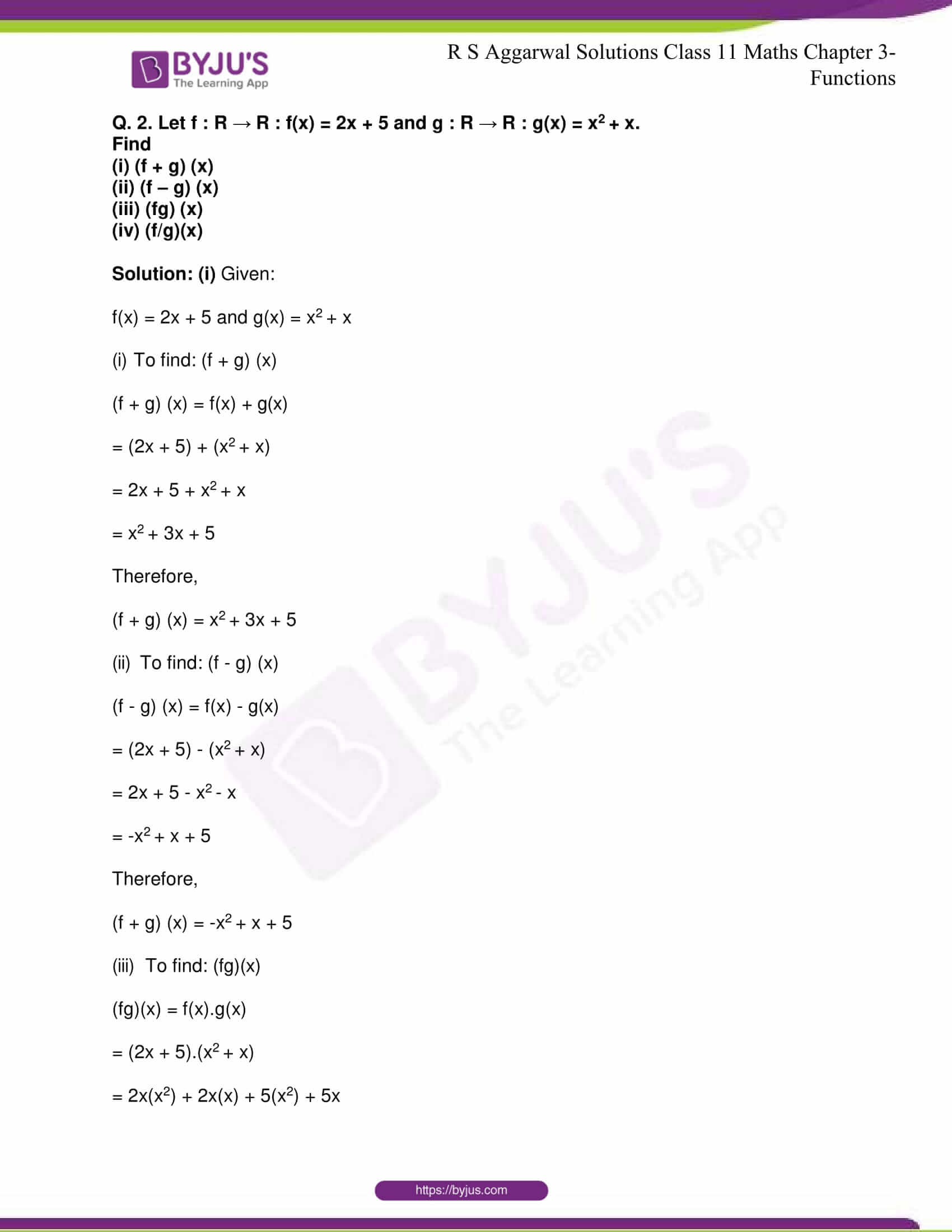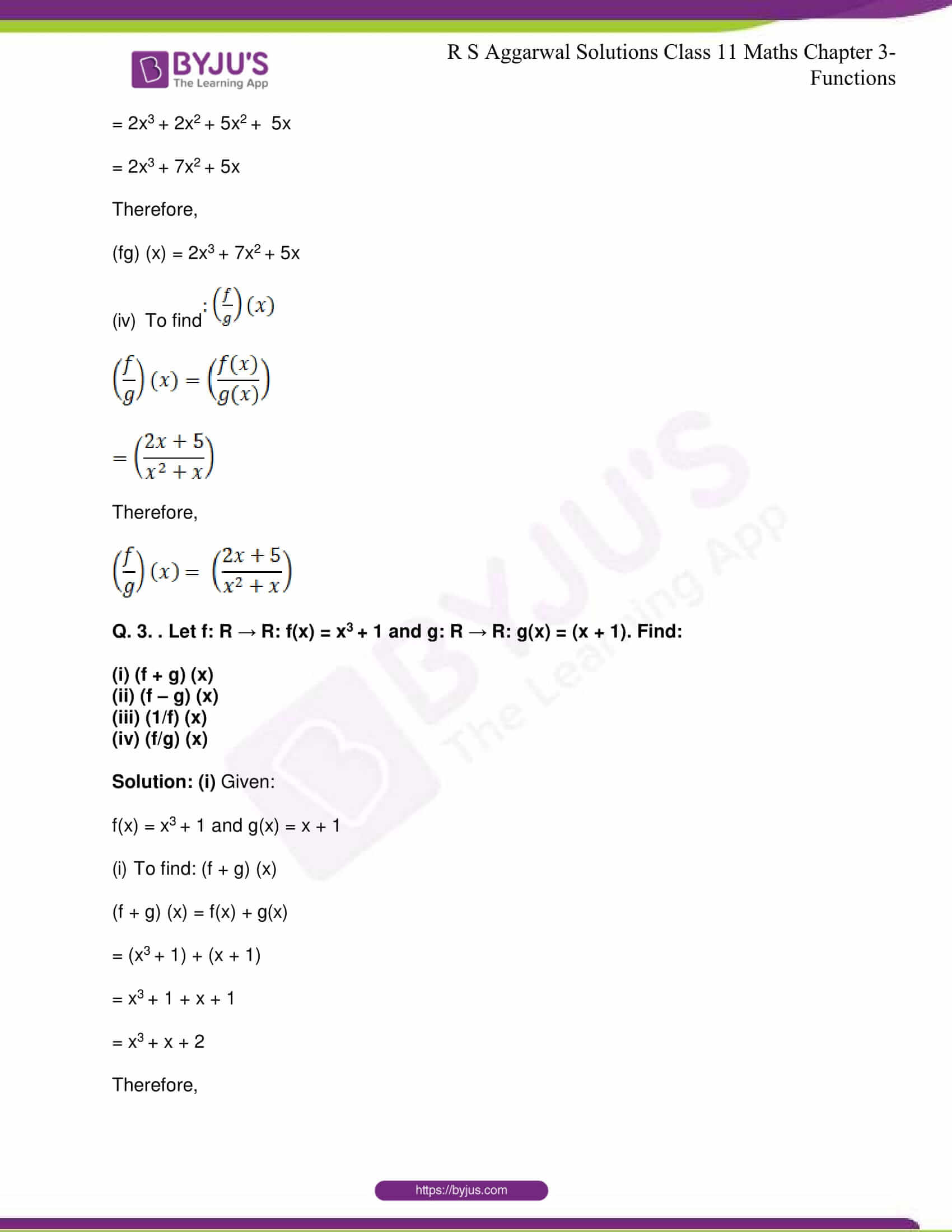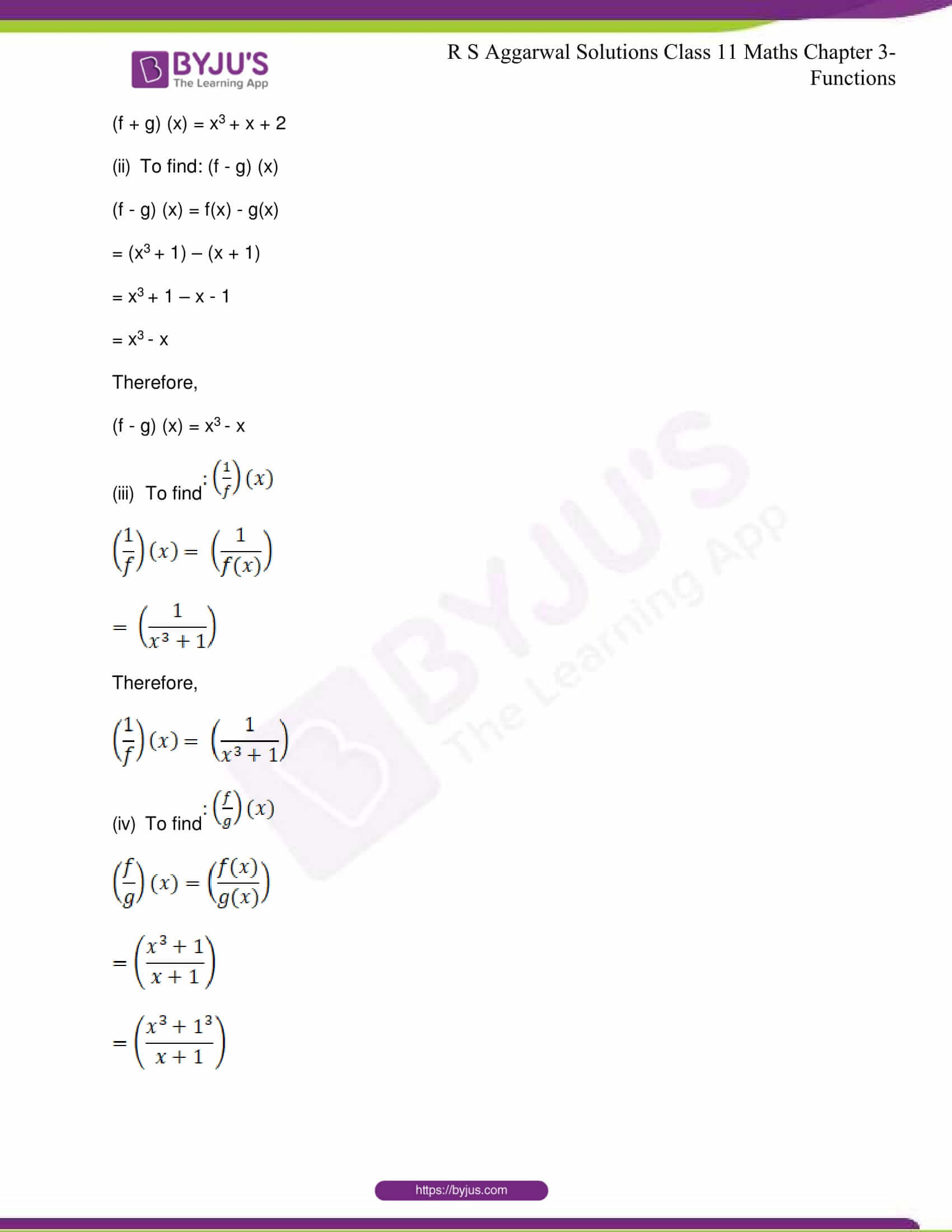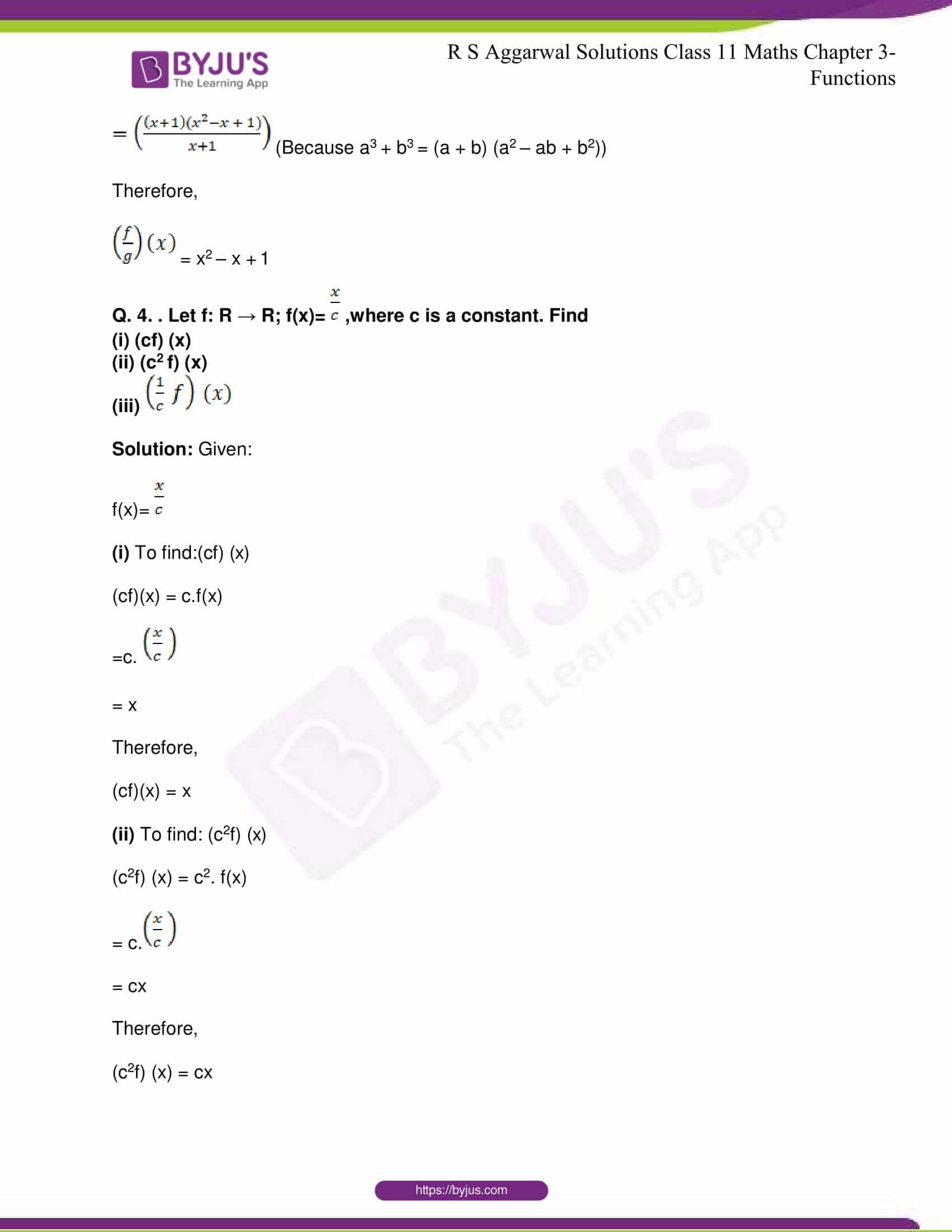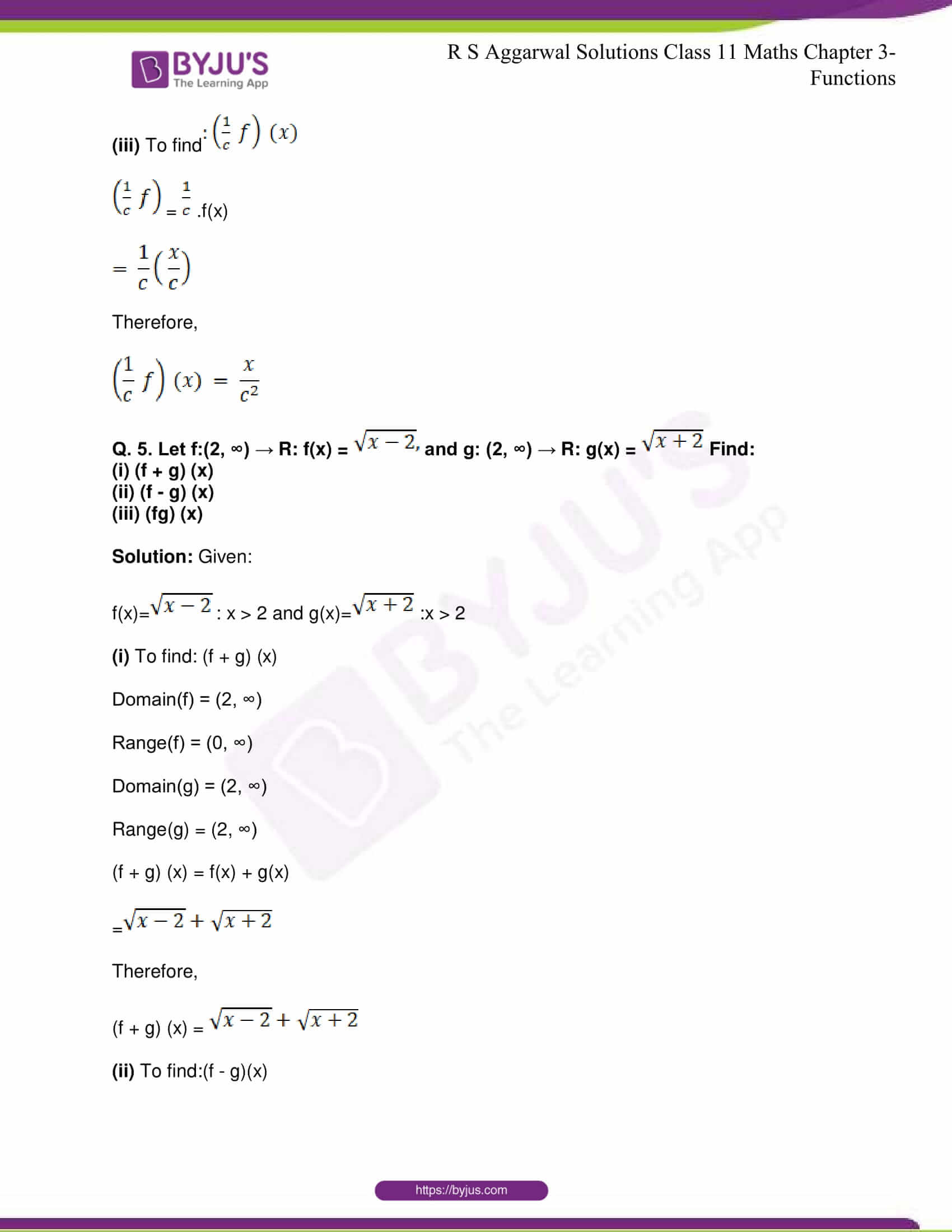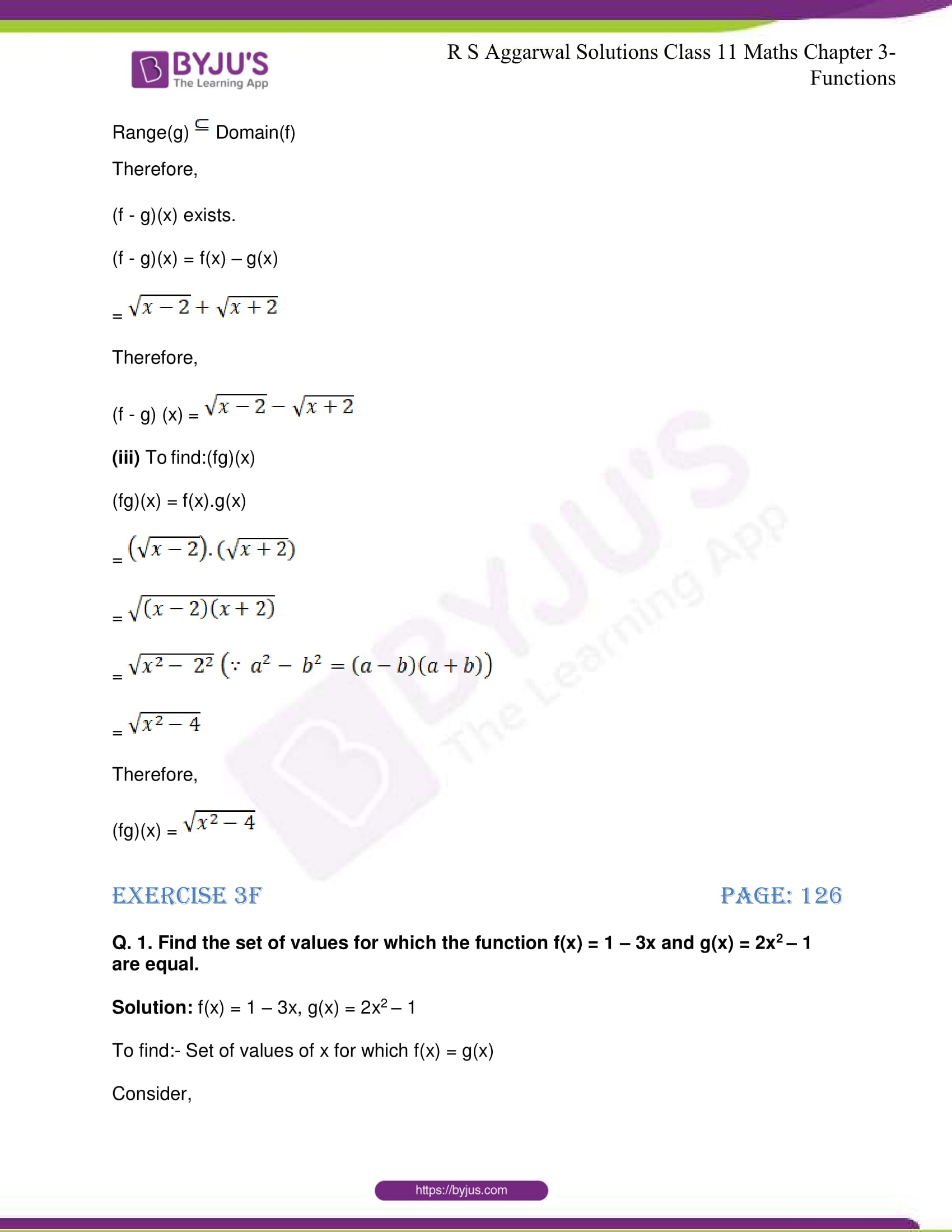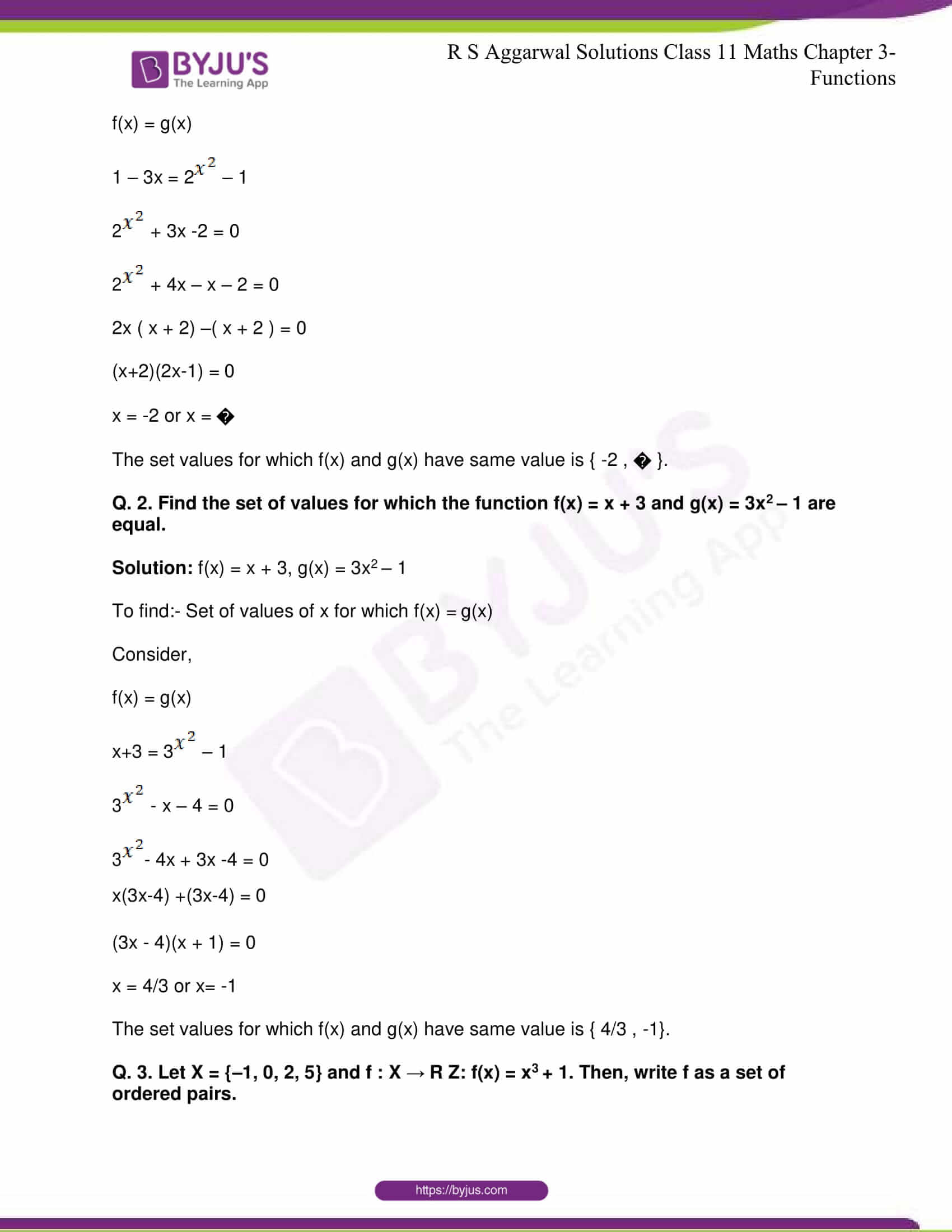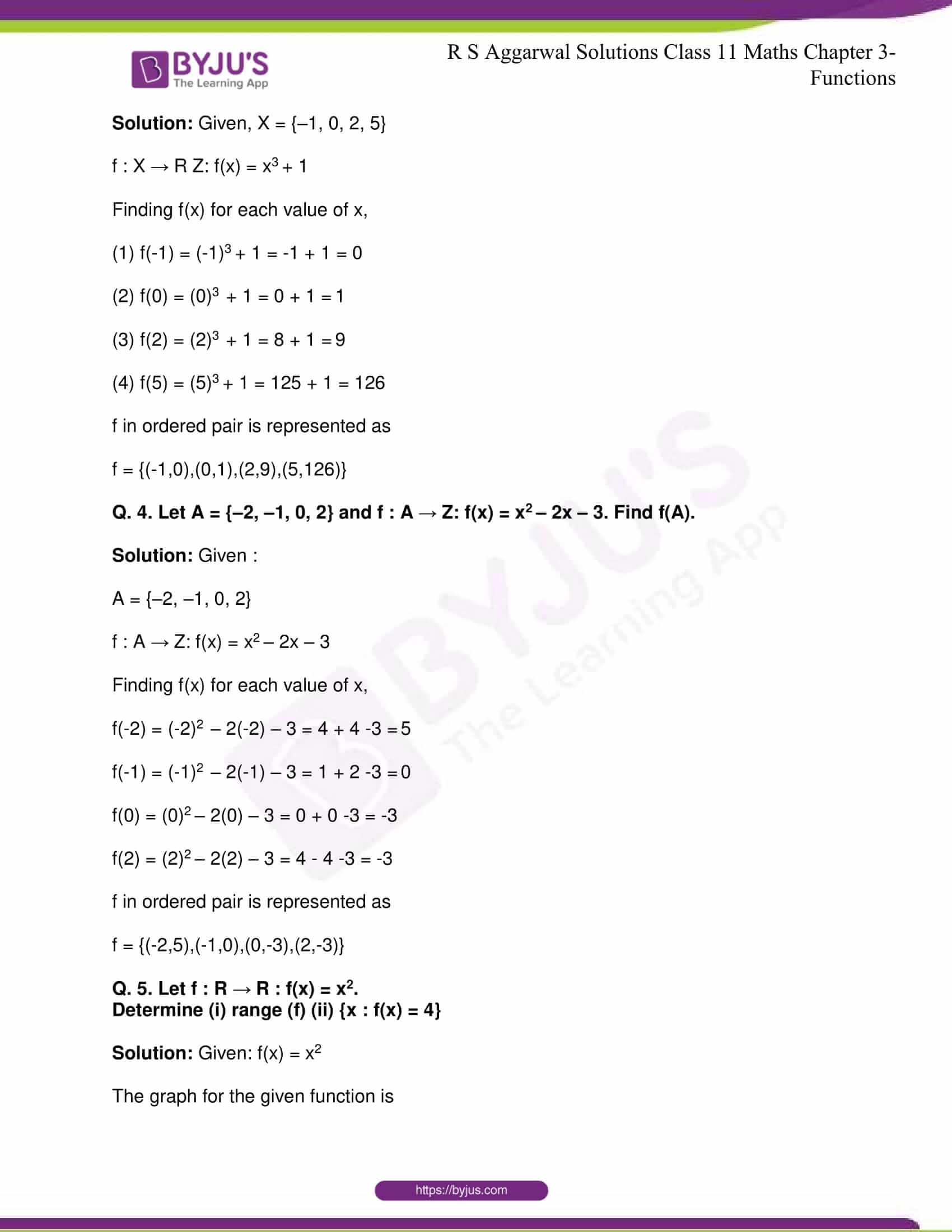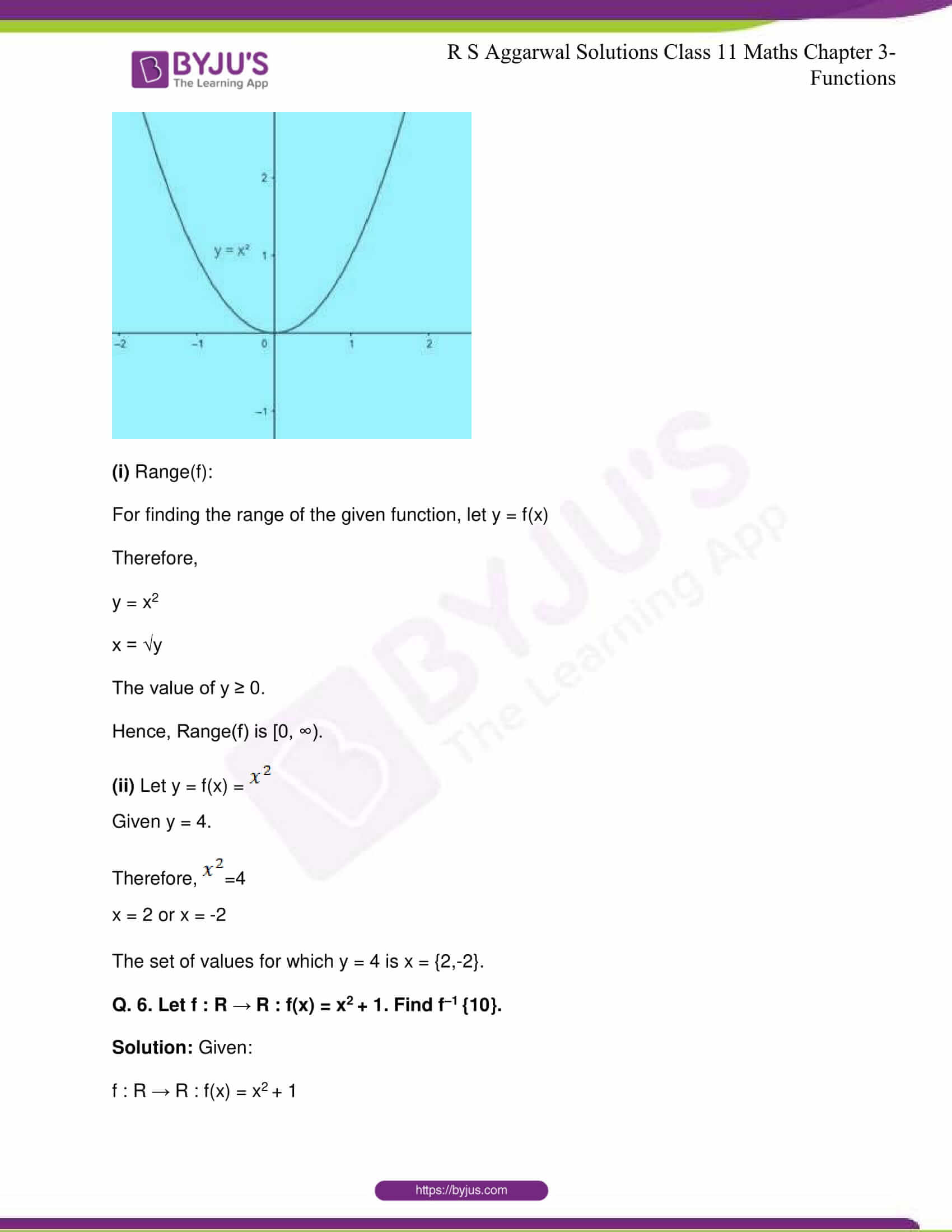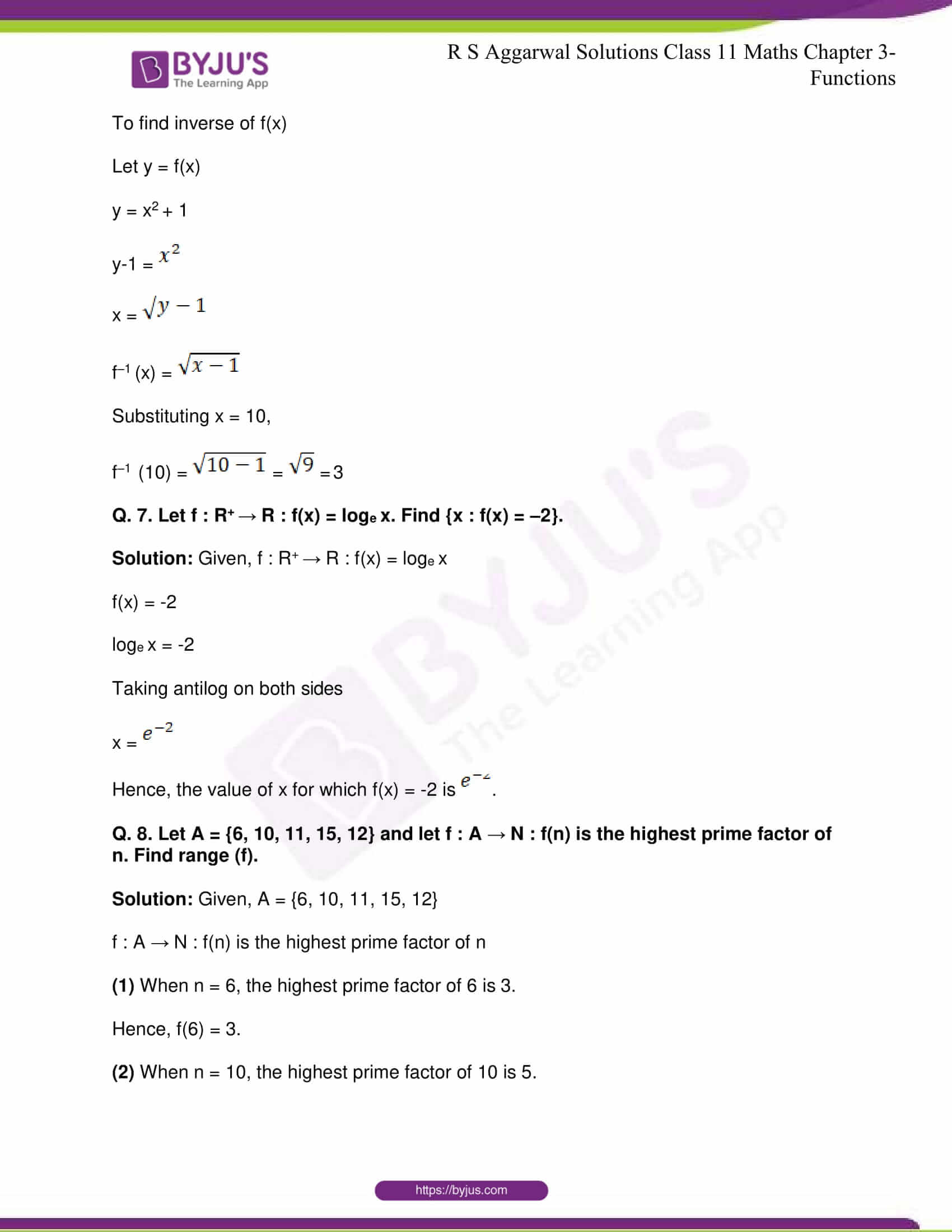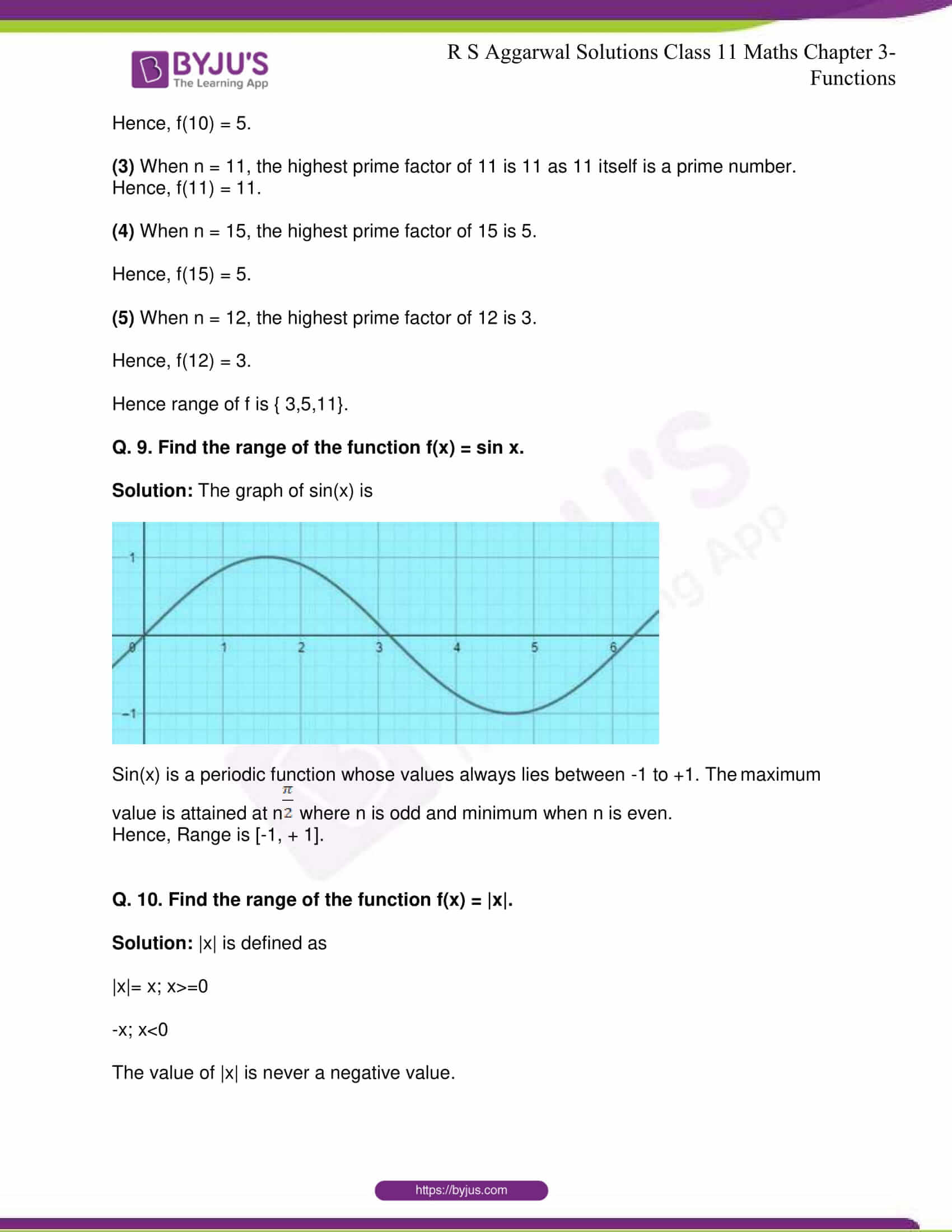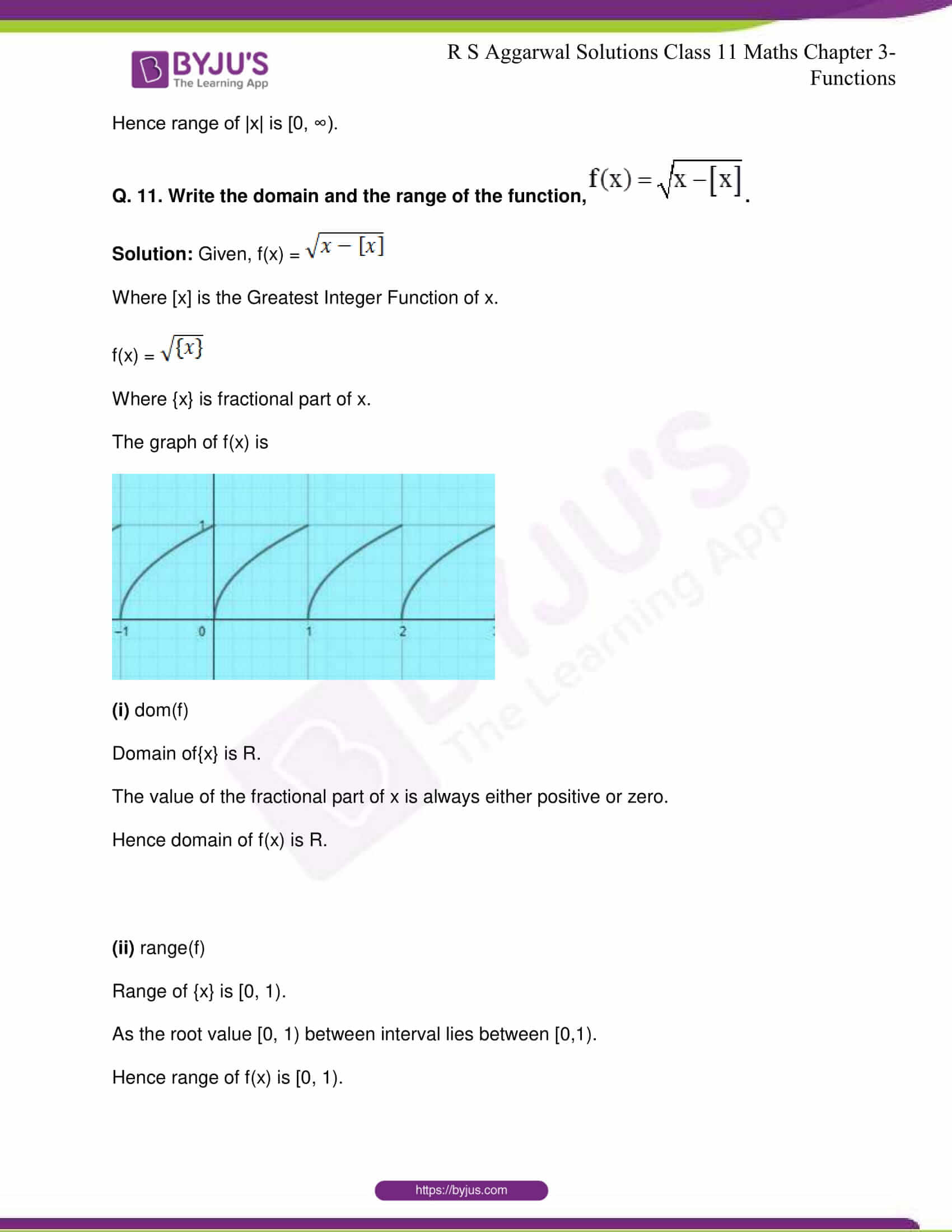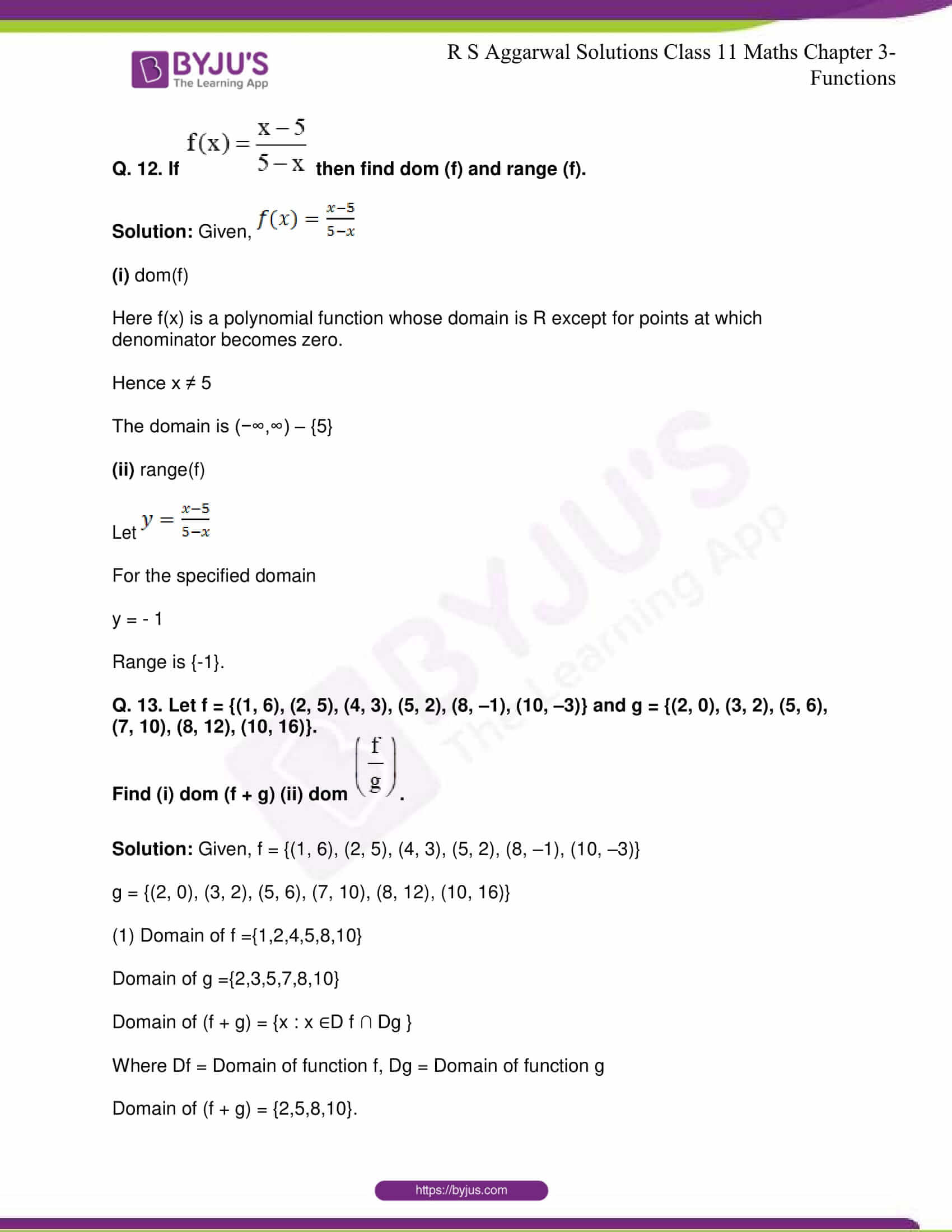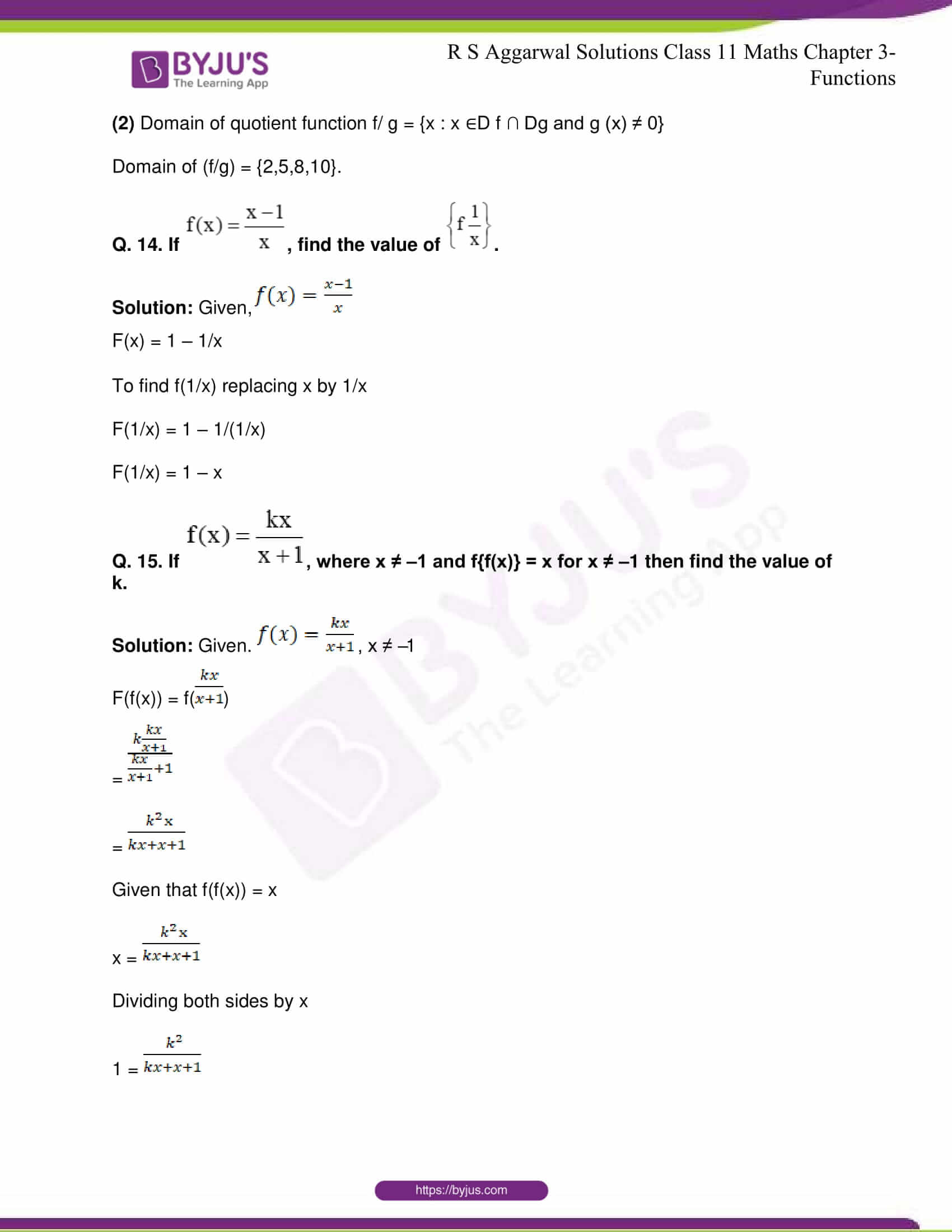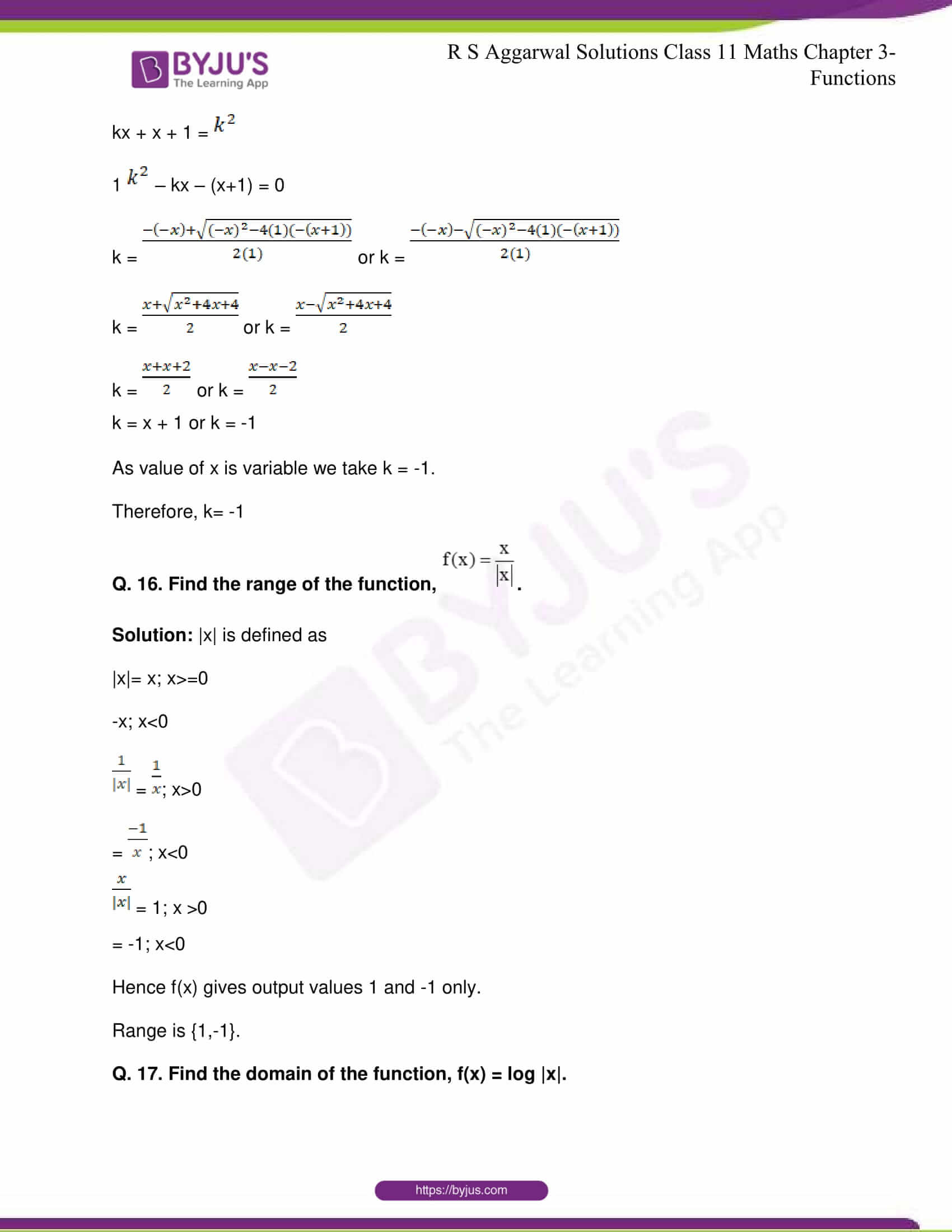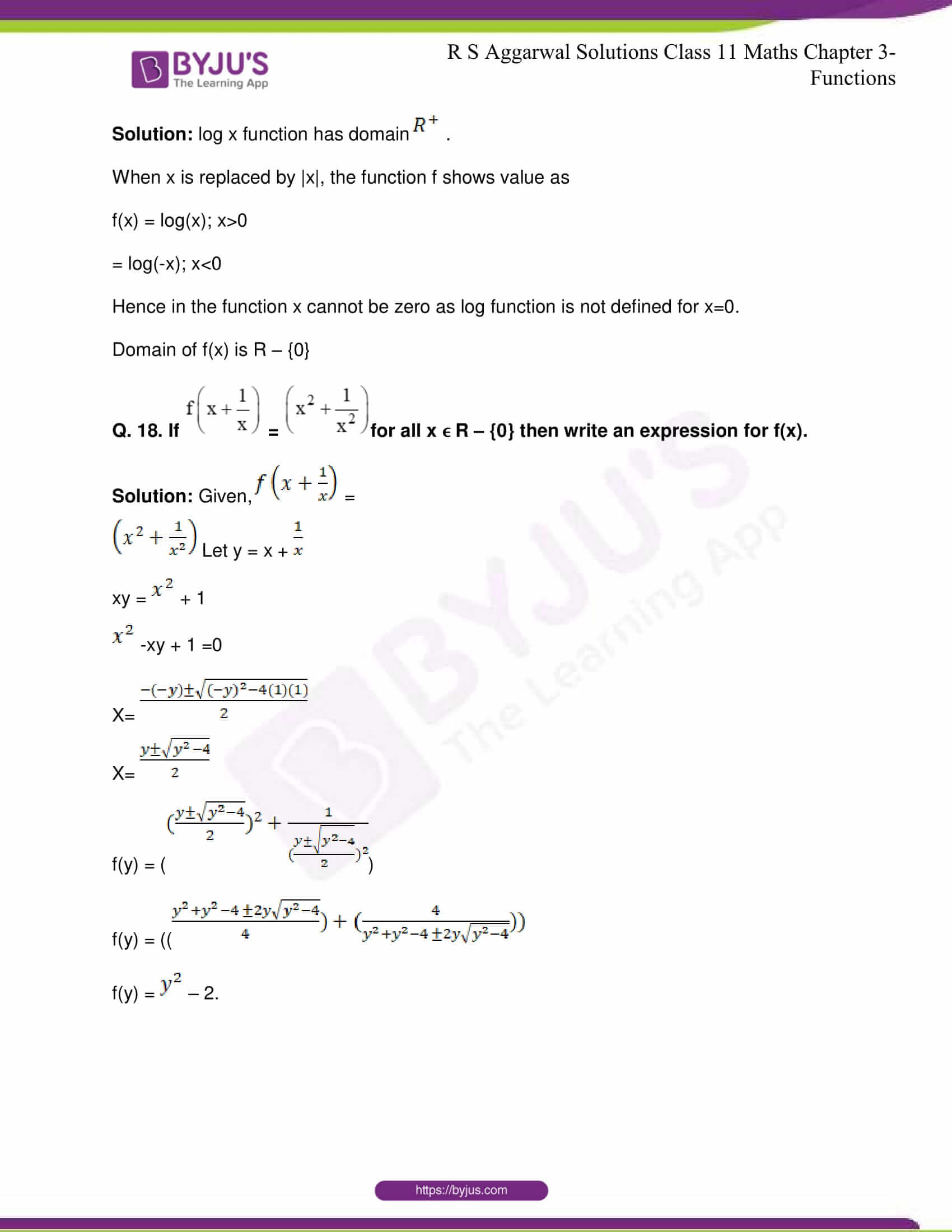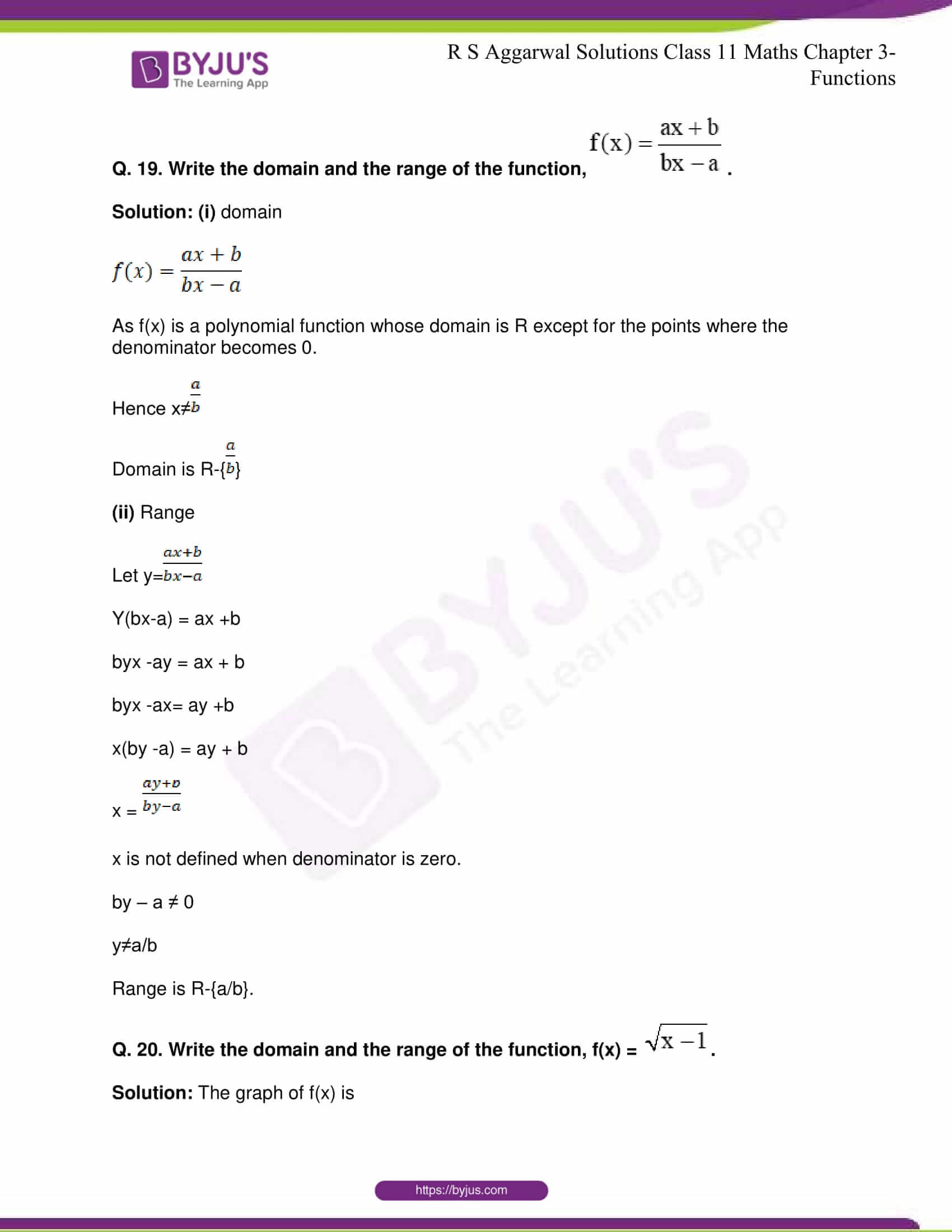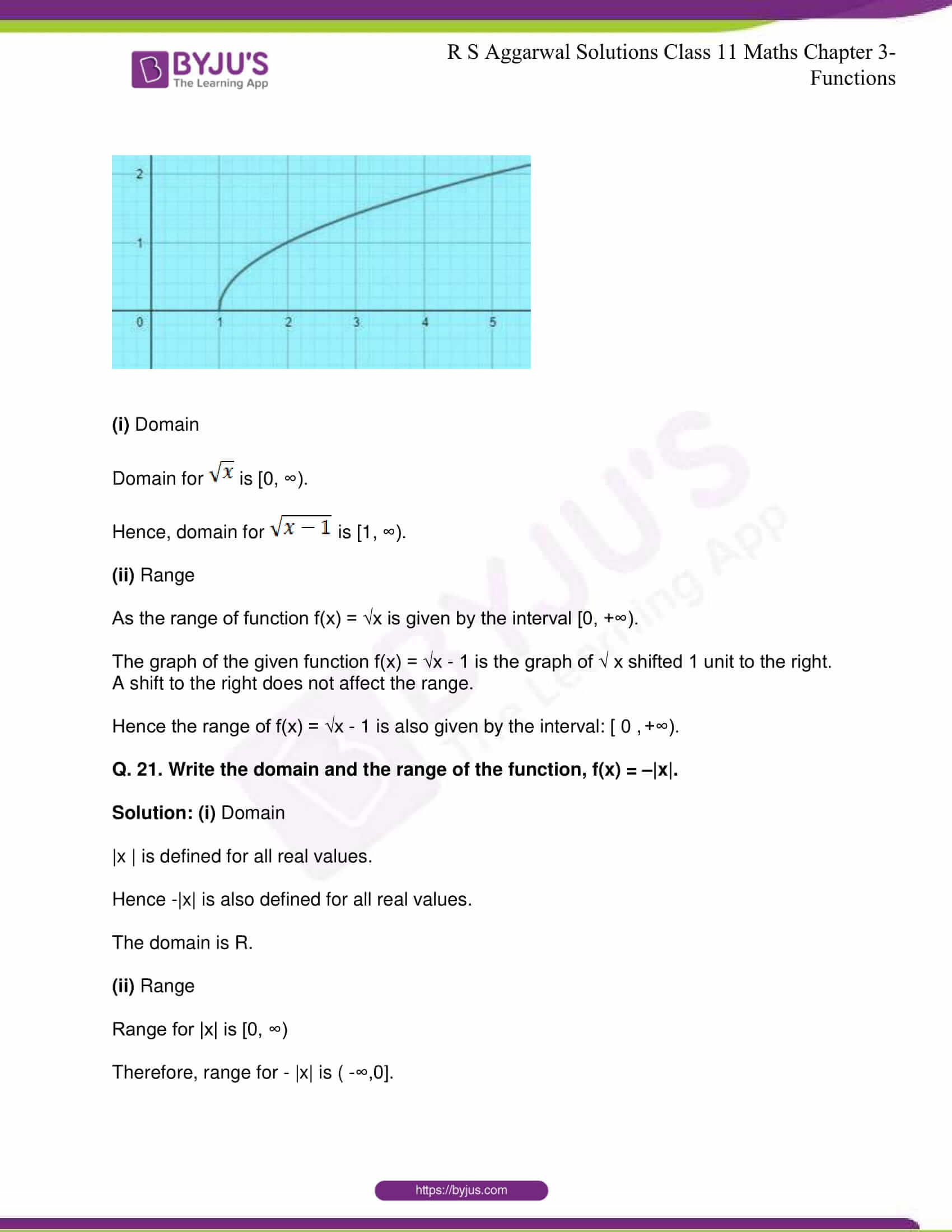## R S Aggarwal Solutions for Class 11 Maths Chapter 3- Functions

Chapter 3, Functions, consists of 6 exercises and the solutions given here contain answers to all the questions present in these exercises. Let us have a look at some of the topics that are being discussed in this chapter.

3.1 Function

3.2 Relation as a particular case of a function

3.3 Equal Functions

3.4 Inverse of Functions

3.5 Real Functions

3.6 Greatest Integer Function

3.7 Graphs of some standard real functions

3.8 Operations on functions

## R S Aggarwal Solutions for Class 11 Maths Chapter 3 – Functions

Let X and Y be two non-empty sets. Then, a relation f from X to Y is called a function, if every element in X has a unique image in Y. Here, in this chapter, students are taught about different Functions and how some standard real functions are represented on graphs. Read and learn Chapter 3 of R S Aggarwal textbook to get to know more about Functions. Learn the R S Aggarwal Solutions for Class 11 effectively to attain excellent results in the examination.